# Physical Science

## Edition 6.1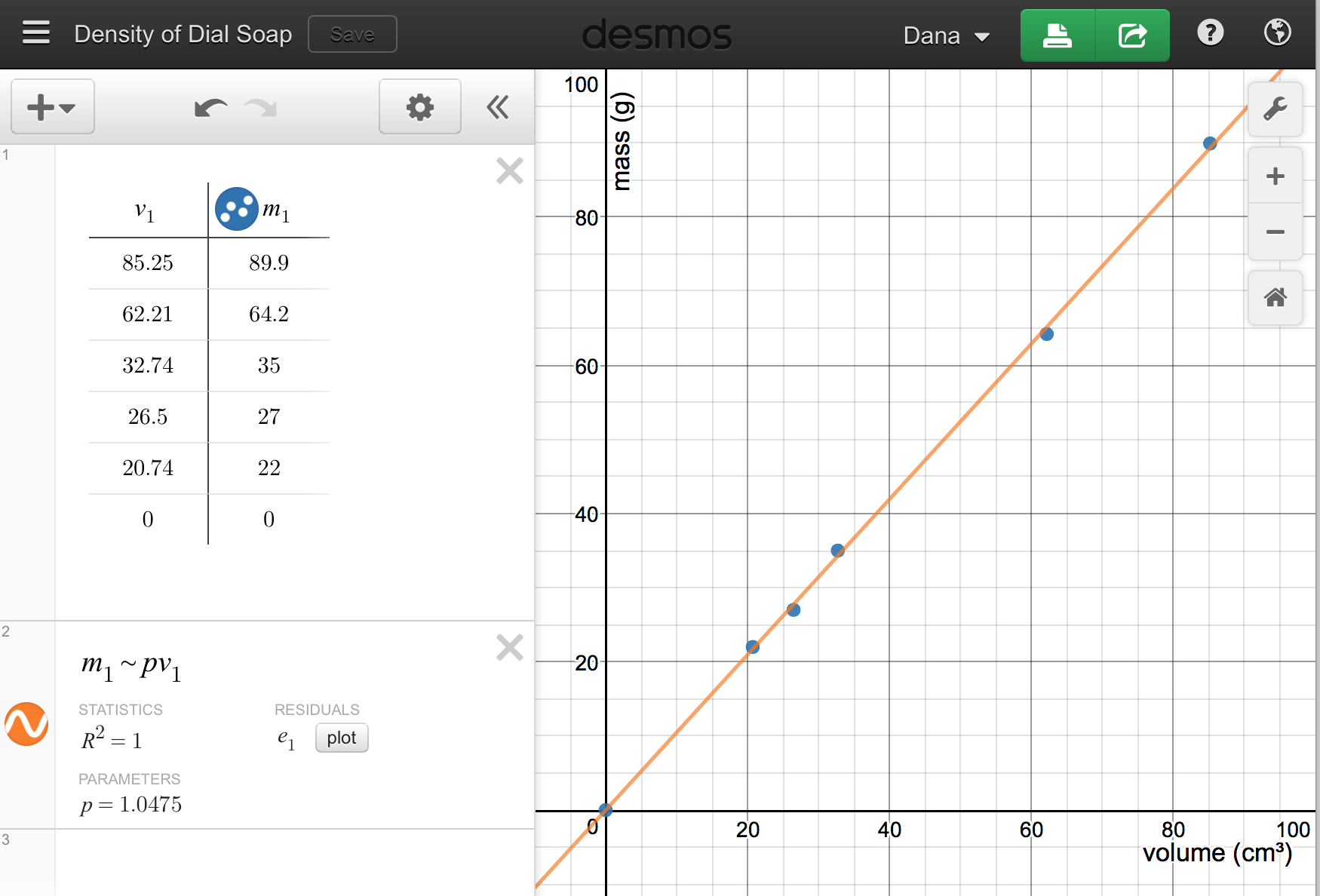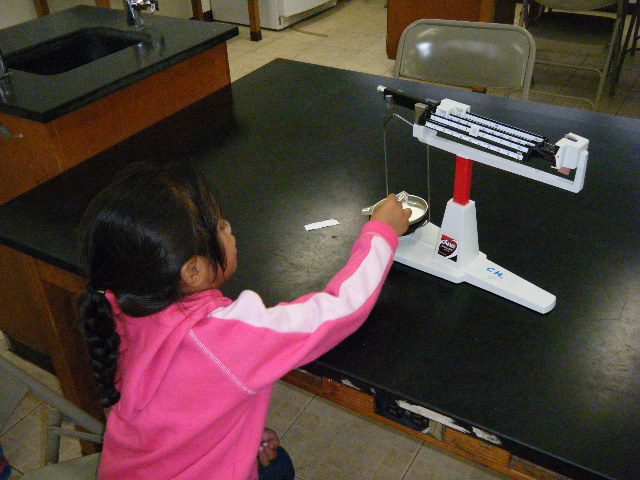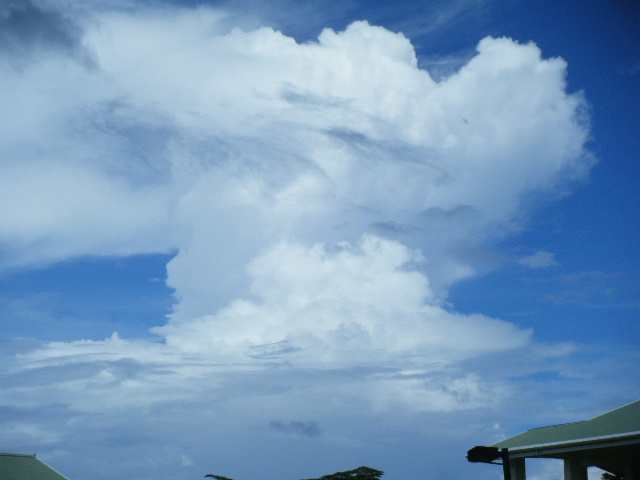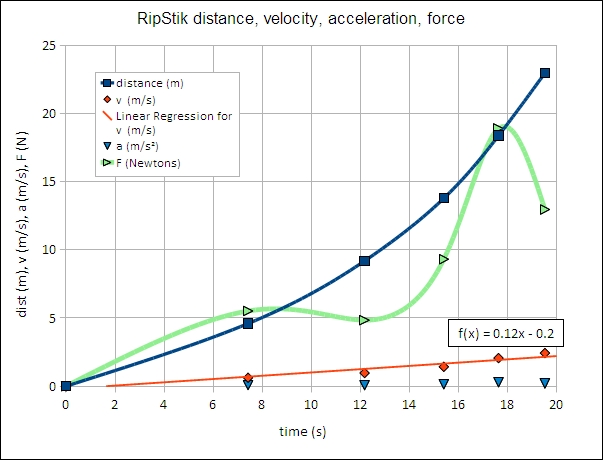Dana Lee Ling

## Physical Science

Dana Lee Ling
College of Micronesia–FSM

Pohnpei, Federated States of Micronesia

QC2020
Physical Science by Dana Lee Ling

Printed in the Federated States of Micronesia

# 003 Introduction

## Course focus

• the philosophy of the natural physical sciences
• determining the mathematical models that physical systems obey
• ability to communicate through writing the findings in the laboratories

## Course and text structure

The text is structured around weekly units and a Monday, Wednesday, Thursday, Friday meeting structure with Thursday being the laboratory day. Each unit is introduced with an activity or demonstration on a Monday followed by further development on Wednesday. The laboratory on Thursday is the focus of the week - the course focuses on "doing" science, science as a process rather than a collection of memorized. Friday the information from Wednesday and Thursday are usually synthesized in a weekly wrap-up followed by a quiz or test on the events of the week.

The text sections are numbered with three digit section numbers. The first two digits are the unit number. The third digit is a one for the Wednesday interactivity, two for the laboratory, and three for the rubric. A third digit of four may be used for quizzes and tests. Digits higher than four are used for "interstitial" inter-activities or laboratories caused by holidays.

## Instructional notes

This text is not a traditional content coverage text. This text also is not a step-by-step guide to leading this course. The course is based in constructivist theories of learning, the discrepant events science of the late Tik K. Liem, and cognitive psychology. The course builds from concrete observables to provide "conceptual" hooks on which the students can "hang" new concepts. The course is also informed by Kuhn's model of Paradigm shifts. The class is seen as a community of scientists constructing a shared set of understandings about the physical world.

While the course has constructivist portions, the course does not expect students to reconstruct the last 3000 years of natural philosophy and physical science. As Newton noted, seeing farther requires standing on the shoulders of giants. Those who first generated new understandings were considered brilliant geniuses. The course also includes demonstrations, lecture, and guided learning as appropriate. The laboratories are not devoid of all content, the discovery element is tempered with guidance and some directed exploration. The laboratories do seek, however, to avoid the formulaic "fill-in-the-next-blank" cookbook structure of traditional physical science laboratories. That said, there is a blurry line between a table that guides data collection and fill in the blank unthinking recording of data.

A tension also exists between a fully complete rubric and providing space for science as discovery and exploration. The analysis section of the rubrics can "telegraph" the answer expected and shift the laboratory from exploration to an exercise in trying to get the answer the student thinks the instructor wants the student to find. In some instances, especially after midterm, the analysis section of the laboratory marking rubric is underspecified. The instructor is expected to realize this and make their own modifications to the rubric as appropriate.

There are times during the laboratories when the instructor will turn the discussion lead over to the class. At such times the instructor is looking to the students to work out a way to handle the data. For example, in the conduction of heat laboratory the instructor asks the students to design an appropriate chart or graph with which to convey their findings. The instructor is not looking for a single right solution. There is not a single right solution. The right solution is whatever the community of scientists in the room decide is the best solution. The instructor might ask questions about the solution if the instructor is confused, but the instructor is only asking for clarification and is not suggesting that the solution is necessarily inappropriate.

Throughout the text there are instructional notes. These are intentionally kept in text used by the students. Students in the course include education majors destined one day to teach in the classroom. The design intent is that the text should be a guide to these students. The text also seeks to provide a sense of transparency with regard to how the course is being taught. Text books rarely include the techniques and methods of delivery. In this course the techniques and methods are intentionally exposed, they are important parts of the course content. For those familiar with the term, the text is like Bauhaus architecture: the functional form is exposed, the underlying structure is revealed.

The fourth edition shifted from providing the theoretic speed of sound and index of refraction of water to having the students obtain this information using WolframAlpha. The fifth edition added some alternate procedures for some laboratories. The sixth edition shifted to using the Desmos free online graphical mathematics system for tables, graphs, and analysis. The sixth edition also sought to improve mobile platform display characteristics to the extent possible. Other changes included the moving of rubrics to an appendix and deletion of older material not in present use by the course.

Spring of 2020 a pandemic coronavirus led to the course being redeployed as a online course. In places, notes concerning these changes have been included in this edition. The edition was bumped a decimal point to 6.1

The following is a sample laboratory report for student reference. The basic structure is an introduction, list of equipment used, procedure that was followed, a data table, a graph, an analysis of the data, and a discussion of results that acts also as a conclusion.

# Title of Laboratory (Heading level 1)

[Written using a past tense prose structure, include the names of the colleagues on the research team]

Lab group: Mayleen Mori, Jeffrey Joseph, Mary Robert, and Lynnleen Albert

In the introduction tell the reader what you are going to tell them. What were you measuring? Why were you measuring what you measured? What were the scientific goals of the laboratory? What were the questions being investigated? What might be the implications, if any, of the results? Who were you working with – what were the names of your team members?

## Equipment List (H2)

• Bulleted list
• Of equipment

## Procedure (H2)

[Written using a present tense action verb - noun clause structure]

1. Use sentences that start with an action verb followed by an object
2. Write the procedure like a recipe
3. Tell the reader step-by-step how to do the experiment

## Data table (H2)

Data table can be a screen capture from Desmos. Include a caption explaining the variables and units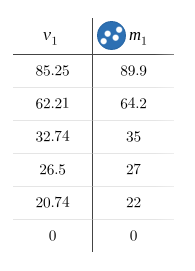v1 is the volume in centimeters³
m1 is the mass in grams

## Data graph

Data graph can be generated by saving the Desmos graph as an image from the Share button. Label the axes.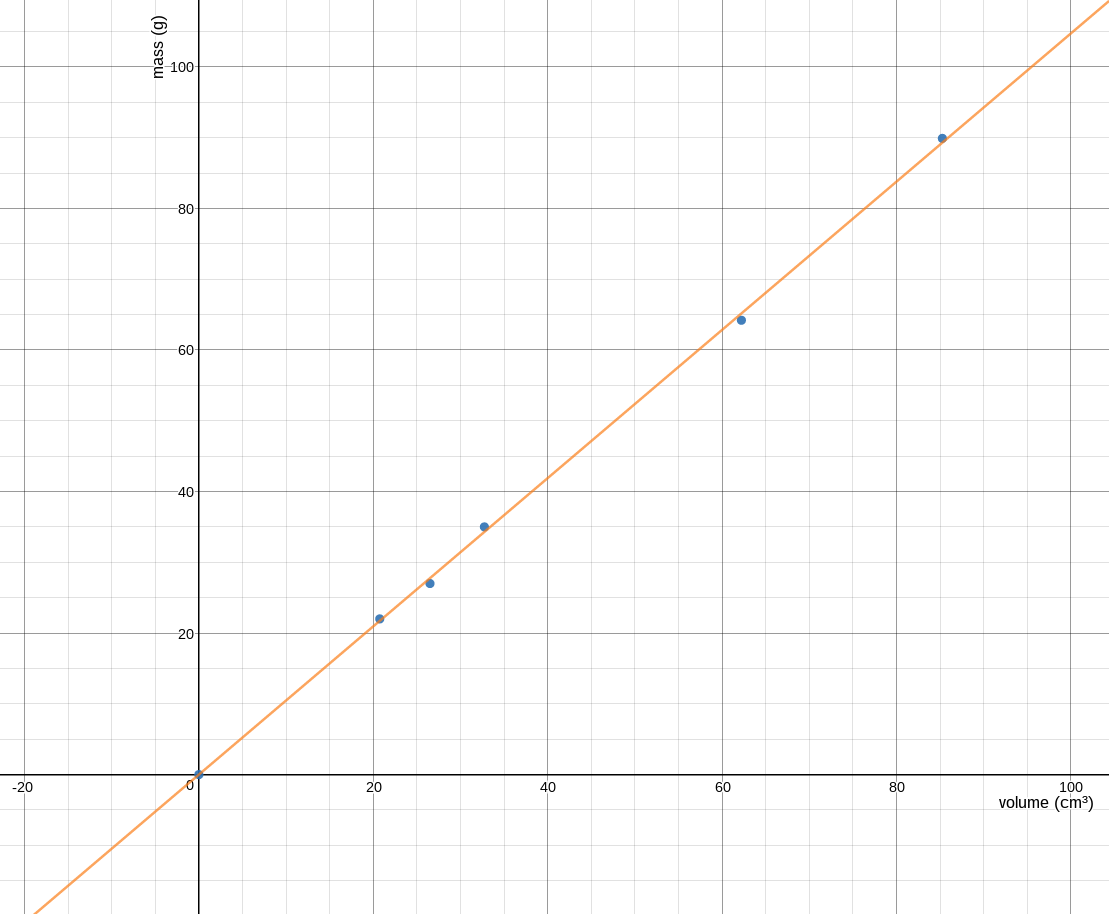## Numerical Analysis (H2)

A numerical analysis of the results based on the graph. The slope of the line and the units have some meaning in many laboratories and are often what needs to reported here. In laboratory one, for example, the slope is the density of the soap. The analysis is to report the name of the soap and the density of that soap. If there is a published value (measuring the acceleration of gravity g, the speed of sound, the index of refraction for water), include the raw error and the percentage error.

• Slope based on the analysis from Desmos including physical meaning (density, velocity) or constant being measured (g,...) and the units
• If there is a y-intercept, then report that with the units. Often there is no y-intercept.
• Raw error (if known)
• Percentage error (if known)

## Discussion and Conclusion (H2)

Tell the reader what you told them. Answer the questions posed in the introduction. Tell the reader whether you achieved your goals you laid out in the introduction. If a value was measured, cite the values reported in the numerical analysis (tell the reader again the value in the analysis and what that value means). Explain the physical meaning of the slope if appropriate. Explain the results of any predictions made based on the analysis. Discuss sources of possible error. Use correct grammar/syntax and vocabulary/spelling, organization, cohesion/coherence throughout the report.

# 007 Laboratory write-up notes

General notes and comments on common physical science laboratory problems

• Use past tense when referring to the events of the laboratory.
• Keep sentences short. Use a noun-verb-noun construction. Subject-verb=direct object. Do not splice together sentences using "and" or commas. One idea per sentence. Simpler is better. Less is more.
• Do not fear periods. Embrace them.
• Read the sentence out loud. Does the sentence make sense or nonsense?
• Present tense can be used in a procedure list. This is an exception to the general rule that past tense should be used.
• Write the procedure in the form command verb - direct object.
• Avoid starting every sentence with "And then..." Consider using a numbered procedure list if you are describing a procedure. For example:
1. Measure and record the length, width, and height of the soap
2. Calculate and record the volume.
3. Measure and record the mass of the soap.
4. Cut off a rectangular slab from one end of the soap and repeat the measurement steps until at least five measurements have been made.
• Ensure that subject and verb agree when using singular or plural subjects.
• Give credit to the other members of the laboratory group by including their names as seen in the sample laboratory report.
• Science laboratories are usually written in the third person. Occasional use of "I" or "we" might be necessary for clarity. In general, however, avoid using first person constructions. Use the equipment or the measurement as the subject of the sentence.
• Use a dictionary and look-up words you do not know.
• Record all raw data in tables, not just a final calculation.
• Where appropriate, provide a column of data labels in a table.
• Take care to use the appropriate chart type. A common mistake is to use a line chart. The course never uses line charts. The course most often uses xy scatter graphs.
• When writing up the conclusion, remember to discuss the goal of the laboratory. What was the point of the laboratory? If the laboratory generated a linear regression - a best fit line - what was the meaning of that line? What was the meaning of the slope? Was the value an expected value?
• Double space your laboratory reports: I need the spaces between the lines to write comments, make suggestions.
• Avoid the use of the pronoun "it" where you can. "I pounded the sakau using a stone in my hand. Upon striking repeatedly, it broke." What broke? The stone? The sakau? My hand? The word to which "it" refers is unclear.
• When you report a numeric value in your laboratory, include the units. Slopes and intercepts also usually have units.

# 009 Measuring space, time, and matter

Weather and time permitting, the first day of residential class can be an outdoor walk and talk about natural philosophy and science. The walk and talk develops the western concepts of space, time, and matter, and the concept of measuring these qualities. One approach is a historical, Aristotelian "no equipment" walk and talk. Another is one focusing on space, time, and matter without the historical components. Either approach can be taken by the instructor. Another more traditional option is to introduce the syllabus and administer a pre-assessment for later use course level assessment.

## Things to think about before class

• Space is about choice. Forward, backward, left, right, up, or down. You can go any direction. Up to you.
• Time leaves no choice. Relentlessly marching ever forward into the future. There is no going back. No left time, no right time. No up, no down. Only one direction. No choice.
• Matter is the mystery. Matter has no direction. Matter has no forward, no backward. No left. No right. No up. No down. Matter simply exists.
• Space is the questions how close, how far, which way, where am I, how high, how deep, how wide, how long. Space has lots of questions.
• Time is the questions when, how old, how young. Time has only few questions.
• Matter is the question how much. Matter has the fewest questions.
• Space is near, far, over there, here, on, over, under, above, below, big, small, narrow, tall, short, wide, in front, in back, across. Space has many descriptors.
• Time is now, never, sooner, later, forever, immediately. Time has a only few descriptors.
• Matter is a lot, a little. Matter has the fewest descriptors.

Everything else is pure energy.

Why?

## Aristotle

Aristotle is acknowledged to be the founding father of natural science in Western culture. Aristotle introduced the idea of doing experiments in order to understand the natural world, although his experiments were more often thought experiments rather than actual physical experiments. For Aristotle, natural science was the same as natural philosophy. Science was a branch of philosophy.

Aristotle is said to have taught while walking around outside at the Lyceum, leading to the students referring to themselves as belonging to the peripatetic school. Aristotle walked and taught what he considered branches of philosophy including natural philosophy.

Peripatetic: To walk around (peri) the patio garden (patetic).

## Fundamental qualities of the natural philosophy of science

• space
• time
• matter

### Space

Space consists of three dimensions. Three dimensions means three directions. Think of the directions as forward-backward, right-left, up-down. Backward, left, and down are not three new directions. Backward, left, and down are simply the opposite of forward, right, and up. If forward, right, and up are positive directions, then backward, left, and down are negative directions.

Consider an ant moving on a tiled floor. The location of the ant can be given by counting the number of tiles along the wall from a corner of the room, and then the number of tiles from the wall to the ant. Two numbers would locate the ant on the floor. No more numbers would be needed. An ant that was seven tiles along the wall, and three tiles away from the wall could be said to be at (7,3). Note that this system usually presumes the walls are at right angles to each other.

If I want to describe a location above the ant on the floor, then I need a third number. I need the number of tiles above the floor. This is a third right angle. A third perpendicular direction. If the location is four tiles above the ant, then (7, 3, 4) would locate the ant. Written as variables these would be (x, y, z).

This method of describing locations in space is called Cartesian coordinates. René Descartes developed Cartesian coordinates in the early 1600s. Descartes is also credited with introducing the idea of using x, y, and z as variables and a, b, and c as constants in algebra.

### Time

Time has only one direction, forward. Time, as we experience time, cannot be stopped or reversed, not with current knowledge and technology.

### Matter

Matter is the amount of stuff in an object. Matter has no direction. Only when matter moves through space can one speak about a direction. The direction is the result of movement, not a property of the matter.

## Motion: combining qualities

Steps and beats: an introduction to thinking about space, time, and measurement.

To walk requires making steps. Steps move one through space. Steps also take time. One cannot move from one place in space to another in zero time. Movement requires changing one's location in space and time. Space and time are intertwined. Time itself is often measured as a movement through a distance on older dial-style clocks with moving hands. Today's digital watches and timers no longer show us the movement, but time is still deeply connected to space.

We can speak of the number of steps covered per beat. Per refers to division. Steps ÷ beats is a measure of space per unit time. Steps per beat can also be called a speed. In this class speed and velocity are treated as being the same.

There is a difficulty with steps and beats. Each person will measure a different number of steps for a given distance in space. Each person will also measure a different number of beats.

## Other combinations and possibilities

Motion requires the qualities space and time. The words speed and velocity both refer to motion. Other concepts may combine other qualities. Density is only possible with space and matter. Momentum will require matter, space, and time - all three qualities.

Some measures will differ in their exponent. Space squared is called area. Space squared, area, appears in the formula for pressure. Space cubed is called volume. Space cubed, volume, appears in the density formula. Time squared appears in acceleration and energy calculations. Time squared is actually an area measure of time.

Some measures differ in the scale on which the quality acts. The atomic scale vibration of atoms in solids is responsible for what is called the temperature of the solid. Temperature is related to motion, which is change in space and time. The units of temperature, however, do not appear to be units of speed.

Some measures are simply new vocabulary applied to the three qualities. Wavelength is a specific distance measured along a wave. Frequency is a rate at which something repeats measured in repeats per unit time. Angles are measures of rotation in space.

## Energy

While energy can be expressed by combining units that measure space, time, and matter, energy is not space, nor time, nor matter. Energy is perhaps the hardest to describe. Among other complications is that in physical science there are different forms of energy. There are special terms to describe these different types of energy. The energy that a moving object possesses is called kinetic energy. The energy possessed by hot objects is called thermal energy. The sun produces solar energy. Gasoline and kerosene contain chemical energy.

Instructional notes on the peripatetic/Aristotelian start option: Using this option the instructor intentionally has no papers, no pens, no watch on for this course. The instructor is limited to that which a theoretical Aristotle might have had access to on a walk around the garden patio. The natural world is the science classroom. Science is not a laboratory, science is a way of thinking about the physical world.

Another option is to focus on space, time, and matter, introducing these concepts along with the three dimensions of space, one of time, and arguably none for matter. This approach would also include examples of space, time, and matter.

# Activity 00 Sticks & Stones

Instructional notes: During the summer term this has sometimes functioned as a lead off laboratory. Finding meter sticks is a weather dependent activity. The class goes out to obtain "meter sticks" only if conditions permit.

## Questions

Can you count off seconds? Can you make a meter stick from a stick? Can you find a one kilogram stone?

This is a lightweight introductory lab designed to be deployed during the early days of the term when the class may still be adding and dropping students. The lab is also designed to help prepare an education major for work in a rural, village school that might not have any scientific instruments.

Curricular note: This particular mini-lab lite is more often deployed in the summer session due to the structure of the summer schedule.

## Time: Keeping the beat

• Fundamental measure: second
• Gather data. Average.
• Calculate the percentage error.
• Repeat on different period.

## Space: Measuring in meters using meter stick tree meter sticks

• Have the students use scientific meter sticks to determine the span of one meter from the tip of their middle finger to the opposite side of their body. They should also determine what part of their abdomen/hip/upper leg is one meter above the ground.
• Leaving the meter sticks behind, head to the nearest meter stick tree forest.
• Find or make a meter long stick.
• Lecture-demonstration using children's letter blocks to illustrate length, width, height, surface area, volume.
• Cover 1 × 1 × 1, 2 × 2 × 2,... for the five quantities above. Continue beyond the number the blocks up to at least 8 × 8 × 8 to demonstrate that volume eventually grows larger than surface area and grows more quickly.
• Measure the length, width, and height of the classroom using the meter stick tree meter sticks. Calculate the surface area and volume.

## Mass: Stones

• Pass around a one kilogram metal mass and a one kilogram bag of sugar or other commodity. Are they the same mass?
• Mass the two objects. Discuss results
• Homework: bring in a one kilogram rock, or, if no rocks, then other objects.

# 011 Measurement in physical science

In regular terms which begin on a Monday, a pre-assessment of mathematical skills is administered on Monday, reviewed on Wednesday. Wednesday is then used to introduce density using cubes of differing materials. Laboratory one occurs on Thursday. The material in 011 is essentially omitted under this structure, with metric concepts introduced on an "as needed, just-in-time" basis. During planning for the online fall of 2020, measurement was returned to the curriculum and the first laboratory was delayed until the second week of class.

Physical science has special terms used to describe measurements.

Primary measurements: A quantity that is directly measured using an measuring device or instrument. Measuring devices might include rulers, stopwatches, mass balances, protractors, and thermometers.

Calculated measurement: A quantity calculated from a mathematical combination of primary measurements.

All measurements are expressed as the combination of a number and the units of measure. Whenever I speak about a measurement in science I must say both the measurement and the units. Thus I say "Five centimeters" or "Three volts." Number and units. Together forever. Leave either one out, and the answer is incomplete and incomprehensible. Wrong.

There are two types of units of measure in science.

Fundamental units: In physical science measurements of length, mass, and time use the units of either meters-kilograms-seconds (mks) or centimeters-grams-seconds (cgs). Physical science uses only metric measurement systems.

Fundamental units measure space, time, and the amount of matter contained in an object. In laboratories either the cgs or the mks system are used.

Derived units: Measurements expressed using arithmetic combinations of fundamental units. For example, volume is derived from multiplying together three independent measurements of length. To avoid confusion these three measures are usually described as length × width × height. All three are still measures of length. Length is the fundamental measurement. Volume is the derived measurement.

Density is another derived measurement. Density is derived from the mass divided by the volume.

Yet another example of a derived unit is the metric measure of liquid volumes, the liter. A liter is defined to be 1000 cm³. The liter is defined using a fundamental unit of length.

## Limits of measurement

All measurements have limits in terms of their accuracy. There are terms used to describe these limits.

Uncertainty: the limit of our measuring tools for a single measurement, our uncertainty based on the smallest measurement our tools can accurately make. For a ruler marked in millimeters there is always at least a half a millimeter of uncertainty, often more. Some electronic devices tell you the uncertainty. Global positioning satellite (GPS) receivers usually provide an estimated uncertainty.

Precision: the average variation in multiple measurements of an experiment usually expressed using the standard deviation.

Error: the difference between the measured result and the actual value. The actual value is usually unknown, hence the error is never truly known.

## Quantifying the fundamental qualities

To "quantify" means to attach a numeric value to something. Words that are associated with quantifying space are distance, length, width, height, radius, and diameter.

Words that are associated with quantifying time are duration, interval, and frequency.

A word that is associated with quantifying matter is mass.

## Measuring the fundamental qualities

In this class we will use only the metric system to measure the fundamental qualities. To measure space we will use meters or centimeters. To measure time we will use seconds. To measure mass we will use grams or kilograms. .

QualityQuantityFundamental unit of measurement
MKSCGS
SpaceDistance, length, width, height, radius, and diametermetercentimeter
TimeDuration, interval, and frequencysecondsecond
MatterMasskilogramgram

## Getting to know one's personal qualities

The following is an optional exercise done in an in residence class setting. In class you will determine your height in meters and your mass in kilograms. For homework you will work on your age in seconds. Include both the number and the units.

Space: height in meters: ______________________ Matter: mass in kilograms: ______________________

### Body Mass Index

A useful use of your height and mass is that these two numbers can be used to calculate your Body-Mass index. If your body mass index is over 26, then you are considered to be "overweight" for your height. This does NOT necessarily mean "too much fat." If one is a weightlifter, one can have a BMI over 26. Note that 26 is a recommended cut-off for Pacific islanders. For non-Pacific islanders the cut-off is 25.

Calculate your BMI by dividing your mass in kilograms by the square of your height in meters.

BMI = (mass in kilograms)/(height in meters)² = __________________________ kg/m²

The units of BMI, kg/m², are called derived units. Derived units are combinations of fundamental units of measurement.

### How old are you in seconds?

Calculating your age in seconds is more complicated than simply stating your age in years. This exercise outlines how to set up a spreadsheet to calculate your age in seconds. You will also be able to determine the week day on which you were born. The intent of this exercise was to also introduce students to using functions in spreadsheets to make calculations. With the shift to using Desmos, the need for facility with spreadsheets was lost and this exercise is left in the text as a remnant.

Spreadsheets calculate your age in days. Days are not a metric unit. To convert days to seconds requires multiplying by 24 hours in one day, 60 minutes in hour, and 60 seconds in one minute.

Days × 24 hours/day × 60 minutes/hour × 60 seconds/minutes = seconds

RowAB
11/31/2000=WEEKDAY(A1)
2=NOW()
3=A2-A1
4=A3*24*60*60
1. Put your birth date in cell A1
2. Type the function

=NOW()

in cell A2. Note that there are no spaces between the parentheses for this function. This function returns the current date and time.
3. Type the formula =A2-A1 in cell A3.
4. Type the formula =A3*24*60*60 in cell A4.
5. Type the function

=WEEKDAY(A1)

in cell B1. This will calculate the day of the week for the date in A1. The function returns a number between 1 and 7 inclusive. The days are Sunday=1, Monday=2,... Saturday=7.

The value in A4 is your age in seconds at the time you entered your formulas.

I am ____________________________________ seconds old.

#### Akan Day Name

At one time I lived in West Africa. Among the Akan your name at birth depends only on your gender and the day of the week. These names are called day names.

WeekDayDayFemaleMale
1Sunday AkosiaKwasi
3Tuesday AbenaaKwabenaa
4WednesdayAkua Kwaku
5Thursday Yaa Yow
6Friday Afua Kofi
7Saturday Ama Kwame

#### Converting days to years

At present you can use the conversion 365.25 days = one year. The 0.25 is why we have a leap year with a 29 February every four years. Leap years are divisible by four.

The actual conversion is 365.2422 days = one year. The actual conversion would have to be used for dates prior to 1900. In century years not divisible by 400, including 1900, there is no leap day. The year 2000 was divisible by 400, so 2000 was a leap year and included 29 February. Thus if you were born after 1900, 365.25 is the correct conversion. At least until 2100 when we skip a leap year again.

#### How many seconds do you have remaining?

Once you know how old you are in seconds, check to see how many seconds you have left to live. Go to the DeathClock. You will need the BMI number you calculated above.

How many seconds do you have left to live? _____________________________

Is the DeathClock real? Is that how long you really have left to live? Why or why not?

# Alphabet Soup

Physical science can be confusing, especially as the same letters get reused with different meanings in different formulas. In laboratory one, m is the slope in the algebraic formula y = mx and m is the mass in the physical science formula density ρ = mass × Volume V. The following table is intended to help sort out what a letter might mean, can mean, in different contexts. One of the most important distinctions is the reuse of letters for variables and units. The letter g as a variable is the acceleration of gravity at the surface of the earth. The letter g as a unit is the unit of mass known as a gram. This table includes both variable meanings and unit meanings.

LetterVariable or constantUnitEquations with variable
Aamperes (current)
aacceleration $a= Δѵ Δt$
BMIbody mass index $BMI= m h²$
cspeed of lightcenti- prefix: cmE = mc²
ddistance d = ѵt, d = 0.5at², d = 0.5gt²
Δ%percent difference $Δ% = (observed−predicted) predicted$
Fforce $F= Δp Δt$, F = ma, F = −kx
ffrequency ff = 1 ÷ τ
hheight, Planck constant
i current, $−1$
gacceleration of gravitygrams d = 0.5gt²
GPEgravitational potential energy GPE = mgh
KEkinetic energyKE = 0.5mѵ²
kHooke's constant,
F = kx, $y = − ( k r2 ) x2 + k$
llengthliters (liquid volume)
λwavelengthѵ = λf
mmass or slopemeters m = ρV, $slope m= (y2−y1) (x2−x1)$
ΩOhms (Resistance)V = iR
PPowerP = iV
pmomentump = mѵ
ρdensitym = ρV
RResistanceV = iR
sarc lengthseconds
Ttemperature
ttime
τperiodτ = 1 ÷ f
ѵvelocity $v= Δd Δt$, ѵ = at, ѵ = λf
Vvolume, voltageVolts V = lwh, V = iR
wwidthV = lwh
Wwork (energy)Watts (power)

# 012 Lab 01: Mathematical models and measurements in Physical Science

Standing on a carved soap box: density of soap

## Question

• Is there a linear relationship between the volume and mass for a material such as soap?
• What is the density of a particular brand of soap
• Based on the density, will a particular brand of soap float or sink in water?

## Goals

• Determine the nature of the mathematical relationship between the volume and mass for a substance.
• Introduce different types of mathematical relationships and models.
• Introduce a decision flow chart to make choices as to the nature of a mathematical relationship between variables.
• Determine the density of soap
• Based on the density, determine whether a particular brand of soap floats or sinks in water

## Equipment

• Boxes of hand soap including Ivory and non-Ivory soap
• Meter sticks
• Mass balances
• Soap carving implements

## Introduction

In this laboratory the mathematical relationship between the volume of a squared-off chunk of soap and the mass of the soap is explored. One result of this exploration will be a calculation of the density of the soap.

In physical science a "relationship" means how one variable changes with respect to another variable. This change is described using mathematical equations. Math is the language in which physics is "spoken."

Galileo Galilei spoke of science as "natural philosophy," a term the Greek philosopher Aristotle also used for science. Galileo noted that the universe around us is "written" in the language of mathematics:

La filosofia è scritta in questo grandissimo libro, che continuamente ci sta aperto innanzi agli occhi (io dico l' Universo'), ma non si può intendere, se prima non il sapere a intender la lingua, e conoscer i caratteri ne quali è scritto. Egli è scritto in lingua matematica,...

which might be translated as:

[Science] is written in that great book which ever lies before our eyes — I mean the universe — but we cannot understand it if we do not first learn the language and grasp the symbols, in which it is written. This book is written in the mathematical language,... Galileo Galilei

Physicist Freeman Dyson, who studied subatomic physics in the 20th century, echoed Galileo:

For a physicist mathematics is not just a tool by means of which phenomena can be calculated, it is the main source of concepts and principles by means of which new theories can be created... ...equations are quite miraculous in a certain way. I mean, the fact that nature talks mathematics, I find it miraculous. I mean, I spent my early days calculating very, very precisely how electrons ought to behave. Well, then somebody went into the laboratory and the electron knew the answer. The electron somehow knew it had to resonate at that frequency which I calculated. So that, to me, is something at the basic level we don't understand. Why is nature mathematical? But there's no doubt it's true. And, of course, that was the basis of Einstein's faith. I mean, Einstein talked that mathematical language and found out that nature obeyed his equations, too. – Physicist Freeman Dyson

A core concept in the physical sciences is the idea that physical systems obey mathematical equations. The mathematical equations are also called mathematical models or mathematical relationships.. If two variables are related by a mathematical equation, then predictions can be made about that physical system. Physics, engineering, and chemistry all depend on predictable results. When something is done in the same way twice, the same result should occur.

One way to begin to find the mathematical equation that relates two variables is to make an xy scatter graph. The graph will help indicate whether a relation exists between the variables. The graph will also help us determine the nature of the relationship. By nature we mean whether the relationship is linear or non-linear.

If the relationship is linear or non-linear, then the system behaves in a predictable manner. Given the value of one variable, we can predict the value of the other variable. In this class we will only try to make predictions for linear relationships. Non-linear relationships are also predictable, but the mathematics is beyond the scope of this course.

If the relationship is linear, then the equation has the form y = startValue + Ratex. You may be more familiar with the form y = mx + b. In physics the slope m is often a rate of change and the y-intercept b is often a startValue. The y-intercept is not always the startValue, but for many systems the y-intercept, where x = 0, is a starting place for a system.

If we find that a relationship does form a roughly straight line, then we will try to find the slope and intercept.

The following graphs show the different types of relationship that might exist between two variables.

To decide on whether a relationship exists between two variables, start with an xy scattergraph. Looking at the graph, consider the following questions:

• Does the (x,y) data form a roughly straight line?
• Does the (x,y) data form a smooth curve?
• Does the (x,y) data form no pattern, scattering randomly on the chart?

If the data points form roughly a straight line, then use the spreadsheet function SLOPE and INTERCEPT to obtain the slope and intercept. The slope and intercept can also be displayed on the graph directly. If the data points form a smooth curve, then the relationship is a non-linear relationship. Determining the nature of the relationship may or may not be possible for us in this course. If the data points form no pattern, then there is no relationship between the variables being studied.

The following decision flow chart is a map of the flow of decisions described above.

Desmos graphing calculator is used to find mathematical models for data. If an instructor chooses to use spreadsheets, then the following two spreadsheet functions calculate the slope and intercept for any linear relationship. Note that the y-data is first in the formula, followed by the x-data. These functions are only necessary

=SLOPE(y-data,x-data)

=INTERCEPT(y-data,x-data)

If the data points form a line, then we can draw a line through the points. This line that best goes through the data points is called a best fit line, trend line, or a linear regression.

Note that because of uncertainty in primary measurements and error, data is considered linear even when the data falls only roughly along a straight line. The data does not have to fall exactly in a straight line. The issue of how close data comes to a straight line is part of the study of correlation in statistics.

## Volume, mass, and density

One of the first persons to base hypotheses on experiments was William Gilbert (1544 - 1603). Gilbert noted the need for experiments to be repeated to ensure that the result is consistent. He also cautioned those that repeated his experiments to "handle the bodies carefully, skillfully, and deftly, not heedlessly and bunglingly." The Scientists, John Gribbin, page 71. Library, Amazon.

So too should you measure carefully, with skill, patience, and attention to accuracy. Measurements should be repeated to ensure an accurate result.

In this laboratory you will explore the relationship between volume and mass for soap. You will measure the length, width, and height of a soap slab, calculate the volume of soap slab, and measure the mass of the soap slab. A graph of volume versus mass will be made. If a relationship is found, then the mathematical equation of the relationship will be calculated. Remember, "a relationship" simply means that a pattern such as a line or curve is formed on an xy scattergraph of the two quantities. That relationship is usually expressed as a trend line. The slope of the trend line will have a physical meaning.

Run a demonstration density calculation using the wood alphabet block and a lead cube. Ask the class to predict the result prior to putting the object in water.

The equation:

$\text{mass}=\text{density}×\text{volume}$

is of the form

$\text{y}=mx$

where volume is x, mass is y, and the density is the slope m.

The theory behind this laboratory is that the slope of the linear regression line for the volume versus the mass is the density. According to theory, density should be the same for a substance no matter whether the piece is large or small. If this is true, then a plot of volume versus mass should produce a straight line with a slope equal to the density.

In this laboratory the units of mass are grams, the units of volume are cubic centimeters, and the units of density are grams per cubic centimeter.

Length, width, height, and mass are fundamental quantities. Both volume and density are "derived" quantities.

### Procedure

• Create as large a rectangular slab of soap as you can from the soap bar.• Measure the length, width, height and mass of the soap slab.
• Create smaller slabs from the soap bar and repeat the measurements.### Alternate procedure for use without a mass balance

The following procedure was developed for use at home by students.

1. Record the mass of a rectangular bar of soap as reported on the packaging.
2. Measure the length, width, and height of the soap
3. Calculate the volume.
4. Cut the soap exactly in half.
5. Repeat the measurements, presuming that the mass is also halved.
6. Cut the half bar in half again.
7. Repeat the measurements again, presuming that the mass is one quarter of the original mass.

### Data tables

Brand of soap used:

Length (cm)Width (cm)Height (cm)v1m1

v1 is the volume in cm³
m1 is the mass in grams

Note: Do not put units into the table. In Desmos enter only the v1 and m1 columns.

### Graph

If using Desmos, entering v1 versus m1 will generate a graph. Add labels to the axes of the graph and adjust the domain and range minimum and maximum from the graph settings (monkey wrench icon). If using alternate software for graphing, follow the directions of the instructor.

### Analysis

The analysis is to determine the density of the soap from the slope of the line. mass = density × volume or m = pV where Desmos uses m1 for m and v1 for V. Use the Desmos regression model m1~pv1 where p is the slope of the line and thus the density of the soap. Report the results of your analysis above. Include the slope and the units for the slope, grams per centimeter cubed. Explain the physical meaning of the slope as the density of the soap. Note that the units for the y-intercept are not the same as the units for the slope. When reporting the y-intercept, the units are the same as those on the y-axis, grams.

### Discussion of results

Report the mathematical relationship of volume and mass, including both the slope and intercept. Discuss the physical meaning of the slope.

Mathematical relationships allow predictions to be made.
The density of water is close to 1.0 g/cm³
If the density of an object is greater than one, then the object will sink.
If the density is less than one, then the object will float.

In the discussion note whether the soap would be predicted to float or sink based on the density (which in turn is based on the slope of the line through the data). In the discussion include whether the soap floated or sank when put in water. Did the predicted behavior happen?

Instructional note: If a separate computer laboratory is being used, bring some of the remaining chunks of soap to the computer laboratory along with a large beaker of water. Have the students use their density results to predict whether their soap floats or sinks. Reinforce the link between slope and density. Then test the prediction. Did the soap know the answer?

### Laboratory Report

Use Desmos and a word processor to write up the laboratory report.

#### Making a table and graph with Desmos

Desmos can be accessed from a browser on a computer or via an app on a smart phone. Add a table. Change the first variable to v1 for volume. Change the second variable to m1 for mass.

Note that the image in this section is from Desmos running on a smart phone, generated by entering data directly into Desmos during the laboratory. This provides immediate graphical feedback during the laboratory procedure.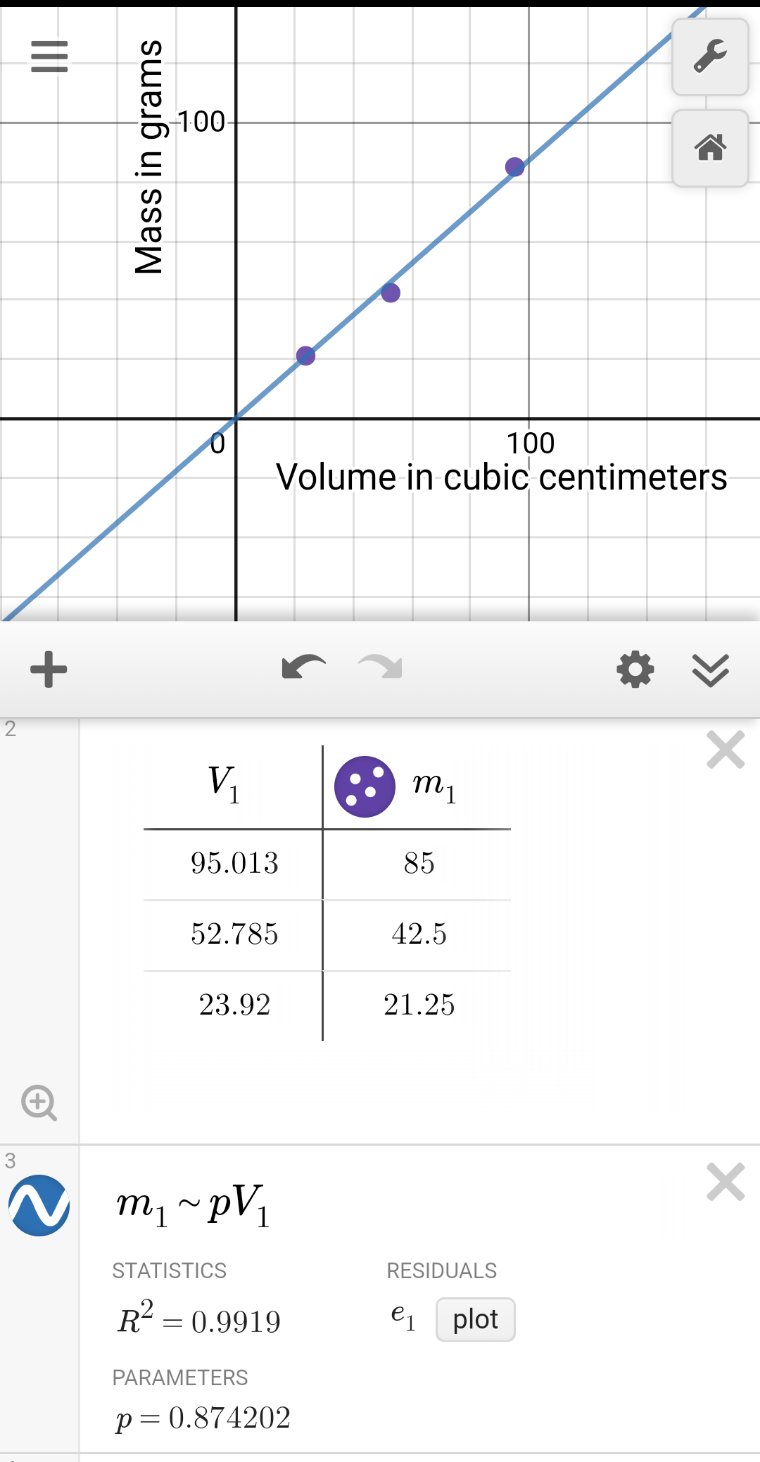V1 is the volume in centimeters³
m1 is the mass in grams

To obtain the slope, enter the equation:
m1~pV1
Note that p is the slope which is also the density of the soap.

Note too that Desmos is case-sensitive. If a lowercase "m" is used for the mass and an uppercase "V" is used for the Volume, then the regression formula must also use the same cases. Data for the above image was produced using the alternate procedure where the mass is known only from the package labelling and only two cuts are made in the soap. This variation was designed to be deployed in an online environment.

If working in a browser with Desmos, save an image of the graph. Screen capture of a region of the screen can be used to capture the table and analysis. Remember to put a caption under the table labeling the variables and units. For Microsoft Windows, screen capture of a region on the screen can be accomplished with the Snipping Tool. For Apple MacOS use command-shift-4 and select the region to capture. On ChromeOS use control-shift-switch window and select the region to capture. On Ubuntu use shift-print screen and select the region to capture. For guidance, refer also back to the sample laboratory.

# 021 Motion

Note that density was matter divided by space. In that formula space was cubed. Full three-dimensional space. In the world of physical science the degree is the number of dimensions.

Motion is space divided by time. For motion, space is not squared nor cubed. Space is to the first degree. Linear. One dimensional. Motion has a single direction.

Measuring motion requires measuring both space and time. Space is measured using meters or centimeters. Time is measured using seconds. Motion in a direction is called velocity in physical science. Motion without reference to a direction is called speed. Working with motion in a direction usually requires working with vectors and trigonometry. In this section we will restrict ourselves to straight line motion. In straight line motion velocity and speed are the same thing.

$\text{velocity}=\frac{\text{distance}}{\text{time}}$

If the distance is measured between two points in space, and the time is measured between two points in time, then the above formula is sometimes expressed as "the change in distance" divided by the "the change in time." The Greek letter delta (Δ) is used for the words "the change in."

$\text{velocity}=\frac{\Delta \text{distance}}{\Delta \text{time}}=\frac{{\text{distance}}_{2}-{\text{distance}}_{1}}{{\text{time}}_{2}-{\text{time}}_{1}}$

The above formula is mathematically the same structure as the formula for the slope of a line between two points.

$\text{slope}=\frac{\left({y}_{2}-{y}_{1}\right)}{\left({x}_{2}-{x}_{1}\right)}$

In physical science the relationship between distance, velocity, and time is often algebraically rearranged and written:

$\text{distance}=\text{velocity}×\text{time}$

A rolling marble passes 0 centimeters (cm) at a time of 1.5 seconds (s). The marble passes 100 centimeters at a time of 3.5 seconds. Calculate the velocity of the marble.

Alternate Monday introduction: A caster board can be ridden past columns with elapsed time recorded in seconds as the board passed the columns. The distance from the start of the run to each post was also measured. As homework the students were to graph the time versus the distance and determine the slope of the line.

A video introduction was produced for remote use fall 2020.

A graph of duration versus distance is a graph of time versus space. The linear relationship distance = velocity × duration is a relationship between time and space.

On a graph of duration versus distance one gets a straight line if the speed is constant. The actual path over the ground might be straight or curved.

In graph one on the left above, time is plotted against space in the form of duration in seconds against distance in centimeters. The object, for example a rolling ball, is moving at a constant speed. The graph on the right is a "bird's eye view" of a ball rolling in a parking lot. The ball may roll straight, left, or right. The speed of the ball along the path is not displayed by graph two. The curve seen is the curvature of the ball along the ground.

Note that in vector physics velocity is always a speed in a particular direction. Change the speed, and the velocity changes. Change the direction, and the velocity also is said to change. A ball moving at a constant speed on a curved path is changing directions. The speed is staying the same but the velocity is changing. This is the difference between speed and velocity. Speed has no specified direction, velocity has to take into account the direction.

Graphs of time versus space, duration versus distance, do not tell us the direction of motion. Time versus distance depicts only the speed as the slope of the line. If the slope is changing, then the speed is also changing.

Graph three shows a ball rolling with the speed of the ball changing.

Note that the above graph says nothing about the direction that the ball is rolling. The information is only about how far a distance the ball has moved from zero centimeters in how long a duration of time in seconds.

## Interactivity: How slow are you?

This optional activity is also an opportunity to introduce GPS receivers, their operation, the screens displayed, and differences among the GPS models in use in the class. This introduction is important to activity 071 later. The GPS can be used to obtain walking speed, running speed. A sports ball radar gun can obtain thrown ball speeds, speed of cars along the road.

Using instruments that measure time and distance, determine your speed. Weather cooperating, as a class we will go outside and use global positioning satellite receivers to determine how slow we saunter, stroll, walk, stride, jog, or run.

# 022 Lab 02: Linear motion

Rolling balls gather no moss

## Questions

Is there a linear relationship between the time and distance for a ball rolling on level ground? How does this relationship change with changes in speed?

## Goals

1. Determine the nature of the mathematical relationship between time and distance for a ball rolling at a constant speed
2. Work in "Newton's" (outdoor) laboratory
3. Demonstrate a lab that can be done with a minimal of equipment
4. Gather data in a manner that generates a single x-axis and multiple y-axis values while keeping the time on the x-axis and the distance on the y-axis. Spread sheet software cannot handle multiple x-axes.

## Equipment

• Inflated rubber kick or four-square balls
• Stopwatch or stopwatches/chronographs
• Metric tape measures or surveyor's wheels
• Sidewalk chalk if needed

## Introduction

This laboratory explores the relationship between time and distance for an object moving at a constant velocity. As noted in the first laboratory, in physical science a "relationship" means how one variable changes with respect to another variable. This change is described using mathematical equations. Math is the language in which physics is "spoken."

## Hypothesis/Prediction/Introduction

For a rolling ball, distance increases as time the ball rolls increases. For a ball rolling at a constant velocity (speed), this relationship is predicted to be linear. This laboratory explores the relationship between the velocity (speed) of the ball and the slope of the resulting line.

## Procedure

Roll a ball along a length of sidewalk that has distance measurements marked off. Time the passing of the marks with a chronograph that can record split times. Record the time in seconds versus the distance in meters. Make at least three sets of measurements (three tables) for a slow ball, faster ball, and fastest rolling ball.

## Data Table

Desmos permits multiple separate tables of data to appear on a single graph. Students can record three separate time and distance tables. These can be entered into Desmos. For the first table use the variables t1 and d1 for time and distance. For the second table use t2 and d2. For the third table use t3 and d3.To obtain the slope of the lines, which is the speed of the ball, use the three regressions:
d₁~v₁t₁
d₂~v₂t₂
d₃~v₃t₃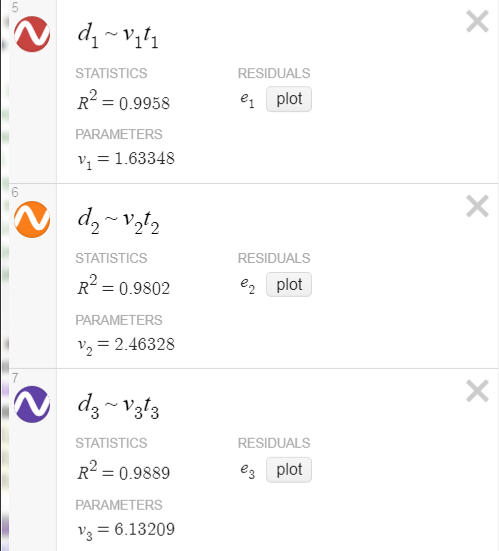## Data Display: Graphs

A graph is one of the first tools one uses when exploring new data. A graph will visually indicate whether a two variables are related and and what that relationship might be. Using the data, make a single graph with the multiple ball runs on a single graph.

The velocity of the ball is the slope of the line for that ball.

## Data Analysis and Results

In algebra the equation of a line with a zero y-intercept is written as y = mx where m is the slope. In physical science the slope is usually a rate of change. In this laboratory the slope is the speed of the ball. We use the letter v for velocity (speed in a direction) in formulas.

distance d = slope (speed v) × time t

d = v × t

This sometimes written simply as d = vt

Given any time t, we can calculate the distance d. Given any distance d, can solve for the time t.

### Discussion

Discuss the nature of the mathematical relationship between distance and time for a rolling ball. Discuss how different starting speeds affect the slope of the relationship. How is slope related to speed? Discuss whether the relationship appears to form the straight line predicted by the d = vt theory.

# 031 Acceleration and the Arc of a Sphere

Monday pre-activity: acceleration from rest for a moving object. Acceleration as a change in velocity with respect to time.

Acceleration is the change in velocity divided by the change in time. As an example, a RipStik was accelerated from rest to 1.34 m/s in 3.43 seconds, and then accelerated to 1.78 m/s in the next 2.59 seconds. The chart shows the time versus distance, velocity, and acceleration curves for the RipStik spring term 2010.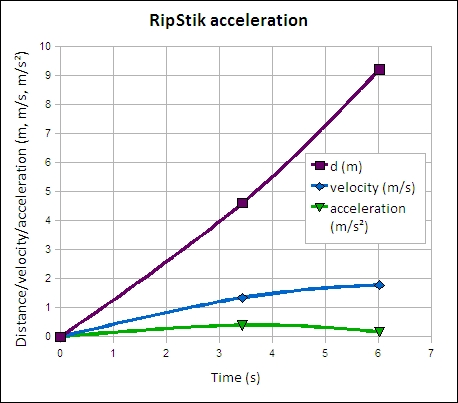location time (s)distance (m)velocity (m/s)mass (kg) momentum (kg m/s)force (kg m/s²)
pillar one 000 670 25.3
space
pillar two 3.494.61.32 88.31
space5.21
6.469.21.55 103.77
pillar three

## Arc of a Sphere

In this activity we will explore the shape of the path through the air made by a sphere. The path is a path in two-dimensional space. The graph will be a space versus space graph using the MKS system. The path reflects the influence of the acceleration of gravity on the sphere. The acceleration of gravity accelerates masses toward the center of the Earth at 980 centimeters per second squared. The result is a parabolic path.

We will be working outside to measure the arc of the sphere. The set-up and variables to be measured are seen in the following diagram.Where k is the height of the y-intercept above the x-axis
and r is the distance from the axis of symmetry to one x-intercept (root).

Task n Position title Student name x y
Right x-intercept 1 Ball underhand pitcher
2 Meter stick holder
3 Recorder
right mid arc height 4 Tape measure holder x y
5 Tape base holder
6 arc height observer
7 Data recorder
Vertex height k 8 Tape measure holder Same as 4 x y
9 Tape base holder Same as 5
10Vertex position observer
11Data recorder
left mid arc height12Tape measure holderxy
13Tape base holder
14arc height observer
15Data recorder
Left x-intercept16Ball catcherxy
17Meter stick holder
18Recorder
x-intercept to x-intercept19Wheel rollerdistance = 2r
20Recorder

Another way to introduce the unit on acceleration is to accelerate a swizzle board such as a RipStik along a flat stretch of sidewalk marked off with chalk. A time verus distance graph will show that the velocity is increasing. As homework on Monday the time versus distance table and graph can be assigned. If using Desmos, have the students use t₁ and d₁ as in laboratory two. Ask them to run a regression equation d₁~vt₁ and see how well that fits. Ask them to then try d₁~½at₁² in Desmos. Which mathematical model fits the data better?

# 032 Laboratory Three: Accelerated Motion

## Questions

What is the nature of the relationship between the time and distance for a ball falling to the ground?
If the velocity is changing at a constant rate, then there is a constant acceleration which would be called the acceleration of gravity. What is the value of the acceleration of gravity g?

### Introduction

This laboratory explores the relationship between time and distance for an object moving at a constant acceleration. In this situation the velocity is changing.

Instructional note. This laboratory builds on two earlier activities that during a regular school usually occur on Monday and Wednesday respectively. The first activity was a plot of time versus distance for a RipStik accelerating from rest. Based on the RipStik activity, there was a non-linear relationship (curved line) between time and distance for the accelerating RipStik. In the second activity, the RipStik is swizzled into an upslope crossing a distance equal zero line at the bottom of the slope. The RipStik decelerates until at a minimal speed the RipStik is turned around and then accelerated back across the starting line. This yields an inverted parabola which can be modeled in Desmos using d₁=½at₁²+v₀t₁ The following lab reproduces the arc of Monday but for a falling ball.

### Goals

• Determine the acceleration of gravity g
• Explore the nature of the mathematical relationship between time and distance for a falling object

### Theory

Existing gravitational theory asserts that the distance an object falls when dropped is given by the mathematical equation:

$\text{distance}=\frac{1}{2}\text{gravity}×{\text{time}}^{2}$

or

$d=\frac{1}{2}g{t}^{2}$

The theory predicts that graph of time versus distance should result in the half-curve of the start of a quadratic parabola as seen in graph 3.1.

The graph suggests that time and distance are not related linearly. That is, twice as much fall time results NOT in twice as much distance fallen, but in MORE THAN twice as much distance fallen.

Proving the hypothesis that a time versus distance graph is actually a parabola is difficult and generally requires calculus. Rather than prove that the curve must be parabolic, the laboratory will seek to show that the curve is well fitted by a parabolic model.

## Procedure

Laboratory teams will drop a ball timing the fall time for the ball.

Teams of three to four students will be formed composed of the following roles to facilitate measurements:

• Ball dropper and timer
• Meter stick holders (2)
• Recorder
1. Start by dropping and timing the ball fall time from one meter above the ground. Drop the ball five times, use the median time to improve accuracy.
2. Move up by one meter and drop the ball from two meters. Again, use the median time for five drops to ensure timing accuracy.
3. Move up by another meter and drop the ball again. Continue going up a meter until you reach five meters. Note that four meters and five meters will require a two story building with a balcony.

Notes

• The team member who drops the ball also must be the timer. Only the person dropping can start the stopwatch precisely enough to get accurate time measurements. Practice first using the stopwatch. The theory and known acceleration of gravity suggest a time of around 0.45 seconds for a drop of 1 m.
• The timer/dropper should watch the floor, not the ball, when dropping the ball and timing.
• The stopwatch is measuring 100ths of a second. 00 00 45 is actually 0.45 seconds. Note the decimal point!
• In Palikir three meter drops are not done because of ceiling fans.

Scratch table for jotting times in the laboratory.

1 m2 m3 m4 m5 m

The median times versus the drop height will be recorded into the table below and then plotted on an xy scattergraph, with the time in seconds on the horizontal x axis and the drop height in meters on the vertical y axis.

### Data table

t₁d₁
00
1
2
3
4
5

t₁ is the time in seconds
d₁ is the distance in meters

* After completing the data up to two meters inside the classroom, groups will work with the instructor to attempt to gather data at four and five meters using a balcony.

## Data graph

If working in Desmos, the graph will appear as data is entered into the table. If using other graphing software, follow the instructions of the instructor.

## Data analysis

Use the Desmos regression d₁~0.5gt₁^2 to obtain the experimental acceleration of gravity g. Note that using the cursor and the Desmos keyboard, the formula can be entered as d₁~½gt₁².

On the graph the rise is meters and the run is seconds². Slope is rise over run. Therefore the units of slope and of the acceleration of gravity are m/s².

### Raw error

The published value for the acceleration of gravity is close to 9.8 m/s². Subtract 9.8 m/s from the experimental acceleration of gravity g to obtain the raw error. In physical science 9.8 m/s² is used. The actual value of acceleration of gravity at the location of Pohnpei, 7° North 158° East, is 9.78543 m/s².

### Percentage error

The percentage error is the raw error calculated above divided by the published value of the acceleration of gravity g, expressed as a percentage.

$=\frac{\left(\text{your experimental acceleration of gravity g}-9.8\right)}{9.8}$

Report the experimental and published acceleration of gravity along with the raw error and percentage error in the analysis section of the laboratory report.

## Discussion

Discuss the nature of the mathematical relationship between time and distance for a falling object. Discuss whether the graph is reasonably close to a parabola. Report the experimental acceleration of gravity g based on the slope from the graph. Compare your result to the theoretically correct value of 9.8 m/s² Discuss any problems you encountered in this laboratory including those that may have contributed to uncertainty in your measurements.

Note that an percentage error of less than 5% is excellent agreement in this laboratory. An error less than 10% is good agreement. And an error less than 20% is still moderate agreement with the published value for this particular laboratory.

# 041 Momentum and energy

## Momentum

While momentum is mathematically defined in physical science as the mass of an object multiplied by the velocity of the object, momentum can be thought of as being the tendency of an object in motion to remain in motion. An object at rest will tend to remain at rest.

## Energy

Energy in this section refers to two different types of energy: gravitational potential energy and kinetic energy. Gravitational potential energy is the energy of position. Kinetic energy is the energy of motion.

### Momentum investigation questions

• When one marble collides with a line of five marbles, what happens?
• When two marbles collides with a line of five marbles, what happens?
• For how many inbound marbles does a pattern continue?
• When the inbound marbles are rolled in slowly, what happens?
• When the inbound marbles are rolled in quickly, what happens?

Sir Isaac Newton wrote in a letter to the Jesuit scientist Ignace Gaston Pardies:

For the best and safest method of philosophising seems to be, first to enquire diligently into properties of things, and to establish those properties by experiments and then to proceed more slowly to hypotheses for the explanation of them. For hypotheses should be employed only in explaining the properties of things, but not assumed in determining them; unless so far as they may furnish experiments.

Letter to the French Jesuit, Gaston Pardies. Translation from the original Latin, as in Richard S. Westfall, Never at Rest: a Biography of Isaac Newton (1983), page 242. Direct source: The Renaissance Mathematicus.

Newton started with diligent inquiry using experiments to establish properties and then used those properties to build hypotheses. The Greek philosophers, in contrast, often started with a hypothesis and rarely did experiments. In this exploration you are starting with inquiry and attempting to move towards a hypothesis.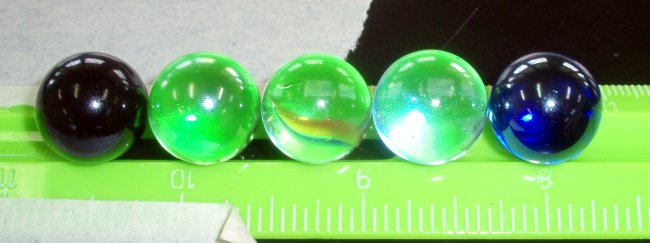## Post-exploration considerations

This exploration was of conservation of momentum in a collision of marbles. Conservation means "stays the same." In a momentum conserving collision we mean that the momentum after an event is the same as the momentum before an event. For this exploration the "event" was a collision between sets of marbles.

Momentum is the mass multiplied by the velocity. The lowercase Greek letter rho ρ is used for momentum, m is used for mass, and v is used for velocity (speed). The lower case Greek letter ρ looks like the English letter "p" but is the lowercase letter "r" in Greek. We can see this "p" shape in the English capital letter R. I have never seen an explanation of why density uses the letter ρ, but "d" was already in use for distance.

$\stackrel{⇀}{\rho }=m\stackrel{⇀}{v}$

The momentum ρ is defined as the mass multiplied by the velocity (speed). Momentum takes into account both the mass and the velocity. If "mass in" equals "mass out" and "speed in" equals "speed out," then the product of mass times speed in should also be equal to mass times speed out. That is, "momentum in" should be equal to "momentum out." This is called conservation of momentum.

Both momentum and velocity have directions associated with them, both are vector quantities. This means they are usually written with an arrow on top of the symbol for them. Marbles have a mass, their velocity is a speed in a particular direction. The tracks keep the marbles moving in the same single direction. In the world of science this is a one-dimensional model and keeps the mathematics simpler.

$\begin{array}{c}{\stackrel{⇀}{\rho }}_{\text{in}}={\stackrel{⇀}{\rho }}_{\text{out}}\\ {\text{mass}}_{\text{in}}×{\text{velocity}}_{\text{in}}={\text{mass}}_{\text{out}}×{\text{velocity}}_{\text{after}}\end{array}$

Written without the vector notation and subscripts, the momentum is ρ = mv where m is the mass and v is the velocity.

# 042 Laboratory Four

Laboratory four explores the relationship between the gravitational potential potential energy and kinetic energy. Gravitational potential energy is energy due to an elevated position. Kinetic energy is the energy an object has due to motion. When a marble rolls down a slope the gravitational potential energy is converted to kinetic energy. The same happens when a car rolls down a hill.

Questions

• How is the velocity of a marble at the bottom of a slope related to the height from which the marble was released?
• Is the relationship linear or non-linear?
• What mathematical rule, what equation, is the marble obeying?

Materials

• banana leaf
• marble
• ruler
• stopwatch app
• string
• scissors
• tape

Procedure

1. Measure the vertical height from which the marble is released.
2. Time the marble over a fixed distance on the flat surface at the bottom of the slope.
3. Repeat the timing measurement three times and use the median time.
4. Calculate the velocity v of the marble by dividing the fixed distance by the median time.
5. Record the height h1 and the velocity v1
6. Repeat this procedure starting at a height of zero and then increasing the height for five to ten measurements

Table

If using Desmos, graph the height h1 against the velocity v1.

h1v1

Caption: h1 is the height in centimeters. v1 is the velocity in centimeters per second.

Note that the table in Desmos will have six to ten rows, the above is just an illustration of the layout.

Graph

Make a graph of the data. Does the data form a straight line? Does the data form a smooth curve? Does the shape look familiar? The shape of a curve sometimes tells us the nature of the mathematical rule that the system is obeying.

Instructional note for instructors: Note that the graph is not a time versus space graph. The slope of a time versus distance graph was the velocity. This graph will be a height (space) versus velocity (space divided by time) graph. The slope is NOT the velocity.

In experimental physics we sometimes seek help from theoretical physicists who work with the mathematics and guide us in finding equations. If the data agrees with the equation, then that provides support for the mathematical result that the theoretical physicists have calculated. The following is an exploration of the theory, a theory that will lead to an equation.

## Gravitational Potential energy

Gravitational potential energy is energy contained in an object due to its position or composition. Objects at rest a height h above a surface have gravitational potential energy due to their position. Gravitational potential energy is equal to the mass multiplied by the acceleration of gravity g multiplied by the height.

Gravitational potential energy = mass × gravity × height

Note that the acceleration of gravity g is 980 cm/s². Although on Pohnpei the acceleration of gravity is 979 cm/s², that value is often rounded off to 980 cm/s², a value closer to the global average of 980.7 cm/s².

The "potential" part of the phrase refers to the "potential" for the energy to be converted to other forms of energy. In this activity the gravitational potential energy will be converted to kinetic energy. Gravitational potential energy is not the only type of potential energy, there are other forms of potential energy. Springs and rubber bands can store potential energy, as can bonds between atoms.

Letters in physical science can be very confusing. The g above is being used as a variable. In physical science g as a variable means the acceleration of gravity. The letter "g" is also used as unit. The letter g as a unit means "grams." Grams is a measure of the mass m. A regular marble has a mass of about five grams, often written as 5 g. Letters in physical science are often used with different meanings as variable and units. Only the context can tell you the meaning of a letter.

## Kinetic energy

Kinetic energy is the energy of motion, the energy that an object with a mass m has as a result of moving at a velocity v. Kinetic energy is actually the integration of the momentum with respect to velocity, but that is mathematics that is beyond the scope of this course. The kinetic energy KE can be calculated from the equation ½mv²

## Conservation of energy

The Conservation of energy states that energy can neither be created nor destroyed. Energy can only be converted between different types of energy. The conservation of energy suggests that the gravitational potential energy of the marble on the slope should be equal to the kinetic energy of the marble at the bottom of the slope.

There is one complication in the following calculation: at the bottom of the slope the marble has TWO kinds of kinetic energy. Have you ever spun a coin on one edge? The coin keeps spinning. The spinning coin has rotational kinetic energy. There is also rotational momentum. Thus the following includes consideration of both the linear kinetic energy of the marble in motion and the rotational energy of the marble because the marble is also spinning as the marble rolls.

Instructional note for instructors: What follows is the theory. The key point here is not that students will derive these equations, but to show how the mathematical equation is derived. The students are not trying to construct the theory.

The conservation of energy can be expressed mathematically as:

gravitational potential energy at start = kinetic energy at end

Remember that there are TWO forms of kinetic energy to account for in this experiment: linear kinetic energy and rotational kinetic energy. Therefore:
GPE = KE + RE.

Gravitational potential energy = mass m × acceleration of gravity g × height h.

Linear kinetic energy (KE) is the energy that an object moving across a surface. Linear kinetic energy is equal to:

$\mathrm{KE}=\frac{1}{2}m{\stackrel{⇀}{v}}^{2}$

where m is mass and v is the velocity.

Rotational kinetic energy (RE) is the energy of a rotating object. Rotating means spinning. As the marble rolls the marble spins. Rotational kinetic energy is equal to:

$\mathrm{RE}=\frac{1}{2}I{\stackrel{⇀}{\omega }}^{2}$

...where I is the rotational inertia of the spinning object.

In this activity the linear velocity (speed) of the marble, v, will be measured. The rotational inertia is related to mass and velocity by the following relationships for a marble with a mass m and a radius r.

$I=\frac{2}{5}m{r}^{2}\phantom{\rule[-0ex]{2em}{0ex}}\omega =\frac{v}{r}$

The following is a sketch of simplifying GPE = KE + RE.

$\begin{array}{c}mgh=\frac{1}{2}m{\stackrel{⇀}{v}}^{2}+\frac{1}{2}I{\stackrel{⇀}{\omega }}^{2}\\ mgh=\frac{1}{2}m{\stackrel{⇀}{v}}^{2}+\frac{1}{2}\left(\frac{2}{5}m{r}^{2}\right){\left(\frac{\stackrel{⇀}{v}}{r}\right)}^{2}\\ gh=\frac{1}{2}{\stackrel{⇀}{v}}^{2}+\left(\frac{2}{10}{r}^{2}\right)\left(\frac{{\stackrel{⇀}{v}}^{2}}{{r}^{2}}\right)\\ gh=\frac{{\stackrel{⇀}{v}}^{2}}{2}+\frac{{\stackrel{⇀}{v}}^{2}}{5}\\ gh=\frac{{5\stackrel{⇀}{v}}^{2}}{10}+\frac{{2\stackrel{⇀}{v}}^{2}}{10}\\ gh=\frac{{7\stackrel{⇀}{v}}^{2}}{10}\\ {\stackrel{⇀}{v}}^{2}=\frac{10gh}{7}\\ \stackrel{⇀}{v}=\sqrt{\frac{10gh}{7}}\\ \stackrel{⇀}{v}=\sqrt{1400h}\end{array}$

In the above expression 1400 is in the units of acceleration: cm/s². After substitution and simplification the relationship between the velocity and the height is given by the following equation.

$\stackrel{⇀}{v}=37.4\sqrt{h}$

Note that the mass and radius (size) of the marble is irrelevant to the speed. Note too that the speed is proportional to the square root of the height. This is not a direct relationship, not a linear relationship.

The above suggests that we can sse the Desmos regression v1~k√h1 as a mathematical model where k is constant that Desmos will optimize. The theory predicts k should be 37.4. Note that the square root symbol √ can be entered using the Desmos keyboard or by typing SQRT when entering the regression in Desmos: v1~kSQRTh1

Discussion and conclusions

Does equation suggested by the theoretical physicists have a shape that agrees with your data? Why or why not? What factors might be affecting the system? A good experimenter thinks about and identifies the factors that might have introduced errors into their measurements.

## Post-script

The key idea is that mathematical models form shapes on graphs.
The shape of the line tells you the form of the equation.
And hypotheses in physical science are often these same equations.
When a hypothesis is confirmed beyond reasonable doubt, it is called a law.

A line means the system is represented by a linear mathematical equation.
A parabola means the system is represented by a quadratic equation.
A parabola on its "side" (seen above) is a square root equation.

The experimental data forms a shape that suggests a square root relationship.
This then provides support to the theory.
Note that two lines are not equivalent, just of the same shape.
The actual data may produce a lower speed than predicted by theory due to friction.

In the centimeter-gram-second system energy is in units called ergs. One erg is a very small amount of energy. An erg is an ant push-up.

# 042 Laboratory Four Alternative: Momentum conservation on a banana leaf

This variant was developed in 2014. Large percentage errors in timing and friction with the banana leaf plagued the design. Students became bogged down in dealing with a difficult system to work with and lost sight of the larger concepts that the laboratory was meant to convey. This variant has not seen use since 2014.

Introduction

This laboratory explores the concepts of momentum and conservation of momentum.

Questions

• What does momentum mean?
• What does conservation of momentum mean?
• Can we show that momentum is conserved in simple systems?

Existing theory asserts that momentum is conserved. At first you will qualitatively explore the conservation of momentum. Then you will make some quantitative measurements of momentum for marbles inbound and outbound from a collision.

In physics:

• Momentum is the mass (grams) multiplied by the velocity (cm/s). The letter P is used for momentum, m is used for mass, and v is used for velocity (speed).
$\stackrel{⇀}{P}=m\stackrel{⇀}{v}$
The arrows above the P (momentum) and the v (velocity) mean that these are quantities with an amount and a direction. If the amount of velocity changes, then the momentum changes. If the direction changes, then the momentum also changes. Both affect the momentum. In this experiment the direction will not change, so the arrows will be dropped from the formulas.
• Conservation means "stays the same." Usually this means, "the momentum after an event is the same as the momentum before an event." For this lab the "event" is a collision between marbles.

Equipment

• marbles
• rulers meter sticks
• stopwatch
• banana leaves set up as ramps

Qualitative explorations

Five marbles sit touching each other in the middle of a banana leaf marble track. One or more marbles are rolled from one end of the track and allowed to collide with the five stationary marbles. The students observe how many marbles are kicked out from the line of five marbles.

Quantitative explorations of a single marble inbound

Said "mathematically," the momentum before is equal to the sum of the momentums after is written:

Pbefore = Pafter
minbound × vbefore = moutbound × vafter
where m is the mass of the marble, v is the speed of the marble.

1. Find the masses of both the inbound and outbound marbles.
2. Measure the distance from the shoot to the set of five marbles.
3. Measure a distance on the outbound side of the five marbles.
4. Shoot a marble into five marbles on the marble track. Have a single timer use a chronograph with lap time capabilities. The timer will start with the release of the shooting of the inbound marble, press the lap button when the inbound marble collides with the five marbles, and then press the stop button when the outbound marble reaches the end of the pre-measured distance.
5. Repeat the procedure for different inbound marble speeds including an inbound speed of zero.
6. After obtaining at least five pairs of momentum in and momentum out, prepare a graph of the data.

Data tables

mass inbound marble × velocity inbound = momentum in [x] mass outbound marble × velocity out = momentum out [y]
mass m (g) × distance (cm) ÷ time (s) = momentum (g cm/s) mass (g) × distance (cm) ÷ time (s) = momentum (g cm/s)
× ÷ = × ÷ =
× ÷ = × ÷ =
× ÷ = × ÷ =
× ÷ = × ÷ =
× ÷ = × ÷ =

Transfer the momentum results to the following table. The following is the data to be graphed.

momentum in p (g cm/s) [x]momentum out p (g cm/s) [y]
00

Graph

Make an xy scattergraph with the momentum in on the x-axis, momentum out on the y-axis

Analysis and brief discussion [a]

• Was momentum conserved?
• What was the slope?
• Based on your slope, was momentum lost or gained?

Instructional note: In practice the timing is prone to large errors. Friction complicates efforts to accurately record the outbound marble speed. The students become "bogged down" in the complexity of getting accurate times and lose sight of the larger picture of the physics happening. The more qualitative approach followed by presentations appears to yield a better insight in the concept of the conservation of momentum. In this alternate version the instructor is left in the position of arguing that momentum would be conserved but for friction and spin up/spin down loss of rotational energy/momentum.

Notes from the field for instructors: In this laboratory we explore conservation of linear momentum. Another momentum that is conserved is angular momentum. Angular momentum is the momentum of spinning. Spinning objects tend to continue to spin. Objects that are not spinning tend to remain at rest – to not spin. Think of a child's toy top. In the experiments above we considered only linear momentum, but the marbles are spinning as they move on the track. In these experiments spinning marbles hit non-spinning marbles. The inbound marbles lose speed and thus spin, the outbound marbles go from not spinning (sitting still on the track) to spinning very quickly. These changes in spin momentum are likely to be related to why linear momentum is consistently "lost" in these collisions.

Where linear momentum is p = mv, the angular momentum L = Iω where I = 0.4mr² and ω = v/r. Thus the angular momentum of a marble is L = 0.4mrv. One cannot just add all the momentums and hope for the best: the units are different. Ultimately one has to retreat to an energy position noting that the potential energy must appear as both linear and rotational kinetic energy in the marbles post-collision, along with losses to friction, sound, and any heat produced in the collision.

The thought occurred as to what to try to reduce the impact of external torque exerted by the track. One idea was to lubricate the ruler track with some form of greaseless lubricant such as WD-40®.

WD40 was tried. The first complication is the tape no longer holds the tracks in place. This problem proved rather insurmountable. In addition, WD40 wound up everywhere - on hands, table tops, soaked into paper that slid into the WD40. Would need a greaseless lubricant. Even then, the loss of taping ability would remain fatal.

Why not simply use pucks on an air table? Two key reasons. The puck and air table are unfamiliar to students - this raises the probability that the students will, in their own minds, see the whole thing as magic. Another mysterious thing in the modern world. Second, the lab should be as reproducible as possible by any teacher in the nation. The qualitative introduction to the lab requires nothing more than what an instructor on an atoll might be able to get their hands on.

The group work adds two less obtainable items - a stop watch and a mass balance. If a mass balance is not available, then simply treat the duck marbles as being 5 grams each. Some digital watches include a stopwatch or chronograph function. Cell phones also often have a stopwatch function built in.

Marbles on a track are very complex!

# 051 Force, Momentum, and Newton's laws

## Newton's first law: When the momentum is a constant including zero

$\stackrel{⇀}{p}=\text{constant}$

Newton's first law is a logical extension of the concepts of the conservation of momentum. Newton's first law simply says that the momentum remains the same. The variable used for momentum is p. Newton's first law is usually applied to single objects where the mass does not change. In this case the velocity of the object stays the same. If the object is at rest, then the object remains at rest. If the object is moving, the the object continues to move. The extension is that the law notes that the motion remains constant provided no external forces act on the object. As Newton said:

Every body persists in its state of rest or of uniform motion in a straight line unless it is compelled to change that state by unbalanced forces impressed on it. - Isaac Newton, Philosophaei Naturalis Principia Mathematica

The tendency of an object at rest to remain at rest, or if in motion to stay in motion, is also referred to as inertia. Inertia can be thought of as the natural resistance an object has to changing its state of motion.

Note that a single mathematical expression p = constant includes both rest (constant = 0) and motion (constant ≠ 0). This "symmetry" is more apparent when one is in constant, unaccelerated motion alongside a moving object. When I run next to a car moving at the same speed as I am running, the car appears motionless relative to me. I would say the car has a momentum of zero. A child sitting in a nahs would say both the car and I have a non-zero momentum.

The somewhat amazing aspect of momentum is that in collisions such as the marble collisions, all observers would say momentum is conserved. Both those sitting at the table and those moving with the speed of the rolling marble. The later observer would say the line of marbles moved backward into the one marble, collided, and "left one behind" stationary relative to the moving observer. If your head hurts a little thinking about this, do not worry, this is normal!

## Newton's second law: When the momentum is a changing

$∆\stackrel{⇀}{p}=\text{constant}$

Note the ∆, this means "the change in." There was no change in momentum in the first law. Now there is a change, a change at a constant rate.

Newton's second law builds on the first law. When the momentum changes with respect to time at a constant rate, this rate is called a force. What force is at a basic, fundamental level and how a force comes into existence remains difficult to characterize. Quantum field theory invokes particles to explain force, but that does not help us mere mortals who do not understand quantum field theory understand what a force is at a basic level.

In part one of laboratory four when one marble was rolled into the chain of marbles the force of the impact of the rolling marble was transmitted down the line of marbles to the last marble which then carried the original momentum away from the collision. Just as the "one-in, one out," "two-in, two-out," and "three-in, three out" was in some way mysterious, so too are forces.

$\text{Force}=\frac{∆\stackrel{⇀}{p}}{∆t}$

Newton himself thought of force as arising out of a change in momentum. Newton used the term quantity of motion for what we now call the momentum p. In Newton's words:

The net force acting on a body is equal to the rate at which the body's quantity of motion is changing.

As was the case with Newton's first law, the mass is often considered to remain the same. Considering mass to be constant and remembering that the momentum p is equal to the mass times the velocity allows for rewriting the second law in the following way:

$\text{Force}=\frac{∆\stackrel{⇀}{p}}{∆t}=\frac{∆\left(mv\right)}{∆t}=m\frac{∆v}{∆t}=ma$

Where m is the mass of the object, v the velocity of the object, and a the acceleration of the object.

If a force acts on an object, the object will accelerate. In laboratory three the force of gravity acted on the falling ball. The ball fell faster and faster as it fell. The rate of the increase in speed was the acceleration.

## Newton's third law: Force and counter-force

As noted above, force is a complex concept. For an object that is free to move, force can cause the object to move and to accelerate. For an object that is not free to move in the direction of the force, the force can still act on the object even though the object is not moving. The study of the application of force to fixed, non-moving structures is called statics.

Forces act on steel beams, bridge spans, building structures, tires, springs, and many other structures. Those forces may include the force of gravity on a bridge, the force of the wind on a building, or the force of the friction with the road on a tire. In each of these cases a counterforce must counter-act the first force or the object will move. While a moving tire may be desirable, a collapsing bridge or a building falling over are not desirable outcomes.

If I lean on the wall, I exert a force on the wall. If the wall did not push back, then I would fall. Imagine a wall made of water, I would simply fall into the wall of water. Water does not push back.

${\text{Force}}_{\text{object A on object B}}=-{\text{Force}}_{\text{object B on object A}}$

From the above comes the concept of force pairs. For every force that does not produce an acceleration, there is an equal and opposite force acting. This is sometimes said, "For every action there is an equal and opposite reaction." That is not entirely accurate, better to say, "For every force there is an equal and opposite counter-force."

Newton's third law is central to the work done by architects and engineers who use statics to analyze the forces on designed structures to ensure the structures are strong and will not fail.

Instructional notes: Demonstrations have included carrying a ball while coasting on a swizzle board to illustrate frames of reference. When the weather is nice, the class can be wrapped up by a third law demonstration known as a yurt circle outside. The class stands in a large circle and then counts off. The circle must be even, the instructor joins if the class is an odd number. Everyone holds hands or locks wrists. On the count of three those with odd numbers lean in, those with even numbers lean out. Done slowly and carefully, the circle will balance, with everyone off-balance.

# 052 Laboratory Five: Force of friction

## Questions

• What factors contribute to friction: weight, surface area, or surface roughness?
• Which factor contributes the most?
• Which factor contributes the least?
• What is the nature of the relationships between the factors and the force of friction

## Equipment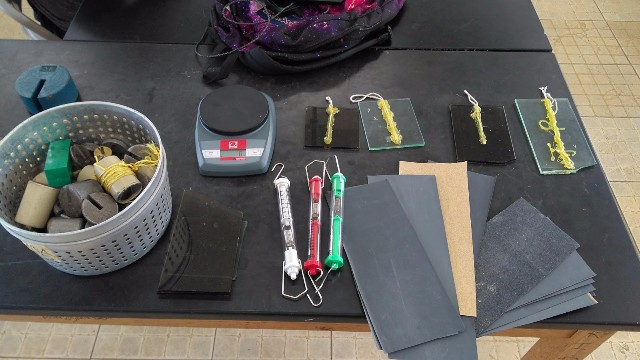• sleds of different sizes with strings
• spring scales
• mass balance
• weights
• sandpaper of various grits across as wide a range as possible.
• rulers

Instructional note: Broken louvers were used to create sleds for this laboratory in Micronesia. These must be handled with care. The upside is that there is no information on the sliding coefficient of glass on sandpaper on line. And the glass does not necessarily behave as might be expected based on information that is on line. The surface area controls for weight by the use of three sleds each consisting of two slabs of glass of different areas glued together with the yoke string in the middle. These three pairs are stacked and flipped in various orders to produce a constant weight sled with different surface areas.## Procedure

Each group works on their variable, measuring changes in their variable against the force of friction.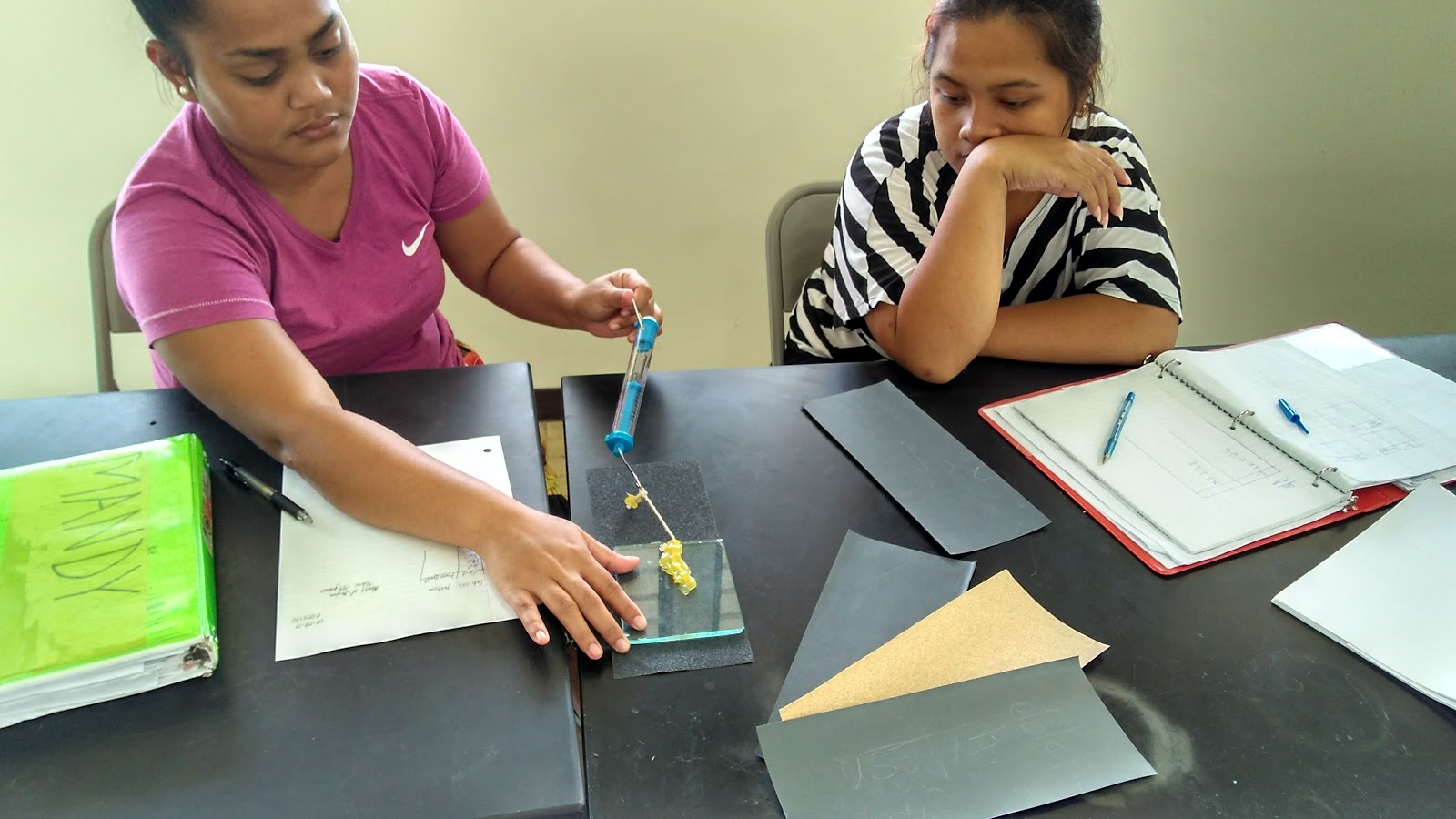Sandpaper grit grades of 60, 120, 360, 600, 1500

## Data Table

Each group works with one of the following tables. Tables may have more or less rows depending on the number of variations of a factor available. Tables can be entered directly into Desmos for those students with the ability to do so. This provides real time feedback on any emerging patterns.

### Weight

w1  f1

w1 is mass in grams
f1 is force in gram-force

### Surface area

s2  f2

s2 is surface area in square centimeters
f2 is force in gram-force

### Grit

g3  f3

g3 is sandpaper grit
f3 is force in gram-force

## Analysis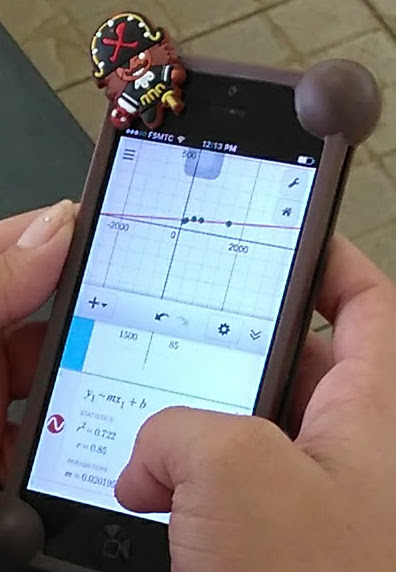Report whether the data is linear, non-linear, or random. If the data is linear, how good is the fit? What is the nature of the relationship: positive or negative? Does the relationship appear to be a strong or weak relationship? If Desmos is being used and the tables are labeled as above, then the regression equations for each would be: f1~m1w1+b1, f2~m2s2+b2, f3~m3g3+b3

The three slopes will be m1, m2, m3. Slopes near zero may indicate no relationship between the variables. The intercepts will be b1, b2, b3. If the slope is NOT close to zero and the intercept IS close to zero, then there may be a positive relationship for the data in this laboratory.

## Discussion

Discussion the results of your group and of the other groups in the laboratory report.

Instructional note: At the end of the laboratory the students can be asked to work on a brief presentation for the next day in the class. This could be the next lecture day of the class. This provides an opportunity for the teams of scientists to share their findings and explore which factors had the most effect, which had the least effect. In one class the instructor uses this opportunity to introduce the students to looking at the correlation r value displayed by Desmos.

# 055 Alternate Laboratory Five: Gram force extension of an elastic band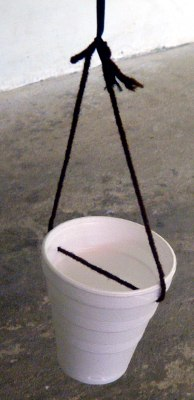## Question

Is there a linear relationship between the extension of an elastic band and force on the elastic band?

## Goal

Explore the mathematical relationship between force and the lengthening of an elastic band. Hooke's law for steel springs states that Force F = k × distance d where k is a constant called Hooke's constant. Materials that obey this relationship are called Hookean materials.

In this laboratory distances will be measured using centimeters. The force will be measured using grams of mass. When grams are used to express force F, the force F is called a gram-force. One gram-force is the force exerted by a one gram mass at rest on the surface of the planet earth.

## Equipment

• Styrofoam cups
• yarn
• elastic bands
• marbles

Instructional note: If one has no mass balances, simply consider the average mass of a duck marble to be five grams. The error that this will introduce will be small, essentially a linear transformation, and is tolerable in terms of the intent of this laboratory.

## Procedure

1. Form teams of three people. One will hold the meter stick, steady the cup, and read the downward displacement. The second will add marbles to the cup. The third will record data.
2. Attach a cup to a string comprised of lengths of yarn and a single length of elastic band.
3. Suspend the cup from the ceiling or a hook on the overhead track.
4. Adjust the height of the empty cup so that the cup is one meter above a table.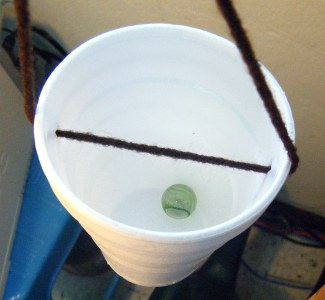5. Measure the mass of all thirty marbles.
6. Round to the nearest gram that is a multiple of three.
7. Divide by thirty. The result will be the mass of a marble rounded to the tenth of a gram. Use the result as the mass per marble for each and every marble. For example, if thirty marbles have a mass of 152.1 grams, then round to 153 grams. Divide by thirty and use 5.1 grams for each and every marble.
8. Add a marble to the cup.
9. Measure the extension distance d in centimeters.10. Repeat the above two steps until you have added 30 marbles. With each additional marble, measure the extension of the elastic band.

Note that the force is technically called a "gram-force" (gmf). Mass is not force, a gram-force is the force exerted by one gram as a result of the acceleration of gravity (980 cm/s²).

## Data tables [d] [t]

Total distance elastic has stretched (cm) [x] Force (total mass) of the marbles in the cup (gmf) [y]
00

## Graph [g]

Make an xy scattergraph of the data.

## Analysis [a]

1. Is the relationship linear?
2. Comment on the nature of the graph.
3. If not, are sections of the graph "reasonably linear"?
4. Report the slope and intercept.

## Conclusions [c]

Wrap up with a discussion appropriate to this laboratory. Discuss whether the system is "predictable," that is, if given a gram-force F could one make reliable predictions of distance d? Do some background research on Hooke's law of elasticity. Look it up on the Internet such as in the Wikipedia. In the conclusion, discuss whether the elastic band is a linear elastic ("Hookean") material.

# 056 Center of gravity and static forces

An interstitial activity

Where is the balancing point: what is the center of gravity?

Then into unreachable cup, stuck to the wall, straight back chair lift: human center of gravity

## Materials

• straight back chair
• tape measure
• cup
• pieces of cardboard cut in regular and irregular shapes
• stone or masses
• scissors
• nail
• pencil
• ruler
• meter stick
• string
• masses

## Pre-cognitive activities

• The unreachable cup
• Who can lift the chair?

### The unreachable cup

1. Place a cup out in the middle of the floor. Stand 30 cm away and pick it up without bending your knees.
2. Move to a wall. Place your heels against the wall. Have someone set the cup 30 cm in front of you. Pick up the cup without bending your knees.

What happened? Why?

### Who can lift the chair?

This one requires a demonstration by the instructor.

1. Measure two foot lengths from the wall by measuring off toe to heel, toe to heel. This works better with zoris or shoes on.
2. Standing two foot lengths away, face the wall. Bend over and with a straight back, place your head on the wall.
3. Have someone else hand you a straight back chair.
5. With the chair lifted, stand up.

Who could lift the chair? Who could not? Why could some lift the chair and some could not?

## Center of gravity: the balancing point

The above activities involve center of gravity. In this activity the center of gravity of an irregular piece of cardboard will be found as an illustration of the center of gravity as the balancing point.

1. Cut an irregular shape from cardboard.
2. Make a hole with a nail near any edge of the cardboard.
3. Secure the cardboard to cork board using a pin
4. Hang a light weight or rock from the pin.
5. Mark the plumb line on the cardboard.
6. Repeat for a hole roughly 90° around the edge.
7. The intersection of the lines is the center of gravity, the balance point.
8. Balance the cardboard on a finger at the balance point.

## Cantilevers

A cantilever is a beam that has one end extending into open space without support. With no weights attached, a meter stick balances in the middle.

The center of gravity for a meter stick with no weights attached is in the middle of the meter stick.

Attach a weight to one end and the fulcrum string has to move to rebalance the meter stick. The left end of the meter stick is termed a cantilever. The force of the weight is keeping the cantilever up. The new center of gravity, center of balance, is at the new location for the fulcrum string.

### Sample data

For the meter stick to balance, the mass of the meter stick to the left of the string must balance the mass to the right of the meter stick. Data was taken for a meter stick that had a mass of 193 g. Masses were used for weights, hence the force is called "gram-force." The table shows the length of the cantilever Lc and the mass m needed to balance that length of cantilever.

Data table

Lc (cm)m (g)
500
59.520
6240
64.560
66.580
68.7100
70.3120
72140
73.5160
74.5180
75.6200
76.5220
77.8240

The data above was generated in class. Graphing the data and performing a mathematical relationship analysis was assigned as homework.

### Analysis

A graph of the above data produces the following chart.

This system is not a direct variation nor a linear equation. The curve above is a section of a rational function.

For a 100 centimeter meter stick, Lc in centimeters, and m in grams hung from the 99 cm point, the governing equation is found by setting the torques equal to each other. ρ is the "linear density" of the meter stick in grams per centimeter of length.

$m=\frac{\rho \left(5000-100\mathrm{Lc}\right)}{\left(\mathrm{Lc}-99\right)}$

The equation contains information about system. As Lc approaches 99 from zero, the denominator becomes smaller and smaller. This represents the fulcrum string moving toward the mass. The decreasing denominator means that an increasing mass can be supported. As the denominator decreases toward zero, the mass that is balanced by the cantilever increases toward infinity. Of course in the real world the string would break when the total weight exceeded the strength of the string.

At the zero in the denominator the mass would be infinite. This is a vertical asymptote in the function.

Another interesting feature is that below 50 cm the mass goes negative. In other words a lifting force must be applied on the right arm if the cantilever is less than half the meter stick. This also makes sense: with the cantilever less than 50 cm, the right arm is longer and thus produces more force downward. A lifting force, effectively a negative weight, would have to be applied. Note that the equation is calculating force using gram-force.

For the particular meter sticks in use in class, ρ = 1.93. Substituting x for Lc and y for m yields an equation more familiar to an algebra student.

$y=\frac{\left(9645.5-192.91x\right)}{\left(x-99\right)}$

# 057 Air pressure and Bernoulli: Under pressure!

An outline of notes without explanation. To paraphrase what Fermat once scribbled in a margin, the explanations are elsewhere. A concept of discovery science is that the class thrashes out explanations. Better to not cover all of these and give the class some time to ponder the science.

These activity are interstitial activities that usually run on week where a holiday cancels the laboratory.

## Gear

• Albezia sticks
• balloon balance
• balloons
• blower/air cleaner
• folder
• glass
• newspaper
• paper
• ping pong ball
• string
• tape
• water jug

## Newton's third law

For any force a counterforce occurs: balloon rocket. Note that the surface of the balloon provides pressure that drives the air out the back of the primitive rocket. Use this as a lead in to the air pressure activities below.

## Pressure

### How many sheets to hold down a stick?

• Obtain some Albezia lebbeck sticks or other snappable wood.
• Place a stack of newspaper on the table.
• Ask the class how many sheets of newspaper are sufficient to hold down the stick so that it can be broken in half with a chop of the hand.
• Demonstrate that a single sheet is sufficient.
• Demonstrate what happens without the sheet. Wear safety glasses and ensure that no one is within a 45° angle down strike. The wood will likely launch up and out like a one way boomerang.
• Discuss. Do not simply explain. Discuss why. To the extent possible remain in a Socratic dialog mode.

Albezia, being round and rough, does not work as well as a thin flat pine yardstick. Increasing the surface area of the Albezia by using a forked stick or pre-halving the stick may help.

Pressure equals the force per unit area. For an equal application of force, a smaller area exerts a higher pressure due to the inverse relationship for the area.

$\text{pressure}=\frac{\text{force}}{\text{area}}$

Solving for the force indicates that larger areas produce more force for a constant pressure.

$\text{force}=\text{pressure}×\text{area}$

### Defying gravity with water: pressure is all around us

• Cut out a piece of manila folder or other suitable material.
• Fill a drinking glass with water.
• Place the paper over the open end of the glass.
• While holding the paper, invert the glass.
• Release the paper.
• Discuss. Do not simply explain. Discuss why. Note that this might only work in Kitti.

Occasionally a student will postulate that air in the glass would cause the demonstration to fail. Having some air in the glass does not cause the demonstration to fail. Demonstrate this as necessary. This works with really tall containers. For a bigger mental impact use a larger container.

### The arc of a water spout

• Find a container into which three holes can be placed. Bigger is better, more fun, and messier. A five gallon water container that has already developed a leak is useful. Ask at the local water company. Patch any hole that interferes with the demonstration.
• Put three holes into the side of the container vertically. If need be, cover the holes with tape. Keep a "tape tab" so the tape can be easily removed.
• Ask the class to sketch or otherwise describe the path the water will make.
• Fill with water.
• Remove the tape tabs.
• Discuss. Do not simply explain. Discuss why. What is the shape of the water arcs? How does pressure vary with depth?

### Balloon balance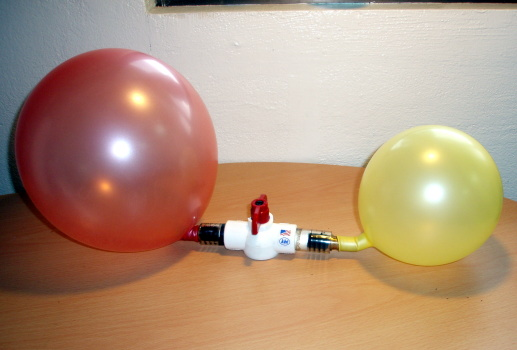• Connect two balloons via a valve. This may take the most prep. Current unit consists of a stop cock valve for 3/4 inch PVC pipe with two short lengths of clear plastic tubing that snug fits into the PVC valve. Two brass gas nozzles are fitted into the clear plastic pipes. Electrical tape is used to add necessary thickness to the brass gas valves to hold on the balloons. All parts were found at a local hardware store. In the past needle valves used for fish tank air systems have been used, lots of electrical tape is needed to build up the small tubing so as to keep a balloon attached.
• Inflate one balloon and attach to one side of the valve with the valve closed.
• Under inflate a second balloon and attach to the other side.
• Ask the class to predict what will happen when the valve is opened.
• Open the valve.
• Discuss. Do not simply explain. Discuss why.

### Others

• Petri dish with water stuck to the bottom of a mirror tile
• Plastic sandwich bag introverted in a jar.
• Plastic sandwich bag extroverted on a jar.
• Balloon inflated into a styrofoam cup.

Source: Tik L. Liem. Science Inquiry Second Edition.

## Bernoulli

### Why planes fly

• Cut out a long strip of paper.
• Blow.
• Discuss. Do not simply explain. Discuss why.

### Magic floating balloon

• Use a blower that blows up.
• Put a balloon in the air column.
• Discuss. Do not simply explain. Discuss why.

### Curve ball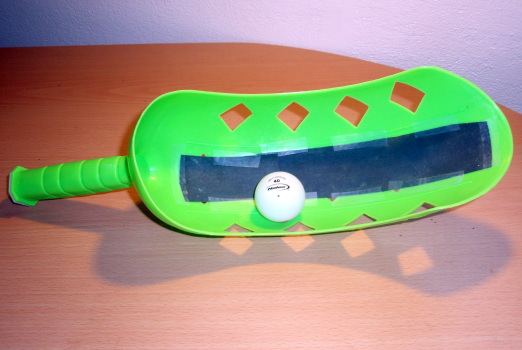• A curved plastic toy ball slinger.
• A ping pong ball.
• Throw. By now the students are used to ducking for cover. Best done outdoors!
• Discuss. Do not simply explain. Discuss why.

Later: two balloons hanging from a stick

• Hang two balloons on strings from a stick so they are a few inches apart.
• Blow between the balloons.
• What happens? Why?
• Discuss. Do not simply explain. Discuss why.

# 058 Pulley laboratory

Pulleys are simple machines that lift objects in a variety of ways. The simplest kind of pulley is a grooved wheel around which a rope is pulled. Pulleys can change the direction of an applied force. A combination of fixed and movable pulleys is a pulley system. This is called a block and tackle. A pulley system multiplies applied force to lift heavy objects. Pulley systems are commonly seen on construction sites. In this investigation, each group will use different pulley systems and determine the mechanical advantage of each.

Equipment: pulleys, lines, masses, spring scales

For your pulley system, make a sketch of the pulley(s) and lines. Record the number of lines supporting the load. Starting with a light load, record the force applied to lift the load. Add mass to the load, each time recording the applied force to lift the load. Graph the applied force versus the load. The slope of the trend line is the mechanical advantage for your system.

Force applied as measured by the spring scale (gmf) [x] Load (weight) lifted by the applied force (gmf) [y]

gmf is "gram-force." One gram-force is the force exerted by a one gram mass sitting on the surface of the earth. Using gram-force allows one to record data in "grams" of mass and "grams" of force on the spring scale.

The actual mechanical advantage for your pulley system is the slope of the trendline.

The ratio of the actual mechanical advantage to the ideal mechanical advantage is the efficiency of the pulley.

This report is a full write-up with an introduction, equipment list, procedure, table, labeled xy scattergraph, analysis, and a discussion of the results. The analysis should include the ideal mechanical advantage, the actual mechanical advantage, and the efficiency. The conclusion should include a discussion of results including a discussion of why the actual mechanical advantage differed from the ideal. Cite any sources used in your research on this topic.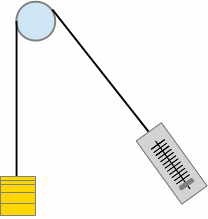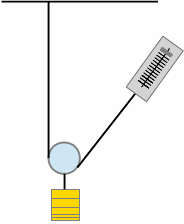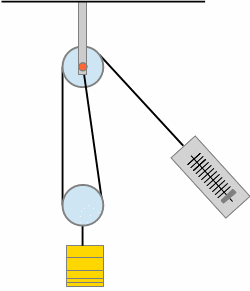# 061 Heat and Temperatures

## Heat energy and moving heat energy

Heat is another form of energy. Previously we worked with potential energy PE, the energy an object of mass mass m possesses for being a height h above a surface in gravitational field g. GPE = mgh. We also worked with kinetic energy, the energy of motion. Kinetic energy KE = 05mv².

There are three way heat energy is transfered (moved).

1. Radiation: heat carried as wave of light. Use a flat cardboard square covered with aluminum foil to reflect solar radiation. One can feel the heat of the sun reflected by the foil.
2. Convection: heat carried upward by rising fluids. Warm air rises. Warm water rises to the surface of the lagoon. Water vapor generated by a pot of boiling water rises. Convection is important in the fields of weather and oceanography. Convection in the atmosphere generates clouds. In the tropics, convection is almost the exclusive generator of low level clouds.
3. Conduction: the transfer of heat through a solid substance or between two substances.

Temperature is not the same thing as heat. Temperature is a measure of the kinetic energy within the molecules of a substance or fluid. Temperature is related to heat. Heat energy moves from places at a higher temperature to places at a lower temperature.

## Fire and ice: measuring temperatures

Water is boiled, water is melted. The temperatures of these events is measured in a demonstration of temperature and explanation of the Celsius system. Other temperatures demonstrated include that of the human body, room temperature, melting solidified coconut oil.

The following was done as a demonstration

• Melting ice water temperature:
• Melting "frozen" coconut oil temperature:
• Room temperature:
• Outdoor temperature:
• Human body temperature:
• Boiling water temperature:

# 062 Laboratory 06: If you cannot take the heat, get a non-conductor

## Questions

What materials are conductors of heat? What materials are insulators of heat? What materials are the best conductors of heat?

The basic set up: hot water in the large cup, room temperature water in the smaller cup.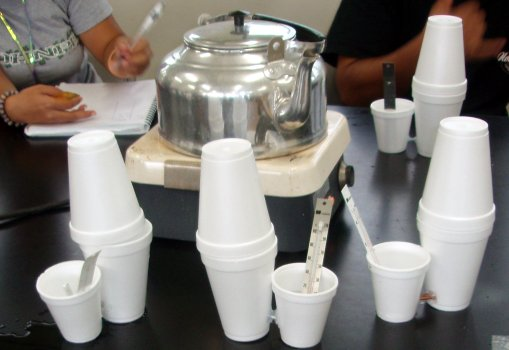The conductors are hardware store finds: sections of six gage solid copper electrical wire, a zinc wall board fastener, bolts, and other odd bits of materials.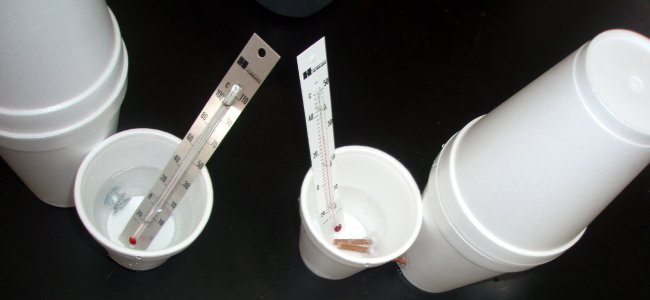Each group should measure one to two elements.

What metals conducted heat? Which conducted the most heat? Which conducted heat the fastest? How could we best represent the data using a chart?

Instructional note: Have the students work in teams of two on gathering data. Put all of the data on the board. Group pairs of teams to form four to six person groups to work on a chart for a presentation that includes the data gathered in the class. The chart should be able to show all of the materials tested by all the groups. The chart should also show the best and worst conductors. Give these larger discussion groups sufficient time to come up with a chart or graph concept. Have a spokesperson present the concept to the rest of the class.

## Group presentations

As a group you will present a chart to the class showing which elements conducted the charts. Your choice on chart type. The instructor may ask questions for clarification on your table and chart designs. There is no one right answer. You are a community of scientists: ask questions.

## Hand-in post-lab

Use a spreadsheet to produce and hand-in a chart that shows all of the materials and depicts the best and worst conductors.

## Alternative 062: Heat Conductivity with a focus on mathematical models

Have the students record the time in minutes t1versus the temperature in degrees Celsius T1. Plot the data until the temperature holds constant for multiple minutes.

Discuss with the student groups what mathematical models the group members thing might fit their data. Sketches of various possible mathematical models can be provided to the students. The data often produces an "ess" shaped curve that can be modeled using a logistic mathematical model. As the students have likely not studied the base of the natural logarithm e let along logistic curves, this will require a brief explanation. This provides a chance for the students to learn that data has driven models in the past. Logistic and their relatives the sigmoid curves arose in part out of studies of population growth with limited resources. The data drove the development of the mathematical models. The mathematics does not have to come first, the data can lead to a model.

The curve in the image is logistic mathematical model. Desmos distinguishes between lower case t (time) and upper case T (Temperature).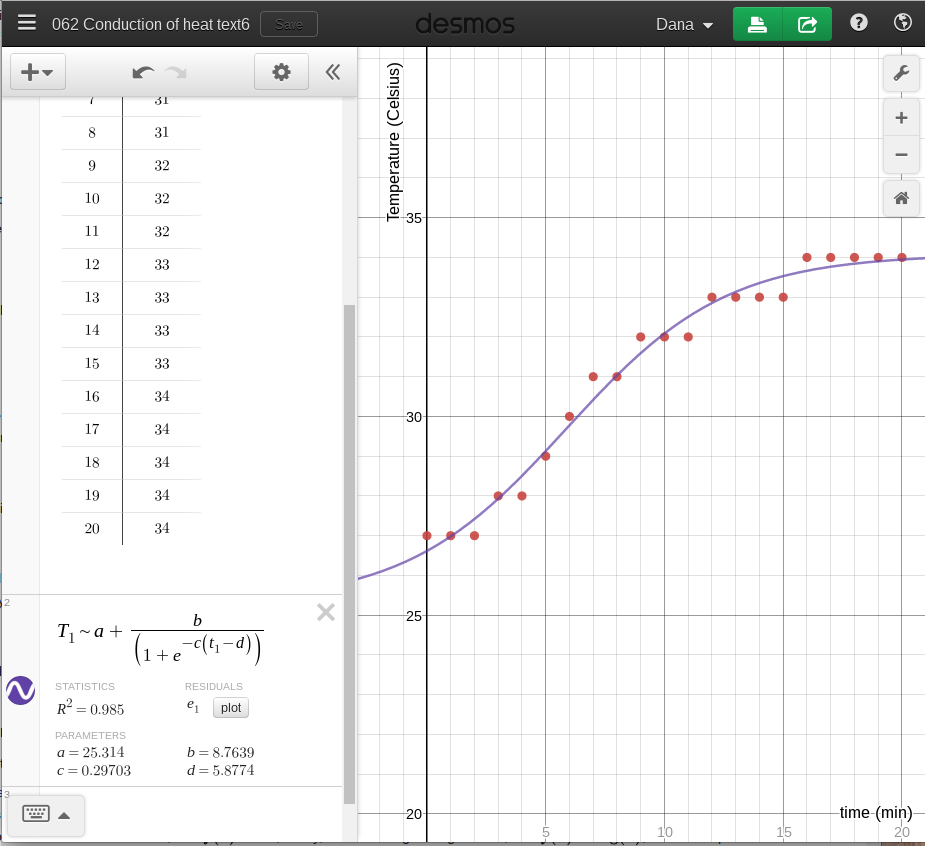The logistic mathematical model is entered as: ${T}_{1}~a+\frac{b}{\left(1+{e}^{-c\left({t}_{1}-d\right)}\right)}$

Note that the table header in Desmos uses t1 versus T1:The laboratory discussion can discuss what is happening physically according to the group's chose mathematical model.

## Alternative 062: Newton's law of cooling

Have the student pour boiling water into an uncovered cup. Record the temperature for up to an hour. The exploration is whether Newton's law of cooling well accounts for the cooling behavior. In the following Desmos formula x1 is the time in minutes, y1 is the temperature in Celsius.

${y}_{1}~\left({T}_{0}-{T}_{1}\right){e}^{-k{x}_{1}}+{T}_{1}$

Where T0 is the starting temperature and T1 is the ending temperature. Note that the model fits best if the formula is entered as seen and Desmos is permitted to regress T0, T1, and k. Discussion of results is enhanced by being able to display all of the cooling curves on large monitor. This can be achieved by the instructor entering data into a master Desmos file as the students gather the data.

While Newton's law does not strictly apply to convective cooling, the system is reasonably well modeled by the law.

# 071 Where in the world is the instructor?

Instructional note: Monday GPS orientation session so that Wednesday stays focused on latitude and longitude discovery learning and not technical issues with GPS operation.

In this "hide and seek" activity students work in teams to learn about latitude and longitude. The learning model is based on discovery learning. Each team will have a global positioning satellite (GPS) receiver. Students form their own teams based on the number of GPS units.

1. N 06° 54.650', E 158° 09.453'
2. N 06° 54.585', E 158° 09.339'
3. N 06° 54.552', E 158° 09.381'
4. N 06° 54.660', E 158° 09.589'
5. N 06° 54.663', E 158° 09.575'
6. N 06° 54.667', E 158° 09.558'
7. N 06° 54.661', E 158° 09.559'
8. N 06° 54.630', E 158° 09.330'
9. N 06° 54.640', E 158° 09.303'
10. N 06° 54.604', E 158° 09.308'

Garmin eTrex directions, light version. There are five buttons on the eTrex: up arrow (scroll or cycle) up through options, down arrow (scroll or cycle) down through options, enter, page, and power (pwr).

• power: Press and hold to power on the unit. Release when screen activates.
• page: change screen to a new screen.
• up/down arrow: change options displayed at the bottom of the bearing screen ("scroll" through the options)
• enter: display options for the displayed screen. Not necessary for today's activity.

Although the GPS receivers appear identical, some use an older operating system. With the older GPS units you will have to use the compass bearing screen and the down arrow to display the location screen. For the newer GPS units there is an additional screen after the compass bearing screen that can display the latitude and longitude. For all of the GPS units, the compass/bearing screen can display latitude and longitude information. If nothing else: study the directions, play with the buttons. Discover and learn!

If your batteries go dead, see the division administrative assistant for replacement batteries. If the unit does not turn on after holding the "power on" button, then try replacing the batteries. Remember: the unit will only work OUTSIDE.

This game has a 55 minute time limit: one class period. If the instructor is not found by the end of the period, then return the GPS units to the administrative assistant in the division of natural sciences and mathematics. In case you do not find the instructor, Thursday's laboratory is outdoors. Good hunting!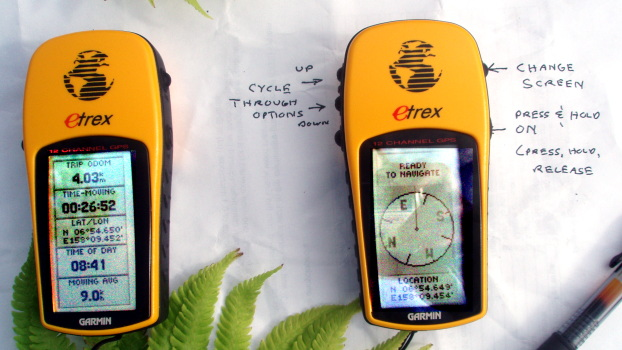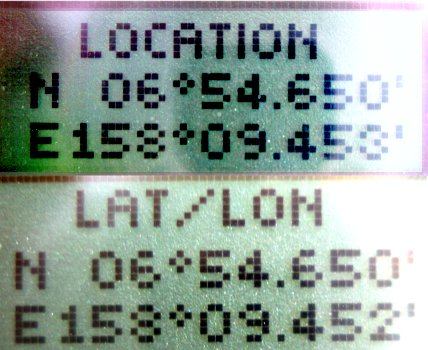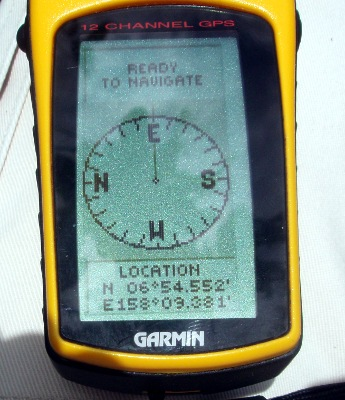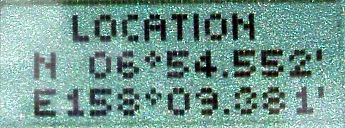# 072 Laboratory 07: Latitude, Longitude, and Metric Measures

## Questions

How many meters per arcminute latitude (or longitude)?
What is the conversion factor from metric meters to minutes of arc?
Given that the GPS units can measure differences of 0.001 arcminutes, what is the distance in meters for 0.001 arcminutes?
How close to a location can the GPS unit put a person?

## Purposes and goals

1. To find a mathematical relationship between metric system meters and arcminutes of longitude or latitude.
2. To continue to develop the concepts of latitude and longitude and their orientation on campus.
3. To continue to become familiar with GPS equipped units and technologies.

Along a line of latitude, the longitude values increase or decrease.
Along a line of longitude, the latitude values increase or decrease.

Latitude and longitude are measured in degrees and arcminutes. There are 60 arcminutes in one degree. There are 360 degrees in one circle. Note that arcminutes of latitude and longitude are effectively measures of distance not duration, of space not time.

On Earth the equator is 0 degrees latitude. The north pole is north 90 degrees, +90°. The south pole is south 90 degrees, -90°. The equator is half-way between the north and south pole. Pohnpei is located about six degrees north of the equator, almost seven degrees. On the Palikir campus, a global positioning satellite receiver (GPS) will display a latitude value close to N 6° 54.580'. The 54.580' digits are the minutes of arc, the apostrophe is the symbol for minutes. These are not minutes of time, but minutes of space. Arcminutes, also known as minutes of arc, measure an angle. On the Earth's surface, however, an arcminute can be expressed as a distance. Arcminutes are sometimes just called minutes, which can be very confusing!

On Earth the prime meridian is 0 degrees longitude. Pohnpei is located 158 degrees to the east of the prime meridian. The furthest east is 180 degrees. At 180 degrees east one is also 180 degrees west of the prime meridian. East longitude is positive, west longitude is negative. The GPS will display a longitude value near 158° 09.600' on the Palikir campus of the college.

In part one we will work outside. While outside we will use global positioning satellite (GPS) receivers to walk along either a line of latitude or a line of longitude. Lines of latitude run east - west. Lines of longitude run north - south. We will either walk along a line latitude heading due east, or walk along a line of longitude heading due north. Walking east along the line of latitude will cause the longitude value to increase. Walking north on a line longitude will cause the latitude to increase. Here in the FSM these directions, east or north, will keep the slope positive for the relationship between minutes of arc and meters. The other value should be held constant to remain on that line of latitude or longitude.

The latitude or longitude will be determined using a GPS unit. A surveyor's wheel will be used to measure of the distance in meters.

## Data gathering

Instructors note: If the surveyor's wheel being used is calibrated in feet, then every one hundred clicks is roughly 30 meters. 100 feet is 30.48 meters which is 1.6% too long. This level of error is an acceptable trade-off given the simplification of the metric data values. Depending on the start location, on the Palikir campus one can measure 120 to 150 meters along a line of latitude in the area north of the A and B classroom buildings. Other sites would have to make their own local decision on whether to measure along a line of latitude or longitude. A conversion table with conversions for 30, 60, 90 meters... can also be used to cope with non-metric measuring equipment.

Latitude/longitude value held constant:_________ ° ______.____________'
Starting longitude/latitude:_________ ° ______.____________'

In the following table record the change in arcminutes of latitude (or longitude). To do this, read the degrees and arcminutes shown on the GPS and subtract the start value recorded above. To keep the relationship positive in Micronesia, walk North or East. If doing a line of latitude on the Palikir campus, start at the main parking lot and walk East.

Subtracting the start value sets the y-intercept to zero and yields a direct relationship.

a1d1
00.0000
______.____________30
______.____________60
______.____________90
______.____________120
______.____________150
______.____________180
______.____________

a1: Change in distance (arcminutes)
d1: Change in distance (meters)

### Alternate data format

Some smart phones apps report latitude and longitude information from a built-in GPS in decimal degree format. If working with with decimal degrees the app may report values to a fifth decimal place, 1/100,000th of a degree. The data when graphed will yield the meters per degree, which could be multiplied by 360 to estimate the circumference at that latitude or longitude.

## Graph

Plot the arcminutes a1 versus the meters d1 using Desmos. Add a the linear regression d1~ma1

## Numeric Analysis

Report the line of latitude/longitude that was held constant, the line the students walked along. Report the starting longitude/latitude. The slope is the number of meters per arcminute. This represents the number of meters one has to go to travel one arc minute on the earth's surface. Report the slope with the units - the conversion factor has units meters/minute.

The analysis should include latitude which was held constant, starting longitude, ending longitude, and the slope which is the conversion factor for moving between arcminutes and meters.

## Discussion and conclusion considerations [c]

The meters per arcminute indicates the number of meters to move one minute of arc over the surface of the earth. The GPS measures minutes to three decimal places, one-one thousandth of a minute of arc. If the distance between two locations is smaller than one-one thousandth of a minute of arc, then the GPS cannot distinguish between the two places. How many meters must one move in order to change the minutes by 0.001'?

The answer to the question, "How many meters must one move in order to change the minutes by 0.001'?" is key to answering how close to a location can a GPS put one? How close can the coordinates given in the hide and seek exercise put one to the target? Discuss these questions and their answers.

If you can determine the correct value of meters per arcminute for the location at which the laboratory was done, that value can be used to estimate the percentage error between the experimentally measured value and the expected (published) value. The error can be reported and discussed.

In part two, power, Internet, and lab access permitting, the class will use Google Maps or Google Earth. Google Maps is accessible from any browser and can be commanded to display the distance walked in meters. Google Earth will also be used to show the equator and prime meridian. The location of 071 GPS hide and seek can also be displayed. Use of Google Earth as an educational tool for exploration and learning is also covered. Edutainment geolearning sites like GeoGuessr might also be presented.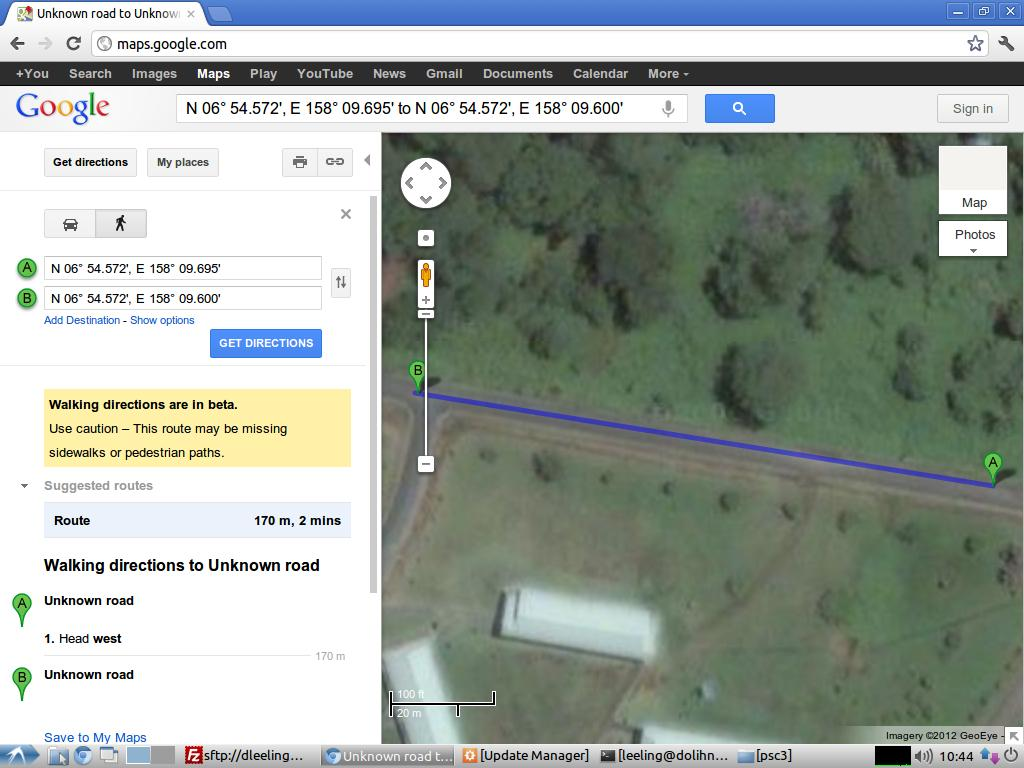the students should be encouraged to engage in open exploration.

If Google Earth is available, then Google Earth has a ruler feature that can measure in meters.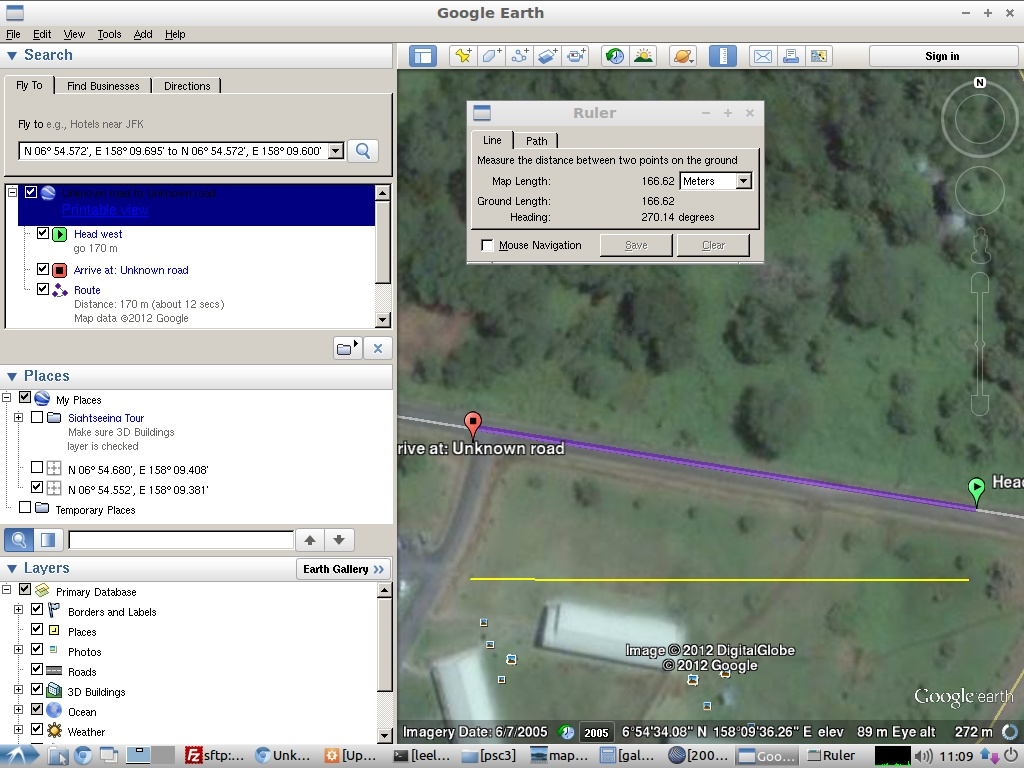# 081 Relative humidity and precipitation

The class starts off with a demonstration of calculating the relative humidity, heat index, and risk level for activity using a wet and dry bulb thermometer. Tables based in part on the heat index calculator.
Other weather calculators.

1. Look in the first column on the left. Find the row with the nearest dry bulb temperature to the measured dry bulb temperature.
2. Move to the right along the row until the wet bulb depression in the top row is matched.
3. Read the relative humidity provided by the table.

Relative humidity from dry bulb and wet bulb depression:

Web bulb depression in °C: Dry bulb minus the wet bulb Dry bulb T in °C 0.5 1.0 2.0 2.5 3.0 3.5 95% 90% 83% 79% 77% 72% 67% 60% 96% 91% 84% 80% 78% 74% 69% 62% 96% 91% 85% 81% 78% 75% 71% 65% 96% 93% 85% 82% 78% 75% 72% 65% 96% 93% 88% 84% 80% 76% 73% 66% 96% 93% 88% 84% 81% 76% 73% 67% 96% 93% 89% 85% 81% 78% 74% 68% 96% 93% 89% 85% 82% 79% 75% 69% 96% 93% 89% 86% 83% 80% 76% 71%
1. Look in the first row at the top. Find the column with the nearest dry bulb temperature to the measured dry bulb temperature.
2. Move down the column until the relative humidity nearest to the one you determined in the first table is found.
3. Read the effective temperature in °C provided by the table.

Heat index as an effective temperature in °C given a dry bulb temperature and Relative Humidity:

Dry bulb temperature in °C Relative humidity 21 24 27 28 29 30 31 21 24 28 29.5 32 33 35 38 46 21 25 29 30.7 34 35 37 41 51 22 26 30 32.1 36 38 40 45 58 22 26 31 33.7 39 41 43 50 64 22 27 33 35.6 42 44 49 54 72
1. Use the effective temperature from above to determine the risk of heat cramps, heat exhaustion, or heat stroke from the table below.
Effective temperature in °C:Risk level
Less than 32 °CLow risk: Safe to exercise with proper hydration
32 °C to 41 °CModerate risk: Heat cramps or heat exhaustion likely
Above 41 °CHigh risk: Heat stroke likely.

Discuss the implications for runners and other athletes in Micronesia

## Precipitation

Warm air rises (convection) and cools. Cooler air holds less water than warmer air. The result is that water condenses out of the air as the air rises. This condensation is visible as clouds. Condensation requires dust, smoke, or other microscopic airborne particles for the water to condense upon. As a result of this condensation, precipitation can occur. Precipitation is produced by three different processes, although the first process is dominant in Micronesia.

1. Collision-coalescence process occurs in warmer clouds, such as over tropical oceans. The tiny droplets of condensed water in the clouds collide and coalesce (stick together) forming larger drops of water. As the larger droplets fall they "sweep up" smaller droplets. This also occurs in colder clouds in the levels below where the ice crystals form. Cloud condensation nuclei are required. Collision-coalescence is the process that produces most of the daily rain in Micronesia.
2. Bergeron process occurs in clouds that are cold enough to have supercooled water and ice crystals. Ice crystals form, and when they get large enough they fall to the surface. Freezing nuclei are required. The Bergeron process relies on supercooled liquid water interacting with ice nuclei to form larger particles. One by-product of this process are charge separations which lead to electrical discharges - lightning. The superheated air explodes outward generating a shock wave that dissipates as thunder. If there is lightning and thunder, then the Bergeron process is at work. In Micronesia, if there is no lightning and thunder, then the rain is being formed by the collision-coalescence process and not the Bergeron process.
3. Orographic precipitation is precipitation from clouds formed as a result of air being forced up a slope. Orographic precipitation is rain being generated by the terrain, typically by air (wind) being forced up a mountain. The air cools, water condenses, and then collision-coalescence produces rain. The precipitation is referred to as "orographic precipitation." This is the generator of the rain on the windward side of high islands such as Oahu and Hawaii. Thus Waimanalo and Hilo are rainy, Waianae and Kona coasts (leeward) are dry.

Other notes

Types of Precipitation
Supercooled water, ice necessary to lightning, thunder. Distance versus time. 330 m/s, about three seconds per kilometer. So divide by three to get distance in kilometers. No shelter under trees! Squat. Stay in car.

Air masses:
Two. Maritime equatorial mE air mass ("doldrums" for lack of wind) most of the year. Jan-Feb ("trade wind season") maritime tropical mT air mass.

No warm and cold fronts. Pressure waves along the equator, local heating effects.
InterTropical Convergence Zone: ITCZ

Tropical storms
Areas of organized convection
Tropical depression (Get numbered!)
Requirements: SST > 28 C, low vertical shear, high pressure anti-cyclone aloft

CATEGORYDESCRIPTIONMAX SUSTAINED WINDSPEAK GUSTS
TROPICAL DEPRESSION AND TROPICAL STORM CATEGORIES
Tropical Storm Category AWeak Tropical Storm26-43 knots (30-49 mph)33-56 knots (40-64 mph)
Tropical Storm Category BSevere Tropical Storm44-63 knots (50-73 mph)57-81 knots (65-94 mph)
TYPHOON and SUPERTYPHOON CATEGORIES
Typhoon Category 1Minimal Typhoon64-82 knots (74-95 mph) 82-105 knots (95-120 mph)
Typhoon Category 2Moderate Typhoon83-96 knots (96-110 mph)106-121 knots (121-139 mph)
Typhoon Category 3Strong Typhoon97-113 knots (111-130 mph)122-144 knots (140-165 mph)
Typhoon Category 4Very Strong Typhoon114-134 knots (131-155 mph)145-171 knots (166-197 mph)
Typhoon Category 5 Devastating Typhoon135-170 knots (156-194 mph)172-216 knots (198-246 mph)

El Niño (and La Niña)
Westerly wind burst
Surface layers slide west
Convection moves west

# 082 Laboratory 08: Clouds

SUNGLASSES DAY! Bring your sunglasses today! Weather permitting we will be observing clouds.

## Question

How accurately can you draw a specific type of cloud?

What am I?

I bring fresh showers for the thirsting flowers,
From the seas and the streams;
I bear light shade for the leaves when laid
In their noonday dreams.
From my wings are shaken the dews that waken
The sweet buds every one,
When rocked to rest on their mother's breast,
As she dances about the sun.
I wield the flail of the lashing hail,
And whiten the green plains under,
And then again I dissolve it in rain,
And laugh as I pass in thunder.

I sift the snow on the mountains below,
And their great pines groan aghast;
And all the night 'tis my pillow white,
While I sleep in the arms of the blast.
Sublime on the towers of my skyey bowers,
Lightning, my pilot, sits;
In a cavern under is fettered the thunder,
It struggles and howls at fits;
Over earth and ocean, with gentle motion,
This pilot is guiding me,
Lured by the love of the genii that move
In the depths of the purple sea;
Over the rills, and the crags, and the hills,
Over the lakes and the plains,
Wherever he dream, under mountain or stream,
The Spirit he loves remains;
And I all the while bask in Heaven's blue smile,
Whilst he is dissolving in rains.

The sanguine Sunrise, with his meteor eyes,
Leaps on the back of my sailing rack,
When the morning star shines dead;
As on the jag of a mountain crag,
Which an earthquake rocks and swings,
An eagle alit one moment may sit
In the light of its golden wings.
And when Sunset may breathe,
from the lit sea beneath,
Its ardors of rest and of love,
And the crimson pall of eve may fall
From the depth of Heaven above,
With wings folded I rest, on mine aery nest,
As still as a brooding dove.

That orbed maiden with white fire laden,
Whom mortals call the Moon,
Glides glimmering o'er my fleece-like floor,
By the midnight breezes strewn;
And wherever the beat of her unseen feet,
Which only the angels hear,
May have broken the woof of my tent's thin roof,
The stars peep behind her and peer;
And I laugh to see them whirl and flee,
Like a swarm of golden bees,
When I widen the rent in my wind-built tent,
Till the calm rivers, lakes, and seas,
Like strips of the sky fallen through me on high,
Are each paved with the moon and these.

I bind the Sun's throne with a burning zone,
And the Moon's with a girdle of pearl;
The volcanoes are dim, and the stars reel and swim
When the whirlwinds my banner unfurl.
From cape to cape, with a bridge-like shape,
Over a torrent sea,
Sunbeam-proof, I hang like a roof,--
The mountains its columns be.
The triumphal arch through which I march
With hurricane, fire, and snow,
When the Powers of the air are chained to my chair,
Is the million-colored bow;
The sphere-fire above its soft colors wove,
While the moist Earth was laughing below.

I am the daughter of Earth and Water,
And the nursling of the Sky;
I pass through the pores of the ocean and shores;
I change, but I cannot die.
For after the rain when with never a stain
The pavilion of Heaven is bare,
And the winds and sunbeams with their convex gleams
Build up the blue dome of air,
I silently laugh at my own cenotaph,
And out of the caverns of rain,
Like a child from the womb,
like a ghost from the tomb,
I arise and unbuild it again.
- Percy Bysshe Shelley (1792-1822)

In previous laboratories we have explored mathematical models, physical processes, and hypothesis testing. Laboratory eight has a different focus. Laboratory eight focuses on observation and recording in a scientifically accurate manner what is seen. Science has many fields that focus on careful observation and labeling. The ability to observe and accurately record observations are valuable scientific skills. Science has long depended on accurate and careful drawings of objects and phenomenon. This laboratory focuses on observation of clouds and attempting to accurately depict a cloud.

## Clouds

Hamlet: Do you see yonder cloud that's almost in shape of a camel?
Polonius: By the mass, and 'tis like a camel, indeed.
Hamlet: Methinks it is like a weasel.
Polonius: It is backed like a weasel.
Hamlet: Or like a whale?
Polonius: Very like a whale.
- Hamlet, Act iii Scene 2, William Shakespeare, 1602

Prior to 1800 clouds were seen as ephemeral, ever changing, impermanent, and thus difficult to categorize or name. In 1802 an Englishman named Luke Howard developed a systematic way to name clouds. Impressed by the Latin naming system designed by Carl von Linné for classifying plants and animals, Howard devised a Latin-based language system for classifying and naming clouds. Howard's system made sense to scientists. With some modifications, this is the system the world uses to this day. The descriptions are Howard's original descriptions.

1. Cumulus: Latin for "heap." Convex or conical heaps, increasing upward from a horizontal base.
2. Stratus: Latin for "layer." Widely extended horizontal sheets.
3. Cirrus: Latin for "curl." Flexuous fibres extensible by increase in any or all directions' - could also be described as "wispy."
4. Nimbus: Latin for "rain." Systems of clouds from which rain falls (usually appear dark grey).

Howard extended his system with descriptions for unusual types of clouds.

• Altus (alto) meaning 'high'
• Capillatus meaning 'having hair'
• Castellanus meaning 'castle-like battlements'
• Congestus meaning 'piled up'
• Floccus meaning 'tufted'
• Fractus meaning 'broken, irregular or ragged'
• Humilis meaning 'flattened'
• Lenticularis meaning 'lens or almond shaped'
• Mamma (mammatus) meaning 'udder or protuberance'
• Pileus meaning 'cap or hood'

One modification has been to add a prefix to the middle and high level clouds. Thus we now speak of altocumulus, altostratus, and cirrostratus clouds. Alto- refers to middle level clouds, cirro- to the highest level clouds. Low clouds get no prefix. Some clouds have bottoms at the low level and tops at the high level. These are vertically developed clouds and usually produce precipitation. These clouds are usually cumulonimbus clouds, a term that combines two of Howard's cloud terms. You and I think of the largest cumulonimbus as clouds that produce heavy rain, wind gusts, and occasional lightning and thunder.

• Low clouds: below 2000 meters (under 10,000 feet)
• Middle (alto) clouds: 2000 to 6000 meters (10,000 to 25,000 feet)
• High (cirro) clouds: 6000 meters and up (25,000 feet and up, not often higher than 35,000 feet)

Here on Pohnpei the top of our ridges are 700 to 800 meters high (2000 to 2500 feet). The bottoms of cumulus clouds are often also around this high. Cloud bottoms can be lower, and when the cloud reaches the ground the term used is "fog."

A second modification is that Howard's extended cloud names are often now used to modify one of the four basic cloud types. Cumulus humilis and cumulus congestus are examples of this naming system.

## Data display: Cloud observation and drawing

Each student will produce as accurate a drawing of actual clouds as the student can accomplish. Here the goal is observing carefully and trying to accurately capture a realistic image the major cloud types. The drawing should include cumulus humilis, cumulus congestus, cumulus castellanus, altocumulus, and cirrus. Lab partner pairs will have to share an art set kit.

Initially we will observe clouds as carefully as we can, making any necessary sketches on eight and half by eleven paper before returning to the lab room to work on actual drawings. Drawings will be finished in class. Turn in your drawing. Note that the drawing is marked against a rubric.

Instructional note: This laboratory is accompanied throughout the term by occasional cloud spotting exercises starting from the first day of class. On days when the sky presents a useful mix of cloud types, the class goes outside for a few minutes at the start of class and is shown the various cloud types present. After this laboratory, the outside forays are done as a "cloud quiz" where students have to correctly identify the cloud type pointed out by the instructor in a written "pop quiz."

## Weather words

Among all animals, only humans appear to attempt to name objects in their surroundings. Western science has spent a great deal of effort on naming and classifying objects and phenomenon. Western science is not the only system that has produced naming and classification systems.

All cultures have weather words. Words such as sky, cloud, fog, rain, wind, thunder, lightning, morning dew, storm, typhoon, and rainbow. Think about your own language. Is there only one word for each or many words? In English rain words include drizzle, mist, downpour, precipitation, shower, and deluge. Lightning is described sometimes by modifier words such as bolt, spear, and flash. Thunder includes variations such as "rolling thunder." Is there more than one kind of cloud? Do the languages of Micronesia include such distinctions?

The following are some of the weather words in some parts of Micronesia listed from east to west.

English Kosrae Pohnpei Nukuoran Chuukese Yapese
cloud puhkuhnyeng depwek hagausinga kuchu magoleng
dew aun fong pwoaik malulu o laiao chiichiin foun ran
downpour af sranom kotou mosul maadolu de langi punguchow
fog ohu toahkoi hagausinga ba iho patapaten atuet
lightning sahrom lioal wila merefi uluch
mist af sringsring kerekerin kotou amoranipwiin spagun gun
rain af kotou langi raan n'uw
rainbow lelahkwem iahia umada reesim ragim
thunder puhlahl NanSapwe hatuli chopunap dirra'
wind eng kisinieng madangi akasepwan nifeng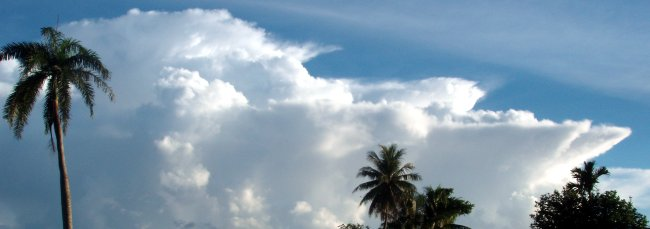# 091 Waves

As an option for introducing waves one can ride a caster board across a wet towel with food color and then across two large sheets of paper such as easel paper used for presentations. Timing the run provides the ability to calculate frequency and period data. With practice, the wheels leave behind sine waves

Equipment

• Super ball or other high bounce ball such a space ball.
• Super ball or other high bounce ball such a space ball.
• Stopwatch
• Very light chain
• Meter stick
• Calculator

## An introduction to the concepts of oscillations

A ball being bounced is a good example of a repeating system. In physics, repeating systems are described by special terms. When the ball repeatedly returns to a previous position and velocity, each repeat is called a cycle. A system that repeats is also known as an oscillating system.

The duration in time for one cycle is called a period τ. Period is often calculated by timing many cycles. Divide the time by the number of cycles to get the period. The units for period are seconds per cycle.

The mathematical reciprocal, dividing the number of cycles by the time, is called the frequency f. The units for frequency are cycles per second. Cycles per second has a special name: Hertz. One Hertz is one cycle per second.

If the distance the ball is dribbled is small (short), the then frequency f is high.

If the distance the ball is dribbled is large (long), the then frequency f is low.

Instructional note: Demonstrate using a stop watch, timing ten to twenty cycles (dribbles). Calculate both the period and frequency.

## Waves on a chain

• The distance from one circle to the next circle on the diagram above is one wavelength λ. In the above diagram one wavelength is 48 centimeters. The symbol λ is the lower case Greek letter "lambda." Wavelength is a space measurement.
• The distance from the middle of the wave to the crest, triangle to triangle, is the amplitude a. In the above diagram the amplitude a is 20 centimeters. Amplitude is a space measurement.

In the diagram above:

• Wave A has an amplitude a of 5 cm
• Wave B has an amplitude aof 10 centimeters
• The wavelength λ of wave A is 20 cm.
• The wavelength λ of wave B is 8 cm.

Amplitude and wavelength are measures of space. Waves have a time component as well. The time component of a wave is the frequency.

• The number of wavelengths (think: crests) that pass a point per unit time is the frequency f. The frequency is also the rate in cycles per second for the wave - the same concept as further above. Frequency is a time measurement and is not shown in the graphs above.
• The generic equation of a basic wave is y = sin(x) where sin is the trigonometric function sine. The actual function for the equation in the first graph in this section is:

$y=20\mathrm{sin}\left(\frac{2\pi x}{48}\right)$

In 1864 James Clerk Maxwell wrote, "For the sake of persons of different types of mind, scientific truth should be presented in different forms and should be regarded as equally scientific whether it appears in the robust form and vivid colouring of a physical illustration or in the tenuity and paleness of a symbolic expression." The Scientists, John Gribbin, page 429. Library, Amazon.

Physics first studied the physical world that we can see, hear, and touch. As physics studied both larger systems - such as the universe, and smaller systems, such as atoms, the physical models imagined became more of a mental picture our mind can comprehend than the actual reality. A theory called string theory imagines the universe consisting of what are mathematically not unlike vibrating strings, yet the reality - if string theory is true - is simply beyond our imagination. The only "reality" - if any - are the mathematical equations that describe the system. Nature is mathematical.

## Wave velocity

When time and space combine linearly, the result may be a velocity. Waves have a velocity. The velocity v for a wave is calculated by multiplying the wavelength λ by the frequency f.

$v=λƒ$

Velocity of a water wave in shallow water where g is the acceleration of gravity and d is the depth of the water.

$v=gd$

Instructional notes: Using a meter stick and stopwatch demonstrate the relationships between frequency f and wavelength λ. Diagram the wavelength and amplitude on the board. Calculate the velocity v of a wave on a chain. Cover the connections to sound waves, water waves.

# 092 Laboratory nine: Mach one: The speed of sound

## Questions

• What is the speed of sound?
• Is the relationship a linear relationship between the time and distance for sound? That is, does sound slow down (or speed up) with distance?

## Equipment

• Wood clappers
• Stopwatches
• Distance measuring equipment
• gloves for the clapper

Instructional note: This lab may take quite a bit of time to gather data in between rain showers. Rain makes hearing the sound difficult. The experiment can usually only proceed in between rain showers.

Laboratory objective: To measure the speed of sound (also known as Mach one).

## Procedure

### Direct measurement over distance

Where there is the linear space to utilize this procedure, this procedure produces the most accurate data. Speed of sound values generated are usually within 5% and can be within 1% of the actual speed of sound on that day. Note that the use of the median for 25 or more time measurements is part of the reason this procedure produces such accurate results. Hand or arm signals enhanced by a towel can be used to communicate. A spinning towel is often used to tell a clapper to "clap again." If available, the ability to use cell phones is a plus when more complex communications are necessary. To direct a unit to take cover due to weather, cell phones are often useful.

1. A clapper with easily visible boards stands at least 200 meters from a group of at least five listeners each with a stopwatch
2. The listeners time the delay between seeing and hearing the boards clap. All times are recorded.
3. The boards are clapped at least five times at each distance. This will generate up to 25 data points per distance. The median time will eventually be used for that distance.
4. The listeners move further away by 50 meters and repeat the sets of measurements. Boards may be visible up to 550 meters depending on conditions.

### Alternate option: Echo Procedure A

1. Measure the distance to an echoing surface that is at least 50 meters or more away.
2. Bang the wood clappers in synchronization with the echo. This takes practice and a good ear.
3. Time 30 claps. This is the time for 30 echoes.
4. Record the time and number of claps.

### Alternate option: Echo Procedure B

1. Utilize an nine member team: clapper, timer, counter, distance measurer, recorder, and four listeners.
2. Measure the distance to an echoing surface that is at least 50 meters or more away.
3. The clapper synchronizes the board claps with the echo. The listeners sit in front of the clapper and use hand signals to indicate whether the board claps are in synch with the echoes.
4. Once synchronization is obtained, the timer starts a stop watch while calling out "Start!"
5. The counter counts the number of claps
6. After a duration of ten seconds the timer calls out "Stop!"
7. The recorder records the number of claps and distance data.

The echo flight distance is the distance out-and-back to the surface off of which the echo bounces.

## Data

Temperature: __________℃

Data table for direct measurement

t1 d1
00
200
250
300
350
400
450
500
550

t1 Median time (s)
d1 is the Distance (m)

Echo procedure A

Location on campus Time for 30 claps (s) Time for one clap (echo travel time) (s) [x] Echo flight distance (m) [y]

Data table for echo procedure B

Location on campus Duration (s) Claps Time for one clap (echo travel time) (s) [x] Echo flight distance (m) [y]
10
10
10
10
10
10
10
10
10
10

## Graph

For the direct measurement, time t1 will be on the x-axis, and the distance d1 will be on the y-axis. For the echo procedures, make an xy scatter graph of the echo travel time [t1] versus the echo flight distance (d1).

## Analysis

The data is likely to be linear. Use the relationship d1~vt1 in Desmos to obtain the speed of sound v.

The graph is a time (duration) versus space (distance) graph, the slope is the speed of sound.

The speed of sound varies primarily as a function of temperature. Use the temperature to determine the speed of sound in air at the time of the laboratory. To make this calculation look up the published speed of sound in air using a site such as HyperPhysics after the laboratory session is over. Cite your source using either APA or MLA.

Perform an error analysis on the experimental value for the speed of sound from your slope calculations versus the published speed of sound. Calculate a percentage error as well: (experimentally measured − published)/published. Do NOT forget the parentheses.

## Discussion

Write up a discussion of the laboratory including a discussion of the results, the theoretic predictions, whether the experimental data agrees with the theory and how good that agreement might be, and potential sources of error. Anything less than 5% is excellent agreement, less than 10% is good agreement.

# 101 Spectra

Instructional note: Spectrometers made from boxes and CDs were used. Both the continuous solar spectrum and a discrete fluorescent light spectrum were observed by the students.

Traditional colors of the visible spectrum in English: Red Orange Yellow Green Blue Indigo Violet. Indigo was apparently inserted by Newton to have seven colors in the "rainbow" as opposed to six.

Beyond violet: ultraviolet. Can cause sunburn, skin cancer. Even higher frequencies include x-rays and cosmic rays (cosmic radiation).

## Continuous versus discrete spectra

If gas discharge tubes are available, a demonstration of continuous versus discrete spectra can be done.

### Continuous spectra

A rainbow is a continuous spectrum produced by the sun. All of the colors "blur" into the next color, there are no distinctive separations in the colors. The sun produces light as a result of being very hot. Things that produce light by getting hot produce continuous spectra.

### Discrete spectra

A gas discharge tube contains one specific gas. Gases produce discrete spectra. The colors do not blur into each other but are separated by gaps where no color appears. Gases with a small atomic number such as hydrogen and helium clearly show separated spectral lines. The lines are related to the nature of the atomic structure of an atom. Atomic structure is a part of a later chapter in this text.

# 102 Color and the colors of light

## Questions

What are the primary colors of light? What are the secondary colors of light? How is color produced by monitors, cell phone screens, lap top computer screens, and televisions? What is your favorite color and why?

In this three part laboratory we will explore the colors of light and systems of organizing colors. In the first part of this laboratory we will look at how televisions and monitors produce the colors of the rainbow. In the second part we will learn about hue, saturation, and luminosity. Finally we will learn some color keywords specific to the world of computers and the world wide web.

1. RGB: Red Green Blue color specification
2. HSL: Hue Saturation Luminosity color specification
3. X11: Using words to specify colors

## I. RedGreenBlue: The RGB lights that make color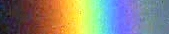Rainbows are a blend of colors that run from purple to red. Rainbows are caused by refraction and internal reflection in a raindrop. Although the colors blur into each other, rainbows appear to have six distinguishable colors in English: red, orange, yellow, green, blue, and violet. Newton's opinion was that there were seven colors: red, orange, yellow, green, blue, indigo, and violet.

Yet television screens, cell phone screens, and computer monitors can produce all the colors of the rainbow using just three colors of light. All colors can be produced with only three colors of light: red, green, and blue (RGB). Red, green, and blue are called the primary colors of light. In this laboratory we will use HTML code to control the colors of light on a computer monitor.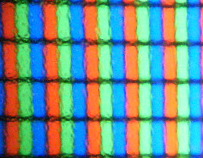A five by four grid of 20 pixels

The screen has millions of sets of tiny picture elements called pixels. Pixel is short for picture element. Each pixel is comprised of three color phosphors, one of each of the primary colors of light: red, green, and blue. Commands to set a particular pixel color are issued as a three digit base 16 (hexadecimal) number. The first digit indicates how bright to make the red phosphors, the second digit indicates how bright to make the green phosphors, and the third digit indicates how bright to make the blue phosphors.

## Controlling the red, green, and blue phosphorsGreen plus on white background

The brightness of phosphor is set by a number. Zero is all the way off. Sixteen is all the way on, full on. Computers, however, do not count in base ten. Our computers work in base 16. Base 16 is called hexadecimal. The problem is that base ten has no single digit "number" symbols for the numbers from 10 to 15. So in hexadecimal the letter A is used for "10", B for "11", C for "12", D for "13", E for "14", and F for "15". The number after F is "one sixteen and zero ones" which is written "10".

The following table shows the base ten number and the corresponding hexadecimal numbers.

 Base 10 Base 16 0 1 2 3 4 5 6 7 8 9 10 11 12 13 14 15 16 0 1 2 3 4 5 6 7 8 9 A B C D E F 10

The colors on a computer screen can be controlled by a hexadecimal number between 0 (off) and F (full on), with 8 being half way on.

HexPhosphor dot status
0off
4quarter of full brightness
5about one third of full brightness
8half of full brightness
Cthree-quarters of full brightness
Ffull brightness for that color

## HyperText MarkUp Language (HTML)

Getting the computer to actually process the color number command will require enclosing the command in a simple program. We will use HTML to work with our colors.

To write and display the HTML, use the onlinehtmleditor.net site. This will allow one to see the results of edits immediately.Alternatively, if for some reason the Internet is not available, write the HTML with a text editor.

Instructional note: The writing of the HTML code is usually done first as a demonstration by the instructor. On Ubuntu the default text edit is gEdit, Lubuntu uses Leafpad, and Windows has Notepad. On ChromeOS the Chrome app Caret is highly recommended by the author. The demonstration includes how to save the web page code. When using Notepad, change the file type to All Files or Notepad will append a .txt filetype extension. Opening the web page with a browser is also demonstrated. Using Ctrl-o for "open" works in most browsers except on Apple where Cmd-o is used. The instructor may also demonstrate resizing the text editor and browser window so both use half the screen. This permits both to be open. The student can then save in the editor and immediately refresh in the browser.

In the text editor you will have to type the code shown exactly as it is typed in the example below.

<html>
<style>
body {background:#F00}
</style>
<body>
<p>Text</p>
</body>
</html>


The "#" in front of the "F00" tells the computer that a hexadecimal number is being used. Note that the f can be lowercase f or uppercase F. #F00 is "red full on, green off, blue off. The "00" are the digits "zero zero" not the capital letter "O"

Computers are very literal: the code must be identical to the code above. Any error, even a single letter wrong, will cause your web page to fail. After typing the code exactly as seen above, save your file as an HTML file.Type the file name into the first blank in the dialog box. Call the file "labten.html" The file can be saved to the location of your choice: the Documents folder or a USB flash drive. If using Microsoft Windows Notepad, set the file type to All Files before saving. On ChromeOS save to the Downloads folder. Remember where you chose to save the file!

Open a web browser such as Google Chrome, Safari, or FireFox. Use Ctrl-o open the saved file. Locate the saved file and open the file.Use the editor to change the color command. Once open change the color to #0F0 and then choose File:Save (NOT Save as...!).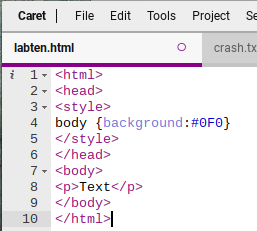After doing this, return to the browser and click on the refresh button (Ctrl-r)to update the web page with the #0FO code. #0FO is red off, green full on, blue off.

## Explorations

Change the background color to the following hexadecimal numbers to see what color results.

• What color is #000?
• What color is #00F?
• What color is #0F0?
• What color is #0FF?
• What color is #F00?
• What color is #F0F?
• What color is #FF0?
• What color is #FFF?
• What color is #555?
• What RGB hexadecimal numbers makes yellow?
• What RGB hexadecimal numbers makes purple (not magenta)?
• What RGB hexadecimal numbers makes orange?
• What RGB hexadecimal numbers makes brown?
• What is the RGB for your favorite color?

RGB is how computers display colors, but try to imagine what combination produces orange, pink, baby blue, sea blue, or brown. Our brains do not think well in RGB. The next system is a system that uses a color wheel to organize the colors in rainbow order, controlling the amount of color and brightness with two percentages.

The primary colors of light are red, green, and blue.
The secondary colors of light are yellow, cyan, and magenta.

• Primary colors
• Red: #F00
• Green: #0F0
• Blue: #00F
• Secondary colors
• Yellow: #FF0
• Cyan: #0FF
• Magenta: #F0F

## II. Hue, Saturation, Luminosity

Rainbow hue analysis
Closest
hue name
Hue angle
red9
orangered17
orangered24
orangered24
darkorange26
orange30
orange36
orange43
goldenrod48
gold52
yellow64
greenyellow77
chartreuse88
lime111
springgreen137
aquamarine165
cyan184
deepskyblue200
royalblue217
blue230
blue236
blue239
slateblue250
indigo270
purple303

Hue values based
on the analysis
of an actual
image of a
rainbow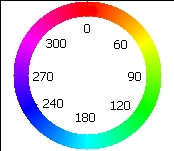Another way to describe color is by using a color wheel or color circle. The word hue is used for the word color when working with a color wheel. On a color wheel the colors are arranged in a circle in the same order that the colors appear in a rainbow. The primary colors of light, red, green, and blue, are spaced evenly around the color wheel. The hues are specified by their angle around the 360° hue wheel.

The hues are arranged in rainbow order. Red, the first color in the rainbow, is placed at the top and is assigned a color angle (or hue angle) of 0°. Once around a circle is 360°.
Green is placed one third of the way around the circle at 120°.
Blue is placed two-thirds of the way around the circle at 240°.

On the right are the hue angles for colors taken from measurements of the hue angles for pixels in an actual image of a rainbow. The color names are approximate X11 color names from part III of the lab. For many of the hue angles there is no exactly matching X11 color of appropriate hue, saturation, and luminosity.

Saturation is how much of a hue is displayed. Zero percent saturation is no color. Zero saturation results in black, grays, or white. The maximum saturation is 100%. At a saturation of 50% the color is tinged with a grayishness but is distinctly of a hue.

Luminosity is how bright, how luminous, the color should be. Zero percent results in black, while 100% makes the color so bright that it appears white. 50% yields the "purest" colors to the human eye, above 50% and the brightness starts to wash out the color.

The color command is hsl for hue, saturation, and luminosity. The command structure is:

hsl(0 to 360,0% to 100%,0% to 100%)

The first number is the hue angle on the color wheel, the second number is the saturation in percent, and the third number is the luminosity in percent. Try the following code, do not forget the percentage signs. Do NOT put any spaces in the "hsl(0,100%,50%)" color command.

<html>
<style>
body {background:hsl(0,100%,50%)}
</style>
<body>
<p>Text</p>
</body>
</html>

• What color is hsl(0,100%,50%)?
• What color is hsl(30,100%,50%)?
• What color is hsl(60,100%,50%)?
• What color is hsl(120,100%,50%)?
• What color is hsl(180,100%,50%)?
• What color is hsl(240,100%,50%)?
• What color is hsl(300,100%,50%)?
• What color is hsl(360,100%,50%)?
• What color is hsl(20,50%,40%)?

The primary colors of light are spaced evenly one-third of the way around the color circle with red at 0°, green at 120°, and blue at 240°. Half way in-between are the secondary colors of light yellow at 60°, cyan at 180°, and magenta at 270°. You might recall that yellow is also #FF0: full on red, full on green. What primary colors are turned on full for cyan and magenta?

The newest variation of HSL is HSLA, where the A stands for "alpha". Alpha specifies the level of transparency for a color. A sample HSLA web page with a Halloween theme. An HSLA color command looks like:

hsla(30,100%,70%,.55)

where the last number is the transparency. Zero is fully transparent, one is opaque color. Numbers in-between are partially transparent.

## III. X11 Color names

No culture uses numbers for colors. People use color words such as red, yellow, green, cyan, blue, and magenta. A page at Luminoso details graphically the results of an XKCD color survey that explored names of colors. There are gender differences in color preferences.

In the world of web pages there are X11 color names. The list at the end of the lab is the X11 color names in hue angle order. The hue angle is given for reference. Note that the color command in the style section controls the color of the text.

<html>
<style>
body {background:linen;
</style>
<body>
<p>Text</p>
</body>
</html>


X11 color names are popular because they are easy to remember. Critics note that the color names are in English and that the naming system is not logical nor consistent.

## Extensions

### Web pages

Web pages often include headings, images and links. Although no web page author would do so, the style commands below include all three computer color systems.

<!DOCTYPE html>
<html lang="en">
<meta charset="UTF-8">
<title>Page title</title>
<style>
body {background:#FEF;
color:hsl(240,100%,50%)}
h1 {color:indigo}
</style>
<body>
<p>Paragraph text</p>
<p>
<img src="http://www.comfsm.fm/~dleeling/marlin.jpg">
</p>
<p>
<a href="http://www.comfsm.fm/~dleeling/marlin.jpg">
</p>
</body>
</html>


### Colors and meanings

Do colors have meanings? Try taking the Global Color Survey. Over 200,000 people have been surveyed. Based on that survey the following meanings tend to be associated with the color that follows.

Happy - Yellow
Good Luck - Green
Good-tasting - Red (tomato)
Dignity - Dark Blue
High Technology - Silver
Sexiness - Red (tomato)
Mourning - Black
Expensive - Gold
Inexpensive - Brown
Powerful - Red (tomato)
Dependable - Blue
High Quality - Gold
Nausea - Muted Yellow
Deity - White
Favorite Color - Blue
Least Favorite Color - Dark Yellow

### X11 Color names full list

angle x11color
Black
DimGray
Gray
DarkGray
Silver
LightGrey
Gainsboro
WhiteSmoke
White
00 RosyBrown
00 IndianRed
00 Brown
00 FireBrick
00 LightCoral
00 Maroon
00 DarkRed
00 Red
00 Snow
06 Salmon
06 MistyRose
09 Tomato
15 DarkSalmon
16 OrangeRed
16 Coral
17 LightSalmon
19 Sienna
25 Seashell
25 Chocolate
28 SandyBrown
28 PeachPuff
30 Peru
30 Linen
33 DarkOrange
33 Bisque
34 BurlyWood
34 Tan
34 AntiqueWhite
36 NavajoWhite
36 BlanchedAlmond
37 PapayaWhip
39 Orange
38 Moccasin
39 Wheat
39 OldLace
40 FloralWhite
43 Goldenrod
43 DarkGoldenrod
48 Cornsilk
51 Gold
55 PaleGoldenrod
54 Khaki
54 LemonChiffon
56 DarkKhaki
60 Beige
60 LightGoldenrodYellow
60 Olive
60 Yellow
60 LightYellow
60 Ivory
80 OliveDrab
80 YellowGreen
82 DarkOliveGreen
84 GreenYellow
90 LawnGreen
90 Chartreuse
120 DarkSeaGreen
120 ForestGreen
120 LimeGreen
120 LightGreen
120 PaleGreen
120 DarkGreen
120 Green
120 Lime
120 Honeydew
147 MediumSeaGreen
146 SeaGreen
150 SpringGreen
150 MintCream
157 MediumSpringGreen
160 MediumAquamarine
160 Aquamarine
174 Turquoise
177 LightSeaGreen
178 MediumTurquoise
180 DarkSlateGray
180 PaleTurquoise
180 Teal
180 DarkCyan
181 DarkTurquoise
180 Aqua
180 Cyan
180 LightCyan
180 Azure
187 PowderBlue
195 LightBlue
195 DeepSkyBlue
197 SkyBlue
203 LightSkyBlue
207 SteelBlue
208 AliceBlue
210 DodgerBlue
210 SlateGray
210 LightSlateGray
214 LightSteelBlue
219 CornflowerBlue
225 RoyalBlue
240 MidnightBlue
240 Lavender
240 Navy
240 DarkBlue
240 MediumBlue
240 Blue
240 GhostWhite
248 DarkSlateBlue
248 SlateBlue
249 MediumSlateBlue
260 MediumPurple
271 BlueViolet
275 Indigo
280 DarkOrchid
282 DarkViolet
288 MediumOrchid
300 Thistle
300 Plum
300 Violet
300 Purple
300 DarkMagenta
300 Magenta
300 Fuchsia
302 Orchid
322 MediumVioletRed
328 DeepPink
330 HotPink
340 PaleVioletRed
340 LavenderBlush
348 Crimson
350 Pink
351 LightPink

# 111 Refraction, lenses, terminology, and vision

Refraction: the bending of light when a light beam passes at an angle to a surface between two optically transparent materials of differing densities.

Monday demonstration included a laser, prisms, and half-round dishes with water to show bending of light. Alternately, a video on optics, reflection, refraction, and total internal reflection can be shown.

Wednesday moves on to introduce convex and concave lenses. Use magnifying lens to produce an image. Introduce object distance and image distance. If sunny, use the pinhole and paper punch hole camera to demonstrate pin hole cameras and effect of aperture size on clarity. Move on to explain human eye, iris and pupil. Move on to cover myopia, hyperopia, presbyopia, and remedies.

Another demonstration that fits here is the coin under the glass of water.

If a video was shown on Monday, use Wednesday to demonstrate reflection, refraction, and total internal reflection. Provide definitions. On a sunny Friday with a long enough, flat enough stretch of straight road, an inferior mirage might be visible and then explained.

# 112 Laboratory 11: Reflection and refraction

Light bending

## Questions

• Is there a linear relationship between the object distance and the image distance for a mirror?
• Is there a linear relationship between the depth of an image below the surface of water and the actual depth of the object below the surface of water?
• What is the index of refraction for water implied by that relationship?

Before breaking into groups and making measurements, ask the students to predict the relationship for the mirror. Label any theories by the last name of the student who first proposes the theory, "_________'s theory of reflection."

A focus on education: Note that while science tends to explore questions, these questions can be rewritten as student learning outcomes. Students will be able to determine the nature of the relationship between the object distance and image distance for a plane mirror. If the relationship is linear, students will be able to determine the slope and intercept for the relationship. Students will be able to determine the nature of the relationship between the object depth and image depth for am object underwater. Students will be able to calculate the index of refraction for water based on the slope of the relationship between the object depth and image depth for an object underwater.

## Hypotheses

• There exists a mathematical relationship between the distance of an object in front of a mirror and the distance of the image "behind" the mirror.
• There exists a mathematical relationship between the actual depth of an object and the apparent depth of an object seen below the surface of the water.

The word "image" is related to the word "imaginary." The image of the object is "not really there."

## Procedure

### Image distance behind a mirror: index of reflection

Place an object in front of the mirror. Measure the apparent distance x₁ of the image of object "behind" the mirror. Measure the distance y₁ from the object to the mirror. Record the distances in centimeters. Note that to obtain a constant known as the index of refraction for water, the image distances will have to be placed on the x-axis and the object distances will have to be placed on the y-axis. This puts the independent variable on the y-axis and the dependent variable on the x-axis. For consistency, both of the two parts will record image distances as x₁ and object distances as y₁.

## Data tables

### Data table one: reflection

Reflection data
x₁y₁

Where:
x1 is the image distance in centimeters
y1 is the actual object distance in centimeters.

A note on variables: the letter "i" is used for the square root of one and current. The letter "o" is simply confusing.

### Image depth under water: index of refraction

When an object is underwater, the object appears closer. The actual depth of the object is called the object depth y₂ in this laboratory. Measure all distances from the top surface of the water. The object, especially when viewed from an angle above the water, will appear to be shallower that the actual depth y₂. This is an image of the object caused by refraction. Technically refraction is due to bending of the light as the light rays leave the water and enter the air. The apparent depth of the object under the water is the image depth x₂. To accurately determine the image depth you may have to move your head left and right above the object.

Measure the object depth y₂ and the image depth x₂ for the object under the water. Start with an empty tub, recording both the object depth y₂ and the image depth x₂. Add water and repeat the measurements. Continue until the tub is full. Record the distances in centimeters.

An alternate procedure is to use multiple beakers and graduated cylinders each with a different amount of water. This procedure has an advantage when the laboratory is done in locations where the water may go off during the day. Large graduated cylinders on the order of 50 cm tall tend to produce more accurate results.

Be careful to measure the actual and apparent depth below the surface of the water, not the top of the container.

### Data table two: refraction

Make measurements at many different depths. Run an mathematical analysis on the results, producing the appropriate graphs and mathematical relations.

Refraction data
x₂y₂

Where:
x₂ is the image distance in centimeters
y₂is the object distance in centimeters.

## Graph: xy scattergraphs

Desmos is able to chart two data tables onto a single graph. If using spreadsheets to perform the analysis, then two charts are likely to be necessary.

## Data analysis

Reflection analysis: If using Desmos and the data appears to be linear, determine the relationship between the image distance x₁ and the object distance y₁ using the relationship y₁~mx₁.
Refraction analysis: If using Desmos and the data appears to be linear, determine the relationship between the image distance x₂ and the object distance y₂ using the relationship y₂~nx₂.

For the apparent depth data, refraction theory suggests that the slope of the line should be equal to the index of refraction for water n. Look up the published index of refraction for water. Report this value and cite your source using either APA or MLA format. Run an error analysis to determine the percentage difference between the experimental index of refraction for water (the slope of the line on the second graph) and the published index of refraction.

$percentage difference = (experimental slope−theoretic index of refraction for water) theoretic index of refraction for water$

## Discussion and conclusions

Discuss the findings. Is there a mathematical relationship? Is the relationship linear or non-linear? Report a conclusion as to the relationship between the object distance and image distance for reflection in a flat mirror. If there are one or more mirror theories, discuss which one might be correct and why. Discuss the accuracy of your results for part two, the index of refraction for water. Discuss also any difficulties you encountered.

The lab will also be marked on grammar, vocabulary, organization, and cohesion.

Instructional note: Post-lab next day wrap-up could include refractive index of water and implication for divers working underwater. Relative distance and magnification. Alternatively, inferior mirages can be demonstrated if the conditions on campus, both physically and in terms of weather, permit the observation of mirages.

# 121 Voltage, Current, Power, and Resistance

If one thinks of standing under a waterfall, there are two factors that contribute to the force of the water. The first is how far the water falls - how high is the waterfall. The second is how much water is falling - the amount of water. The higher the waterfall, the greater the force on a person standing underneath. The greater the amount of water, the greater the force on a person standing underneath. The height of a waterfall is called "the head" and the amount of water flowing is called the "current." Similarly, electricity flowing in a circuit is characterized by two factors: voltage and current. Similar to head, the voltage is a measure of the electromotive "pressure" in the circuit. The amount of electricity flowing is called the "current." These are just "analogies" and they are not exactly comparable, but the factors for a stream can help you think about electricity.

Voltage: The voltage is the amount of electrical "pressure" in a circuit. Voltage is measured in volts using a voltmeter. The letter for voltage is a capital letter V. This can be confusing because the variable for Voltage is capital letter V and the units for voltage is also a capital letter V.

Current: The current is the amount of electricity flowing in a circuit per unit time. Current is measured in amperes (amps) using an ammeter. The letter for current is the lower case letter i.

Power: Power is the amount of electrical energy consumed per second. Power can be calculated from the relationship P = iV where P is the power in Watts, i is the current in amperes, and V is the voltage in Volts.

Resistance: The resistance refers to the resistance to the flow of electricity in a circuit. Resistance is defined by the drop in voltage as a current flows through a load. A load can be a light bulb or any other electrical appliance. Loads include coffee pots, fans, rice cookers, ovens, refrigerators, cell phone speakers, microwave ovens, televisions - anything powered by electricity. The letter for resistance is a capital letter R. Resistance is calculated by using Ohms law: V = iR. The units for resistance, technically volts per ampere, are called Ohms and use a capital Greek letter omega: Ω

The symbol for resistance in a circuit diagram is a repeated "w" shape.

A coffee maker uses 900 Watts according to the plate on the bottom of the coffee maker. The coffee maker is a standard 120 volt appliance. Wall outlets supply 110 to 120 volts. Using Ohms law and the power relationship above we can calculate the resistance and the current. To calculate the resistance we would need the current. The power relationship above can give us the current. P = iV or i = P ÷ V. 900 ÷ 120 = 7.5 amps. Using the current i = 7.5 amps, the resistance R = V ÷ i = 120/7.5 = 16 Ω

A wall outlet is typically designed to handle no more than 18 amps. One can add the amperages for appliances and if the total exceeds 18 amps, the circuit is overloaded and can overheat. In theory a circuit breaker should trip (open and disconnect the circuit) if the amperage exceeds 20 amps on a single phase household circuit. If the breaker does not trip, then there is a risk of fire.

Cash power is used across Micronesia. The units on the cash power meter are kilowatt hours: one hour of 1000 Watts of power. Or two hours of 500 Watts. Or ten hours of 100 Watts. Power is money. What uses the most power in a house?

# 121 Magnetism

Magnets are materials that attract certain materials. Materials attracted by a magnet are referred to as magnetic. Many compounds that contain iron are magnetic. Magnets can be made of iron compounds or more exotic mixtures such as aluminum, nickel, and cobalt as in alnico magnets. Magnets are typically made of metallic compounds.

Magnets produce a magnetic field.

Observe a magnet and iron filings. Sketch the field lines.

Magnets always attract magnetic materials. Magnets, however, can attract or repel other magnets.

This leads to the model that magnets have two "ends." The planet earth also has a magnetic field. When allowed to move freely, magnets align themselves with the field. The end of the magnet that points north is called the north pole of the magnet.

Poles that are the same repel each other. "Likes repel."

Poles that are different attract each other. "Opposites attract."

Demonstrate a Faraday coil with a magnet, a coil, and a microammeter, if available.

# 122 Laboratory 12 Circuits, conductors, and Ohm's Law

## Questions

What is a closed, open, and short circuit? What materials conduct electricity? How does electrical conduction relate to heat conduction? Is there a linear relationship between the current and voltage for an electrical circuit?

Due to equipment limitations, the following parts do not have to be done in any particular order.

## Terminology

Electrical conductor: An electrical conductor allows electricity to flow through the material.

Electrical insulator: An electrical insulator does not allow electricity to flow through the material.

Cell: A single "battery" is call a "cell." Two or more cells connected together are called a "battery." Each "dry" cell produces 1.5 volts. The symbol for a cell in an electrical diagram is:

In part III of today's laboratory the resistance will be obtained from the slope of a current versus voltage graph.

Schematic diagram: A schematic diagram is a symbolic representation of a circuit using abstract symbols for the power source and loads.

Closed circuit: A closed circuit is one in which electricity flows. In a circuit with a light bulb, the circuit is a closed when the light bulb lights.

Short circuit: A short circuit is one in which electricity flows but not through a load. In a circuit with a light bulb, the circuit is a short circuit when the wire gets hot and the bulb does not light. The current is flowing from the cell directly back into the cell without passing through the load.

Open circuit: An open circuit is one in which electricity does not flow. In a circuit with a light bulb, the circuit is open when the light bulb does not light and the wire does not get hot. No current flows in an open circuit.

## Laboratory experiments

### Part I: A cell, a wire, and a bulb [i]

Using only a single 1.5 volt cell, a single wire, and a flashlight bulb, make the bulb light up. The materials are on the north table.

In electrical physics a closed circuit is one in which electric current flows. When the bulb lights, the circuit is a closed circuit. The circuit may also be a closed circuit without lighting the bulb. When this happens the wire will get hot. If the wire gets hot, then you have created a short circuit.

A circuit in which no electricity flows is an open circuit. In an open circuit the bulb does not light and the wire stays cool. Draw [i] three sketches. Draw a sketch of a closed circuit that lights the bulb. Draw a sketch of a short circuit. Draw a sketch of an open circuit. Sketch what you actually see with your eyes, drawing the battery, bulb, and wire. Do not use images from the Internet. Turn in your sketch.

### Part II: Conductors [d][t]

Using the two-cell battery and the bulb, test the materials provided on the middle table for conductivity. Note whether the brightness of the bulb varies for any of the materials. Make a table that indicates whether the material is a conductor or a non-conductor of electricity. If laboratory six determined heat conductivity, then include in the same table whether that material was a conductor of heat or a non-conductor of heat in laboratory six. Turn in the table.

### Part III: Ohm's law [d][t]

Ohm's law indicates that the voltage V is equal to the current i times the resistance R for a circuit. Three circuits are provided. All three circuits include a two-cell battery, a switch to open and close the circuit, a voltmeter that measures the voltage, and an ammeter that measures the current in amperes. The only difference in the three circuits is the number of resistances. Each bulb is a resistance. Each bulb is wired in series, so the resistances add directly. The circuits with one and two bulbs are shown in the schematic diagrams below.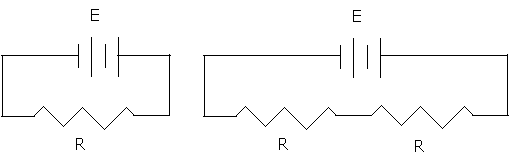Using the ammeter, measure the current i in amperes. Using the voltmeter, measure the voltage V in volts. Note how the meters are connected to the circuit. The electricity in the circuit flows through the ammeter. The ammeter is measuring a rate of flow of electricity. The voltmeter is arranged "across" a single light bulb. The voltmeter is measuring an electrical "pressure difference" across a single light bulb.

For each of the three circuits measure the current i and the voltage V and record the data in a table [d] [t]. Include in your table the value of the voltage and current when the circuit is "open" (the switch is not closed).

Note also the brightness of the bulb for each set-up and whether the bulb is hot, warm, or cool.

As you record the current and voltage for each of the three set-ups, calculate the resistance for each by dividing the voltage by the current. Does the resistance have a relationship with the brightness and how hot the bulb is?

## Graph

Make an xy scattergraph of the data in part three. Use the current i as the x variable and the voltage V as the y variable.

Turn in the table from part II, and the graph from part III.

# 131

## Reading a table of elements

Elements are materials that consist only of atoms with the same atomic number. The atomic number for an atom is the number of positively charged protons in an atom.

The number of electrons is equal to the number of protons. Electrons orbit the protons. This is an oversimplification of reality, but the reality is that the electron is actually a probability wave function. The mathematics to get the electron right are beyond most people on the planet. Best to think of the electron as zipping around the proton. The drawing below left is an abstract image of a hydrogen atom with its single proton and single electron.

The atomic number is determined by the number of protons. Hydrogen has an atomic number of one. The atomic mass is determined by the number of protons and neutrons. While hydrogen always has one proton in the nucleus, the number of neutrons can vary from none to two. Hydrogen with one neutron is called deuterium and has an atomic of mass of two, hydrogen with two neutrons is called tritium and has an atomic mass of three.

Electrons not only go around the proton, but electrons also spin around their own center. Some spin "clockwise" and some spin "counter-clockwise." Spinning is one of the fundamental movements of the universe. Electrons tend to pair up. Electrons are particles, not thinking beings. If electrons could think, we would say electrons like to be in pairs, one spinning one way and the other spinning the other way. Hence, to continue to anthropomorphize, a single electron is lonely and wants an opposite spin partner. The result is that hydrogen's electron will either try to snatch a partner electron from another element or simply run away to an element in which it can become a pair. Two hydrogen atoms will remain bonded together, sharing their electrons, as this puts two electrons together.

Helium is atomic number two because of the two protons in the nucleus. Along with two protons are two neutrons, thus the atomic mass is four.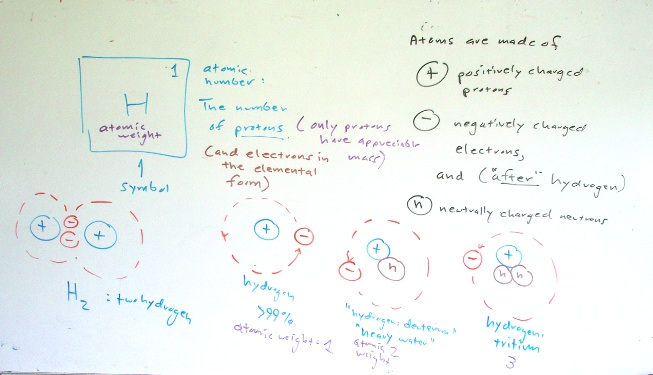# 132 Laboratory 13: Floral pigments as litmus tests for acids and bases

Chemistry from the flower garden into the kitchen

## Questions

What floral pigments make the best detectors of acids and bases? What substances are acids, bases, or neutral?

This laboratory explores whether flower petal pigment solutions are good indicators of acids and bases. The flower petal pigment solutions are produced by boiling flower petals in water. If a flower petal solution is found that is a good detector of both acids and bases, then that solution will be used to determine whether unknown substances are an acid, a base, or neutral. Flower pigment solutions are also called floral pigments in this laboratory.

• Hypothesis: Flower pigment solutions can be used to detect acids and bases.
• Analysis: Use a flower pigment solution to determine whether unknown substances are an acid or base.

In the English language acids are said to be sour or tart. Bases are often said to be bitter.

Demonstrate litmus paper and its reaction to the known acid and base. Note that litmus paper is not always available. In lieu of litmus paper paper, one can produce litmus solutions.

## Phases

There are three phases to this laboratory.

1. In the first phase you will gather flowers to use as tests for determining whether a substance is an acid or a base. Pick common flowers. Do not pick flowers that belong to someone. Do not pick the orchids in front of the library or other rare flowers. You might also try colorful leaves, again, avoiding rare plants.
2. In the second phase you will determine which flower produces the best floral fluid for determining whether something is an acid, a base, or neutral. After boiling the petals, the fluid will be tested with a known acid and a known base. You are looking for a flower fluid which shows a distinct and different color change for the known acid and the known base. In this lab lime juice is usually our known acid and baking soda is usually our known base.
3. In phase three you will use that one "best" flower fluid to test liquids to determine if they are an acid, a base, or neutral. When working with test tubes, be sure to rinse them well between tests. Be careful, some unknowns are poisonous, others can burn your skin.

## Equipment

• flowers
• hot plate
• tea pot to heat water
• many small and medium sized beakers
• numerous small test tubes
• spatulas for placing powder into test tubes
• mortar and pestle for grinding aspirin, anti-acid tablets
• paper towels
• transparent tape
• Gloves for handling hot beakers
• sheets labeling knowns and unknowns
• x11 color chart
• Fruit picker pole for flowers

## Phase I: Collecting

Collect the flowers and leaves, boil them.

## Phase II: Finding a litmus solution

Test each floral solution for color changes, including changing to clear or clear changing to a color.

Make a table [d][t] of your results for the flower that produced a color change for both the known acid and the known base. Specifically record the color change, if any, caused by the known acid or the known base. The table should include:

• flower name
• original color of solution
• color change, if any, when known acid is added
• color change, if any, when known base is added

Look for a change in color as in a change in hue, of color angle, not a change in saturation or luminosity.

Flower or leaf nameOriginal color (neutral)color change for an acidcolor change for a base

Note that you are not testing to determine whether the floral litmus solution is acidic or basic. The floral solution is being used as an indicator fluid. Whether the fluid itself is acidic or basic is not determined by this laboratory, but in general the floral litmus solutions are near neutral.

## Phase III: Testing household substances

Use flower petal solutions that change color in a consistent way for acids or bases. If you find one that changes color for both acids and bases, use that one. If you find that one changes colors for acids and another changes color for bases, use both of those two floral pigment solutions. These floral solutions can also be called "litmus" solutions.

Use the solution to analyze [a] whether the following household substances are acids or bases (different compounds might be made available on lab day):

• acetone
• alcohol
• aspirin
• ammonia
• baking powder
• baking soda
• corn starch
• cream of tartar
• diluted bleach
• drain clog dissolvers (Drano, Seligs)
• limes (fruit)
• mineral lime (coralline lime)
• rolaids
• salt
• sugar
• vegetable oil
• vinegar

Make a table [at] of your results for the household substances noting the color change and whether the substance is an acid, a base, or neutral. Only put the substances present in the laboratory on the day of the laboratory. Not all substances will be available.

## Discussion of results and conclusion [c]

[c] Conclusion. Discuss what flowers showed the most distinct color change for the known acid. Discuss what flowers showed the most distinct color change for the known base. Discuss any difficulties you encountered.

Notes for spring 2008: The chromatograph appears to have failed, despite prior work suggesting it could be done. The only difference was the use of a rock pounder in the functional chromatograph and the use of a mortar and pestle in class. Not enough crushing? The upshot is that pens or MM's would work better and this section of the lab was effectively not done.

Need more substances to test next term: ammonia, cream of tartar, acetone, drano...Could use more droppers.

Post-lab marking notes: Students remain unclear on acids versus bases. That concept in and of itself appears to be sufficient for the laboratory. Drop the chromatograph. Label the "known" substances more clearly. "Lime. Citrus fruit. Sour. Known acidic solution. Baking soda. Bitter. Known basic solution." Test more substances. Dilute bleach to see if oxidizing effect can be reduced. Test neutral fluids such as rubbing alcohol, vegetable oil. Dilute drain cleaner. Look for and acquire other substances such as cream of tartar, baking powder, coralline lime powder.

Dark red leafed coleus works almost better than Hibiscus tiliaceus. Early morning H. tiliaceus does not produce as much pigment as afternoon H. tiliaceus. Cream of tartar was impossible to find spring and fall 2008, and both limes and baking soda were scarce on island the day before the lab in spring 2008.

Spring 2008 lab report follow-up: Students thought the floral fluids were acid or base and that we were testing them to see which were acidic and which were basic. Misunderstood the whole role of a "known" as used to find a best possible litmus fluid.

This lab needs further refinement, the language below and comment outs, prior to fall 2008 run. The three phase piece was added for 11:00 and really helped. The coleus made an excellent indicator. Maybe phase one should be tossed and coleus grown prolifically on campus?

Fall 2008: trying a more distinct three table layout in sequence from the door. Students have difficulty with colors, print the x11 color chart on a color printer for reference in class. Worked well. Hibiscus rosa-sinensis was the consensus choice, although one group did well with a high concentration Spathoglottis plicata. Coleus was not as clearly indicative of bases. Two different coleus performed differently, the dark purple leafed varieties may make better indicators.

I intentionally did not want to put in fill-in the blank table. At this point in the term I want to see what the students will do if left to their own devices. That said, the ul list was added post-hoc fall 2008 as the tables still really confusing. The whole idea of finding a known predictable test substance and then using that new found standard is completely new to the students.

Students tend to be deeply confused about the conceptual underpinnings of the laboratory. The concept of a litmus fluid eluded them. The students often consider the floral solutions to be acidic or basic, misunderstanding their role as indicator solutions. Introducing the laboratory using litmus paper to test the knowns appears to help, but the point of the laboratory is to be able to determine whether a solution is acidic or basic without having to resort to litmus paper. T

# 142 Laboratory 14

Laboratory fourteen is a term end exploration of a physical system with an underlying mathematical model. The laboratory functions as a term end practical in that the students must decide how to put together an appropriate full length report complete with an introduction, equipment list, procedure, data tables, graphs, analysis, discussion, and conclusions. One of the intents is to see what the students can do when given only minimal guidance and little in the way of formal framework.

The lab chosen varies from term to term and has included exploring the index of refraction of glass, the subsequent bounce heights of a ball, and an exploration of frisbee velocity versus distance. Another intent of the laboratory is to focus again on the nature of possible mathematical models that a system can exhibit. The laboratory chosen should lead to a better understanding of questions such as those that follow.

The graph plots three data sets for three different possible mathematical models. One data set is plotted as squares, one as circles, and a third as triangles.

1. Which shape plots linear data?
2. Which shape plots non-linear data?
3. Which shape plots random data?
4. For the data that plots linearily, make a mathematical estimate of the slope.

# Flight of the Frisbee, Flying rings, and Mathematical Models

## A laboratory practical examination and exploration

The distance that an object thrown parallel to the ground at a height h above the ground is given by the equation:
$d=\sqrt{\frac{2h}{g}}×{v}_{\mathrm{horizontal}}$
Where d is the distance the object will travel, h is the height, g is the acceleration of gravity, and vhorizontal is the speed with which the object is thrown. In this situation the launch angle relative to the ground is 0°. The object only travels horizontally for the duration of the fall time. A graph of velocity versus distance will be linear of the form y = mx where the slope m will be the square root constant in the equation above. For a horizontal throw one meter above the ground the slope will be 0.45 seconds. This equation holds for objects that fall when thrown horizontally such as balls. Throw the object with twice the velocity, the object will go twice as far.

Objects that fly such as flying disks and flying rings should travel farther and outperform a non-flying object. This laboratory seeks to explore whether flying disks and rings outperform non-flying objects and to determine the nature of the mathematical relationship between velocity and distance.

## Data Gathering

Gather velocity versus distance data. Use distance the disk flies divided by the time of flight to calculate the velocity. Note that this velocity is technically a mean velocity as the speed of the disk decreases during the flight. Launch velocities, however, are difficult to measure. Use the data gathered to determine the mathematical relationship between velocity and distance. Equipment will include flying disks, flying rings, timing devices, and distance measuring capability. Stopwatches, GPS units useful. Radar guns worth trying for launch velocity.

## Questions to consider and to which to respond

1. Performance versus non-flying objects
1. Does a graph of velocity versus distance demonstrate that flying objects outperform non-flying objects?
2. How do they demonstrate this?
3. By what factor do flying objects outperform non-flying objects? Suggestion: explore the percentage difference between the experimental slope and the non-flying object slope of 0.45
2. Nature of mathematical relationship
1. What is the nature of the mathematical relationship between velocity and distance for the flying object?
2. Linear, non-linear, or random?
3. If non-linear, what mathematical model appears to best fit the data?

# 152 Laboratory 15: Site swap notation

Bring three balls!

## Question

What is site swap notation?Early in the course a quote from Freeman Dyson was used to start a journey through the mathematical models (relationships) that explain physical systems. Dyson calculated how an electron ought to behave. Later someone went into a laboratory and the electron behaved as predicted by the mathematical model. In laboratory two a linear model predicted the location of a rolling ball. In laboratory three a falling ball obeyed a quadratic mathematical relationship. The behavior of a marble rolling off of a banana leaf obeyed a square root relationship. And in laboratory four the marbles knew what to do in order to mathematically conserve momentum. Sound, the relative depth of an image, and Ohm's law all exhibited linear relationships.

Words for juggling in Micronesia
LanguageJuggle!
Marshalleseejōjō
Kosraeankahkah furoh
Pingalapesemwandimwanda
Mwoakillesemedapwedah
Mortlockese
Northern Noumeneassippo*
Southern Noumeneas
Faichuukese
Satawalese (Yap)
Woleaien
Ulithian
Yapesemachyoch
*Technically a child's game in which a ball is
tossed up and rocks are grabbed in the hand
and then the tossed ball is caught

There are other mathematical relationships that govern physical systems. There are systems that are modeled by exponential, logarithmic, and circular functions. There are exotic functions such as the hyperbolic sine and hyperbolic cosine. Some systems are best described by complex variables that include a real and an imaginary component. Many of these systems are beyond the mathematical scope of this course.

The relationships above are algebraic mathematical models. Much of the mathematics curriculum is centered on algebra in part because algebra is important to describing the physical world. There are, however, other mathematical models, non-algebraic models. This laboratory seeks to broaden your mathematical horizons by introducing a mathematical model and notation that is not algebraic. In this laboratory you will learn about the mathematics of site swaps.

Juggling is the art of keeping more bodies in the air than the number of hands used to loft the bodies. Juggling is an old tradition in Micronesia. Juggling balls follow a parabola in the air. The vertex will be an important concept in this laboratory.

## Site Swap mathematics

Site swap mathematics provides a mathematical notation for juggling that describes the possible juggling patterns for a given number of balls. The numbers are an abstract short hand. The numbers do not tell you how to juggle, the numbers do not tell you where to move your hands, nor whether to throw balls over or under other balls. Site swap notation only tells you to what hand (landing site) the ball will next go.

A simple three ball cascade juggle has each of the three balls passing through each of the hands in alternation. Each hand must touch each ball in succession, with the balls being passed from the right (R) to the left (L) hand and back to the right. The first ball thrown must "fill" the right hand after the third ball has left the right hand. Every ball in this pattern lands three hands (sites) after being thrown.

In the above space-time diagram time is passing as one moves from left to right. R is the right hand, L is the left hand. The hands are the landing "sites," numbered S0, S1, S2… The three balls are indicated by the circles, with the balls numbered from one to three. Note that the first ball thrown lands on the third available landing site S3. S3 is a left hand landing site. Ball one is then launched by the third hand and lands three sites later at S6, a right hand landing site.

The landing site Sn can always be calculated by adding a three to n.

If we "interleave" the left hands in between the right hands ...we obtain the following diagram: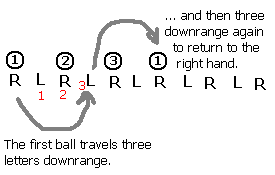Studying the above diagram we can see that the number one ball moves three "hands" down the sequence before landing on the left hand (think of hands as landing sites, hence the use of the word site in the name of the notation). The first ball returns to the right hand after moving three more hands (or sites) rightward in the diagram. Remember, the other balls are following the same pattern, landing three sites down.

The pattern above is called a three-ball cascade. In the cascade pattern above each ball lands on the third open site after being thrown. Mathematically this is recorded as 3 3 3 3 3 3 3 3 3... This means each ball lands on the third site down the diagram. To save space, we toss the spaces and write 33333... For further simplicity we invoke a rule that number patterns repeat indefinitely, hence a single 3 can be used to denote this pattern. Thus 3 means 3333333.... forever.

There are four basic patterns in juggling.

• Cascade: each hand throws in alternation to the other hand in a left-right pattern. In the simplest form, balls all rise to the same height.
• Shower: one hand throws the balls in an arc typically higher than a cascade, while the other hands always catches the balls and passes them back to the first hand. The pass back is barely a throw.
• Columns: the objects are juggled in vertical columns. For three balls, this means that one ball moves between the left and right hand while the other two hop up and down staying always on the left or right.
• Fountain: Done with four or more even numbers of balls, the balls always thrown and caught by the same hand.

Another pattern is the three ball shower, with the balls always being thrown from one hand and then caught and fed back to the first hand in a transfer. The right hand must "launch" the balls in sequence "downrange" to the opposite hand. The first opposite open "site" (hand) that permits a transfer (a throw of 1) to set up the relaunch of ball one is the fifth site.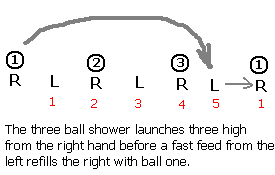Mapping the launches and landings of the other two balls confirms that the balls land "5 site" downrange. This is referred to as a 5 1, or 51 with the spaces removed, with each ball executing the same 51 pattern over and over: 515151...

Other notes:

• Throws alternate hands. The basic site swap system does not take into account synchronous throws.
• A zero is an empty hand.
• Even numbers return to the same hand. A 2 is a ball held in the hand for a "beat", it is not a thrown ball. Think of it as a pause.
• There are n-1 catch/tosses prior to a ball landing.
• Site swap numbers can be conceived of as "beats."
• For the number 3 and above, the height of the throw is related to the number. The relationship is NON-LINEAR.

### The swapping

The notation is called site swap in part because one can "swap" or "switch around" the "sites" (hands!) on which balls land. That it, from a three ball cascade one can toss a ball from the right hand straight up, catching the ball four sites (or four counts or four beats) later. This gives one time to catch and throw a ball from the left hand while the 4 count ball is airborne. While this is happening the left hand holds onto a ball. This is counted as a "2" in the world site swaps (even numbers return to the same hand, holding a ball for an extra beat results in calling this a count of 2).

The diagram depicts a start of a three ball cascade with a single column ball off the right hand followed by a return to a three ball cascade. The notation, unsimplified, would be 33423333...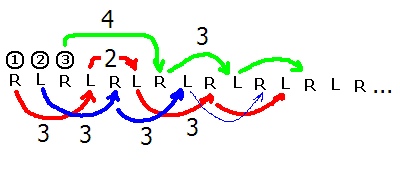## The math

• For any site swap number sequence the average must be equal to the number of balls.
• Two balls must not come down into the same hand at the same time. This rules out sequences such as a 3 2 1 (321). Any n(n-1) pattern lands two balls in one hand. This rule is far harder to check and generally requires making a space-time diagram or analyzing the orbits of individual balls.

Site swap notation is a mathematical model for juggling, another physical system. Juggling ball orbits are predictable and juggling machines have been successfully build without the need for microprocessors or computers. This single variable mathematical system has rules and systems of proof. The intent of this laboratory is expand your thinking, to get you to consider any system that you see as potentially having a mathematical model underneath the system.

To determine the number of balls calculate the average for each sequence. Add the numbers as single digits and then divide by the number of numbers. [Note to real site swappers: this is intended as math exercise and the author does not mean to imply that this condition is by any means sufficient. It is not sufficient. The class will not be checking for multiplex landings, transition issues, or excited states.]

If the mean is fractional, then the pattern does not exist or is incomplete.

In the world of site swap notation "equations" would look like 33342333. The equation can be true or false. 33342333 is a true equation because (3+3+3+4+2+3+3+3)/8 = 24/8 = 3. The result is a whole number. The result is the number of balls.

Thus site swap mathematics has equations, the equations make predictions about a physical system.

### Joggling

Running and juggling is difficult with anything other than patterns that synchronize with the cadence of the runner. Asymmetrical patterns such as 51 are very difficult to maintain while running. Since cadence is essentially a two beat, left right proposition, this author suspects that only patterns of single digits N M where N = M are really functional. This is potentially a massive limitation leaving only simple cascades as a functional possibility.

Science is best learning by doing. In order to "do" site swap equations, you have to learn to juggle. Thus we will tackle learning to perform a simple cascade.

## Equipment

Three balls roughly tennis ball size - bring your own!

## Procedure: Three steps to juggling...

1. First learn to toss one ball back and forth between two hands.
2. Then practice tossing two balls in alternation on a one-two beat from left to right and back again. Do NOT toss both at the same time: wait for the first ball to reach the vertex of its parabola before launching the second ball from the other hand. Practice making both parabolas identical, with a vertex right in front of your forehead.
3. With two balls in your dominant hand, and one in your weak hand, launch ball one from the dominant hand. Wait. Wait. Wait until the ball is at the vertex of the inverted parabola in front of your forehead, then launch the second ball from the left hand under the incoming first ball. Wait for that ball to reach the vertex before launching the third ball under the incoming second ball. The launch is a throw to the inside, causing the vertex of balls thrown by the right hand to peak over the left eye, balls thrown by the left hand should peak over the right eye. Opposite side peak. The most common error is launching balls outside over incoming balls. This is a common difficulty for students who are really good at juggling two mangoes in one hand.

Siteswap FAQ by Allen Knutson
Siteswap from Wikipedia
The science of juggling

# 013 Laboratory one marking rubric

The following rubric indicates how points may determined for laboratory one. The report includes the assessment of grammar, vocabulary, organization, and coherence. Alternate rubrics may be used at the instructor's discretion.

1. [d] Data (often in a table of results) [max:10]
+1length data
+1height data
+1width data
+1volume data
+1mass data
+1per chunk measured up to five chunks
2. [t] Data table format 
+1borders
+1margins, table on page, repeat of headers on new page to control orphans
3. [g] Data display: Graph 
+1Correct xy scattergraph
+1axis labels
+1axis units
+1trend line
+1equation of trend line
4. [a] Data analysis: mathematical and/or statistical analysis 
+1assessment based on appearance whether a linear mathematical relationship exists
+2slope with correct units
+2intercept with correct units

The following are also marked. Refer to the generic rubric for details.

 5. [c] Discussion of results and Conclusions. 6. [f] Format [G] Grammar and Syntax [-2 if conclusion too short to judge grammar properly] [V] Vocabulary [-2 if conclusion too short - taken as evidence of vocabulary limitations] [O] Organization [C] Cohesion [0 if conclusion too short to judge cohesion]

## 023 Laboratory two marking rubric

1. [d] Data table
5Data for five ball roll runs
2. [t] Data table format 
+1borders
+1margins, table on page, repeat of headers on new page to control orphans
3. [g] Data display: Graph
+1Correct xy scattergraph
+1axis labels
+1axis units
+1trend lines
+1equation of trend lines
5. [a] Data analysis: mathematical and/or statistical analysis
5 Five slopes reported from best fit lines on graph

# 033 Laboratory three marking rubric

1. [d] Data (often in a table of results) 
+5drop times including 0, 100, 200, 300, 400, 500
+1squared times
2. [t] Data table format 
+1borders
+1margins, table on page, repeat of headers on new page to control orphans
3. [g] Data display: Quadratic graph 
+1Correct xy scattergraph
+1axis labels
+1axis units
4. [g] Data display: Linear graph 
+1Correct xy scattergraph with x and y axes correct
+1axis labels
+1axis units
+1trend line
+1equation of trend line
5. [a] Data analysis 
+2Value for the experimental acceleration of gravity g [+1] with correct units [+1] (slope of the second graph)
+2Value for the actual acceleration of gravity g [+1] with correct units [+1]
+1Percentage difference from theoretic acceleration of gravity (percent error)
+1Citation of source of theoretic value for the acceleration of gravity g

The following are also marked. Refer to the generic rubric for details.

1. [c] Discussion of results and Conclusions. Conclusion should discuss experimentally obtained value for g, actual value of g, the percentage difference (error), and how good is the agreement between the experimental result and the actual valur for g based on that percentage difference. Option to reduce conclusion by one point per missing element in this list.
2. [f] Format
3. [G] Grammar and Syntax [-2 if conclusion too short to judge grammar properly]
4. [V] Vocabulary [-2 if conclusion too short - taken as evidence of vocabulary limitations]
5. [O] Organization
6. [C] Cohesion [0 if conclusion too short to judge cohesion]

## 043 Laboratory four marking rubric

1. [d] Data table
3Data
2. [t] Data table format 
+1borders
+1margins, table on page, repeat of headers on new page to control orphans
3. [g] Data display: Graph
+1appropriate graph
+1axis labels
+1axis units
+1trend line, if appropriate
+1equation of trend line, if appropriate
4. [a] Data analysis: mathematical and/or statistical analysis
1 Slope reported from best fit line on graph

# 053 Laboratory five marking rubric

1. [d] Data [max 3]
+1per column of correct data 
+1extension first col, force second col
2. [t] Data table format 
+1borders
+1margins, table on page, repeat of headers on new page to control orphans
3. [g] Data display: Graph 
3xy scatter graph with correct axis labels including units
2Missing a label, units for a label, or other single element
1plotting the wrong data, or incomprehensible, wrong graph type, multiple missing elements
4. [a] Data analysis 
+1determination of linearity
+1slope (if determined to be non-linear, then discussion of non-linearity substitutes for slope and units.)
+1units for the slope
+1analyzed sectional linearity, Hookean ranges

The following are also marked. Refer to the generic rubric for details.

 5. [c] Discussion of results and Conclusions. Content should include discussion of the experimentally determined Hooke's constant if one was found, and the linearity or non-linearity of the system. Might include nature of elastic as Hookean only over specific domains and ranges. Discussion of measurement difficulties and errors. Truly excellent research with cited sources and discussion of the the impact of that information on the above system may be awarded additional points. 6. [f] Format [G] Grammar and Syntax [-2 if conclusion too short to judge grammar properly] [V] Vocabulary [-2 if conclusion too short - taken as evidence of vocabulary limitations] [O] Organization [C] Cohesion [0 if conclusion too short to judge cohesion]

# 059 Laboratory 058 marking rubric

1. [d] Data [max 7]
+1per force, load data pair up to five 
+2startValue
2. [i] Image 
4original work and correct
3original work and incorrect
2unoriginal copy and paste from other sources with citation
1unoriginal copy and paste from other sources without citation
2. [t] Data table format 
+1borders
+1margins, table on page, repeat of headers on new page to control orphans
3. [g] Data display: Graph 
+1correct xy scattergraph
+1axis labels on both axes
+1axis units
+1linear regression trendline displayed
+1linear regression trendline equation displayed
4. [a] Data analysis 
+1determination of linearity
+1efficiency of the pulley system

The following are also marked. Refer to the generic rubric for details.

 5. [c] Discussion of results and Conclusions. Discussion should include the efficiency and what factors affect the efficiency of the pulley system. 6. [f] Format [G] Grammar and Syntax [-2 if conclusion too short to judge grammar properly] [V] Vocabulary [-2 if conclusion too short - taken as evidence of vocabulary limitations] [O] Organization [C] Cohesion [0 if conclusion too short to judge cohesion]

## 063 Laboratory six marking rubric

1. [d] Data table
3Data
2. [t] Data table format 
+1borders
+1margins, table on page, repeat of headers on new page to control orphans
3. [g] Data display: Graph
3Correct axis labels
2Missing a label or other element
1 plotting the wrong data, or wrong result on graph, or otherwise incorrect
4. [a] Graph choice is appropriate, shows thoughtful analysis of how to best represent the data in a meaningful way.
3Good design choices
2Fair design choices
1Poor design choices

# 073 Laboratory seven marking rubric

1. [d] Data 
+1per coordinate pair
2. [t] Data table format 
+1borders
+1margins, table on page, repeat of headers on new page to control orphans
3. [g] Data display: Graph 
+1correct xy scattergraph
+1axis labels on both axes
+1axis units
+1linear regression trendline displayed
+1linear regression trendline equation displayed
4. [a] Data analysis 
+2Calculated slope including reporting the units
+1Latitude/longitude which was held constant
+1Start value longitude/latitude

The following are also marked. Refer to the generic rubric for details.

 5. [c] Discussion of results and conclusions including relating how the measurements in the laboratory relate to the hide and seek exercise. 6. [f] Format [G] Grammar and Syntax [-2 if conclusion too short to judge grammar properly] [V] Vocabulary [-2 if conclusion too short - taken as evidence of vocabulary limitations] [O] Organization [C] Cohesion [0 if conclusion too short to judge cohesion]

# 083 Laboratory eight drawing rubric

1. Accuracy of shapes, forms, and colors
5The shapes are represented with outstanding form, the colors are accurate, all contours of the shapes are accurate, light source is discernible
4The shapes are represented with good form, the colors are accurate, most contours of the shapes are accurate, light source is discernible
3The shapes are represented with good form, the colors are close to accurate, many contours of the shapes are accurate
2The shapes need more form, the colors are limited in their accuracy, many contours of the shapes are missed
1No effort was made to complete the drawing with good form and accurate color choices; contours bear little resemblance to actual shape
2. Composition
5The composition of the drawing uses the size of the paper to create very good balance of the drawing to the background, or shows creative cropping, well reflects what is seen
4The composition of the drawing uses the size of the paper to create good balance of the drawing to the background, well reflects what is seen
3The composition of the drawing uses the size of the paper to create a centered drawing with slightly too much background, some changes from what is seen
2The composition of the drawing uses the size of the paper to create a centered drawing but too much background becomes distracting; not seeing details as one moves closer
1The composition of the drawing used the wrong size of paper, or was not planned out, the composition reflects no balance or unity, or the amount of blank background around the drawing is excessive.
3. Consistency in drawing style
5The drawing style used is consistent/works well together throughout; the art media provided is used to its full potential, careful attention to the use of the media is seen in the drawing
4The drawing style used is consistent; the art media provided is used well; the drawing style adds a cohesiveness to the drawing; minimal carelessness
3The drawing style used is not distracting to the subject; the art media provided is used moderately well, the drawing style is consistent; some carelessness
2The drawing style used is distracting to the subject, the style changes throughout the image, needs more practice with the art media provided, much carelessness
1There is no attempt to use a cohesive drawing style, or no drawing style in evidence; needs improvement
4. Effort/Craftsmanship
5The project was continued until it was as complete as the student could make it, effort beyond that required, took pride in work
4The student worked hard and completed the project, but with a little more effort it might have been outstanding
3The student finished the project; could have been improved with more effort
2The project was completed with minimal effort, chose an easy solution and did it indifferently
1The student did not finish the work adequately

# 093 Laboratory nine marking rubric

1. [d] Data [variable]
+1per location
+1dry bulb temperature reported
+1wet bulb temperature reported
2. [t] Data table format 
+1borders
+1margins, table on page, repeat of headers on new page to control orphans
3. [g] Data display: Graph 
+1Correct xy scatter graph type
+1axis labels
+1axis units
+1trend line
+1trend line equation
4. [a] Data analysis 
+2experimental speed of sound with units
+2theoretic speed of sound with units, based on temperature and humidity
+1relative humidity reported
+1error analysis

The following are also marked. Refer to the generic rubric for details.

 5. [c] Discussion of results and conclusions. [max 2 if analysis is incomplete] 6. [f] Format [G] Grammar and Syntax [-2 if conclusion too short to judge grammar properly] [V] Vocabulary [-2 if conclusion too short - taken as evidence of vocabulary limitations] [O] Organization [C] Cohesion [0 if conclusion too short to judge cohesion]
 Up to 5 Favored color discussion. 5: Thorough, personal, exceptionally well developed. 4: Well developed, good. 3: Fair. Done but with a minimal level of completeness. 2: Weakly done. Little detail. 1: Exceptionally weak. Up to 5 Least favored color discussion. See above. 5 Grammar, syntax 5 Vocabulary 5 Organization: both favored and least favored covered. 5 Cohesion (0 if too short to judge)

# 113 Laboratory eleven marking rubric

1. [d] Data tables content [5+5]
+1per reflection/refraction data row (min five data points)
2. [t] Reflection table format 
+1borders
+1margins, table on page, repeat of headers on new page to control orphans
3. [g] Reflection graph format 
+1Correct xy scattergraph
+1axis labels
+1axis units
+1trend line
+1equation of trend line
2. [t] Refraction table format 
+1borders
+1margins, table on page, repeat of headers on new page to control orphans
-2Reversal of image and object data columns. For slope to be index of refraction, x-axis must be image depth and y-axis must be object depth. Table columns must reflect this order or the graph will be incorrect.
5. [g] Refraction graph format 
+1Correct xy scattergraph
+1axis labels
+1axis units
+1trend line
+1equation of trend line
6. [a] Data analysis: mathematical and/or statistical analysis. 
+1reflection slope calculated
+1refraction slope calculated
+1refraction percentage difference from theoretic value calculated

The following are also marked. Refer to the generic rubric for details.

 7. [c] Discussion of results and conclusions. 8. [f] Format [G] Grammar and Syntax [-2 if conclusion too short to judge grammar properly] [V] Vocabulary [-2 if conclusion too short - taken as evidence of vocabulary limitations] [O] Organization [C] Cohesion [0 if conclusion too short to judge cohesion]

Instructional note: Groups of two are recommended and work well for this laboratory. At this point in the term some students become overly reliant on one person gathering the data and then copying the data from that one person, even tossing out their own measurements in favor of those from another group. If this issue develops, then one can assign uniqueness of data points as appropriate. The refraction data is useful for detecting this sort of activity - the position of the image as seen by different observers is often different for each observer. An example is provided below.

1. [u] Uniqueness [max 5, typically 3]
5Only one person reported the refraction data
4Two people reported the same refraction data
3Three people reported the same refraction data
2Four people reported the same refraction data
1Five or more people reported the same refraction data

# 123 Laboratory twelve marking rubric

1. [i] Images, sketches, or drawing [3 + 1]
+1open circuit
+1closed circuit
+1short circuit
+1quality point for high quality drawings
2. [if] Image formatting 
3image, sketch, or drawing that is clear and informative with correct labels OR image fully appropriate to laboratory, original, explanatory, captions as appropriate, and distinguishes bulb base components accurately
2Sketch is clear and complete, only a minor issues such as a mislabel, unoriginal, or lack of a necessary caption
1Sketch is confusing, unclear, not well done, or inappropriate image, image copied from Internet without citation of source
3. [dc] Data tables content conductors 
+1electrical conduction data
+1heat conduction data
4. [tc] Conductors table format and layout 
3Clear, concise, well thought out, informative. Includes both electricity and heat, both clearly labeled.
2Missing borders or other minor format inconsistencies, or missing either heat or electricity data, or confusing, or unclear, or uses two tables or other less efficient presentations such as text, or lack of appropriate labels makes single table unclear
1Incomplete, runs off edges of page, lacks minimal margins
5. [dΩ] Data tables content current versus voltage: ohms 
+1per amperage, voltage coordinate pair [(0, 0) is a theoretic construct and was not measured, therefore not counted in analysis. Typically discussed on lab hand-back.]
6. [tΩ] Table format current versus voltage 
+1borders
+1margins, table on page, repeat of headers on new page to control orphans
7. [g] Current versus voltage graph format 
+1Correct xy scattergraph with current versus voltage
+1axis labels
+1axis units
+1trend line
+1equation of trend line
8. [a] Data analysis 
+1slope
+1units of slope reported as being ohms

# 133 Laboratory thirteen marking rubric

1. [d] Phase II data table content 
+1Flower name
+1Original floral fluid color
+1Acid color change
+1Basic color change
2. [t] Phase II data table format
3Clear, concise, well thought out, informative
2Missing borders or other minor format inconsistencies
1Incomplete, runs off edges of page, lacks minimal margins
0Omitted
3. [a] Phase III analysis of household substances in a table [max: 10]
+1Per household substance correctly classified as acid, base, or neutral
4. [at] Phase III analysis table format
3Clear, concise, well thought out, informative
2Missing borders or other minor format inconsistencies
1Incomplete, runs off edges of page, lacks minimal margins
0Omitted

The following are also marked. Refer to the generic rubric for details.

 3. [c] Discussion of results and conclusions. Content should include most distinct color change for the known acid, most distinct color change for a base. Discuss any difficulties you encountered. 4. [f] Format [G] Grammar and Syntax [-2 if conclusion too short to judge grammar properly] [V] Vocabulary [-2 if conclusion too short - taken as evidence of vocabulary limitations] [O] Organization [C] Cohesion [0 if conclusion too short to judge cohesion]